# [R Course] Data Visualisation with R: Options

Learn how to use the multitude options included in the ggplot2 package such as themes, scales, axes, legends, and facetting.

08-06-2019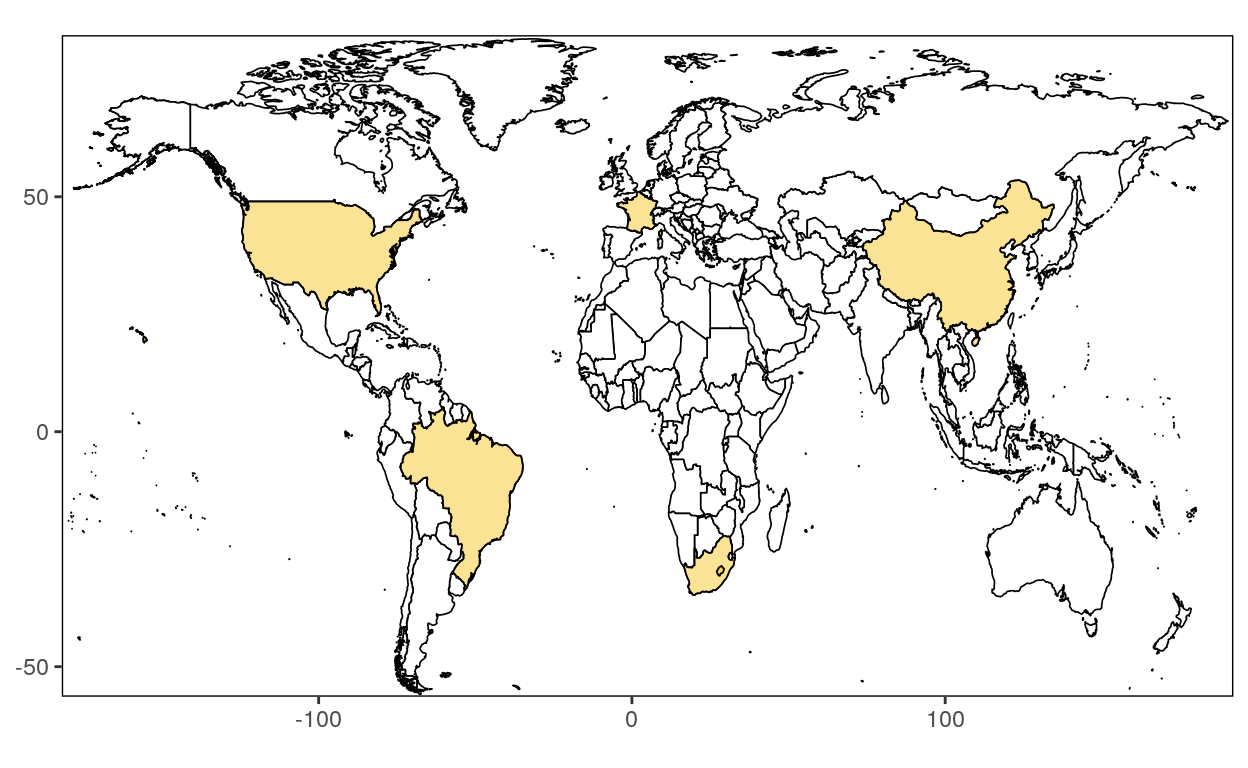## Set up

We will work with data from UNIDO and US data from map_data.

### Data

You can load UNIDO data stored in a Gsheet by using the following code!

``````library(gsheet)

``````

We want to create a column with random data from a sample of 1 to 500.

``````dataGraph\$sample <- sample(1:500, 18, replace=F)
``````

You can load US data from map_data by using the following code!

``````library(ggmap)

usa <- map_data("usa")
``````

### Package

For the examples to work, we need to load the `ggplot2` package.

``````library(ggplot2)
``````

## Themes

Themes control the display of all non-data elements of the plot. You can override all settings with a complete theme like theme_bw(), or choose to tweak individual settings by using theme() and the element_ functions. Use theme_set() to modify the active theme, affecting all future plots.

### Default

The default themes:

``````ggplot(usa, aes(x = long, y = lat, group = group)) +
geom_polygon() +
theme_grey()
````````````ggplot(usa, aes(x = long, y = lat, group = group)) +
geom_polygon() +
theme_gray()
``````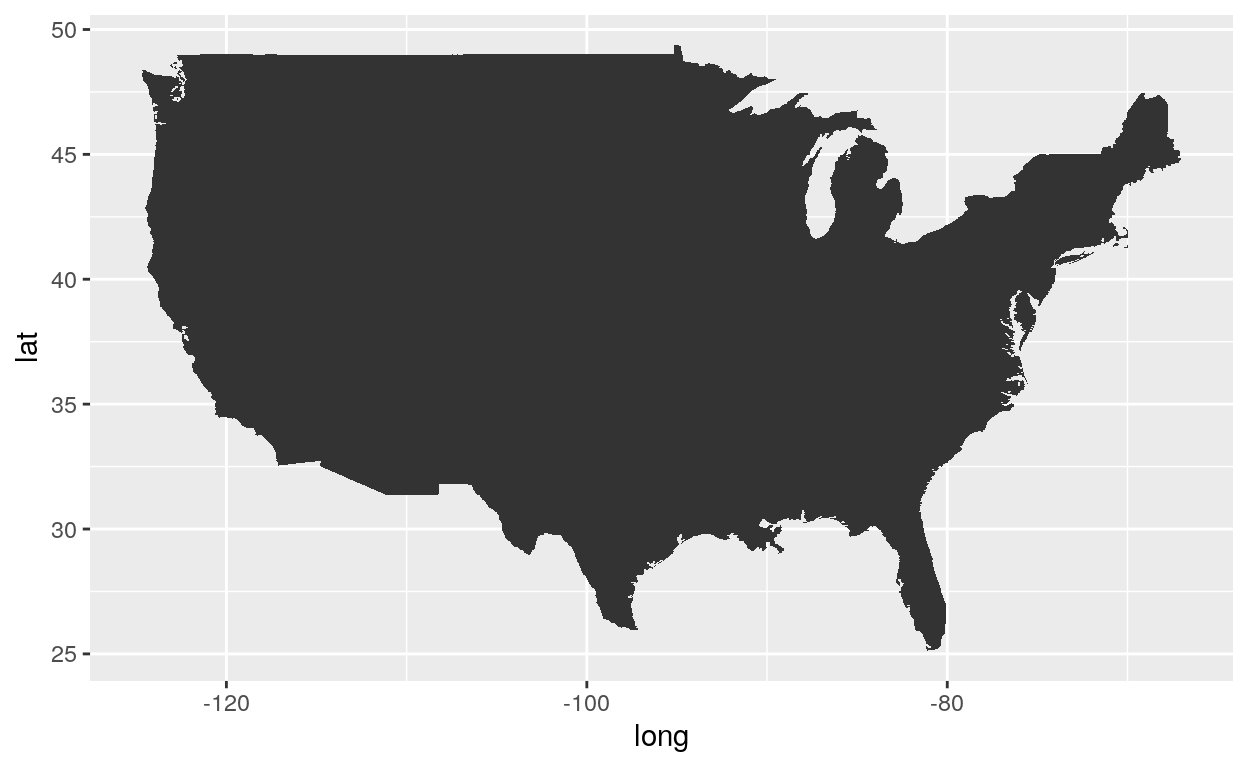### BW

``````ggplot(usa, aes(x = long, y = lat, group = group)) +
geom_polygon() +
theme_bw()
``````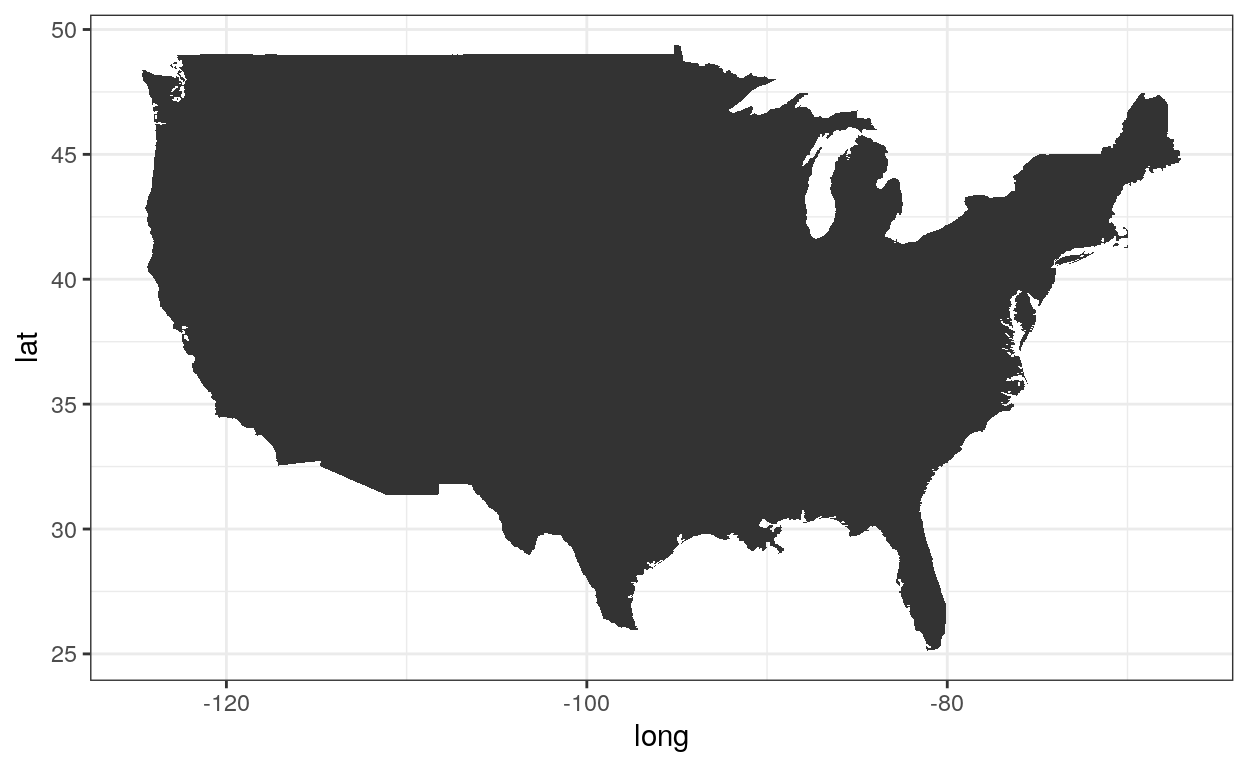### Linedraw

``````ggplot(usa, aes(x = long, y = lat, group = group)) +
geom_polygon() +
theme_linedraw()
``````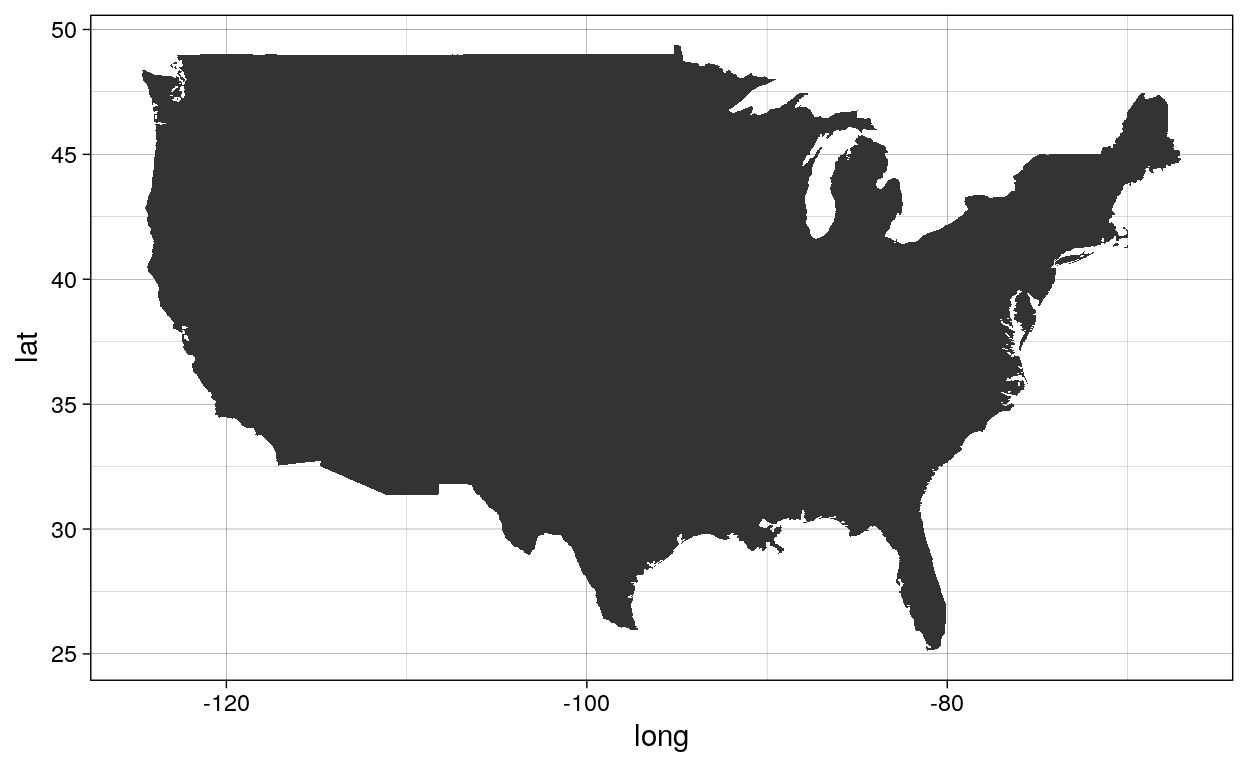### Light

``````ggplot(usa, aes(x = long, y = lat, group = group)) +
geom_polygon() +
theme_light()
``````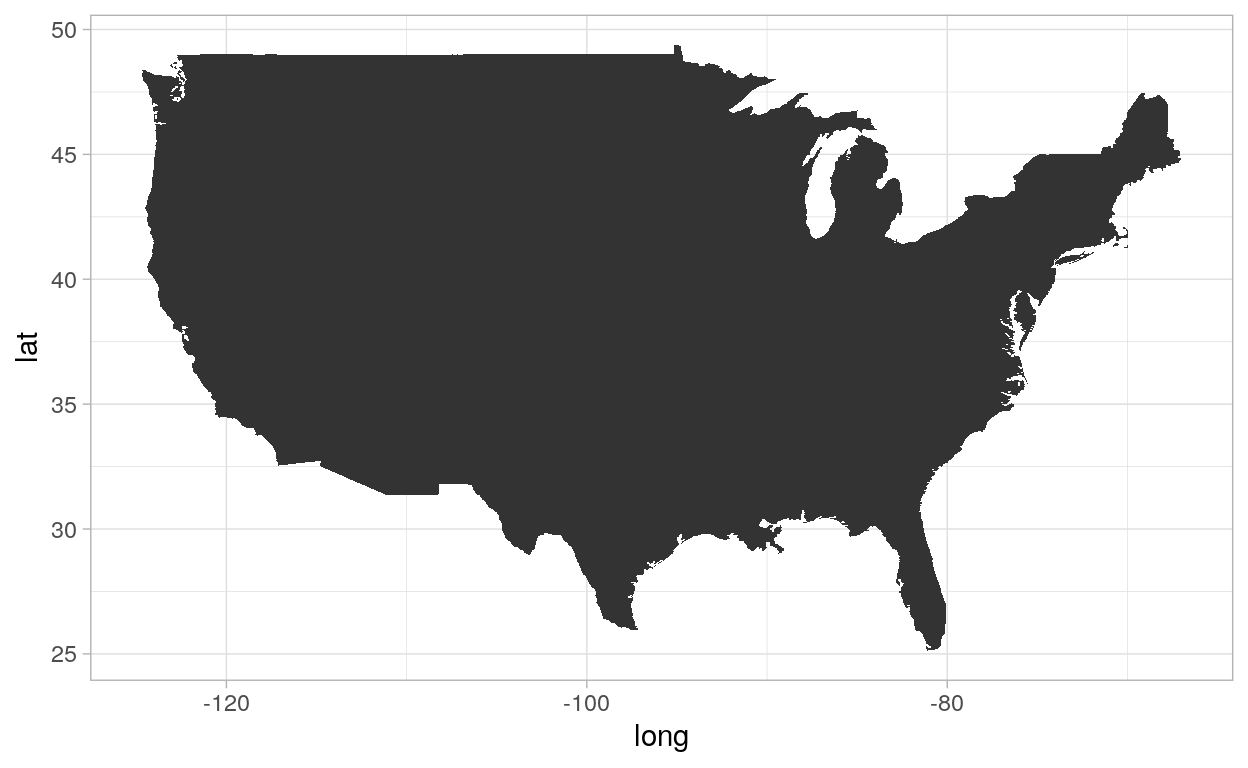### Dark

``````ggplot(usa, aes(x = long, y = lat, group = group)) +
geom_polygon() +
theme_dark()
``````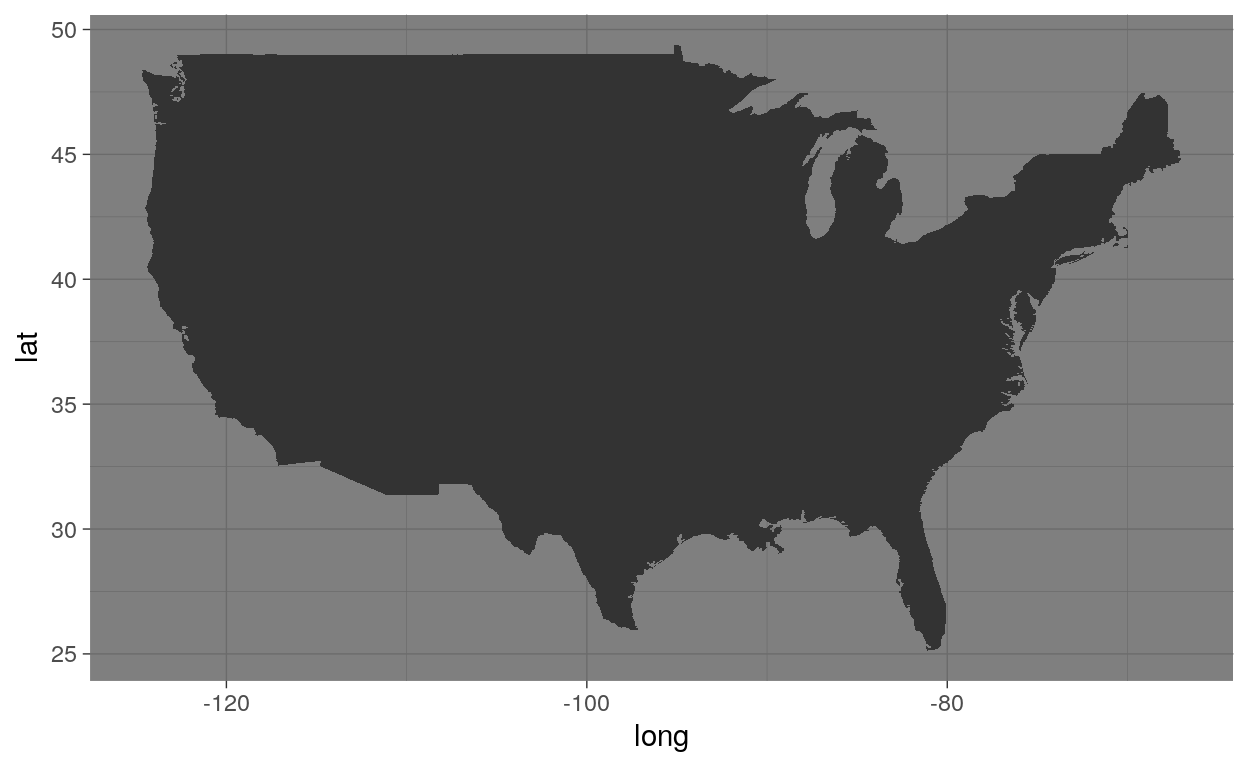### Minimal

``````ggplot(usa, aes(x = long, y = lat, group = group)) +
geom_polygon() +
theme_minimal()
``````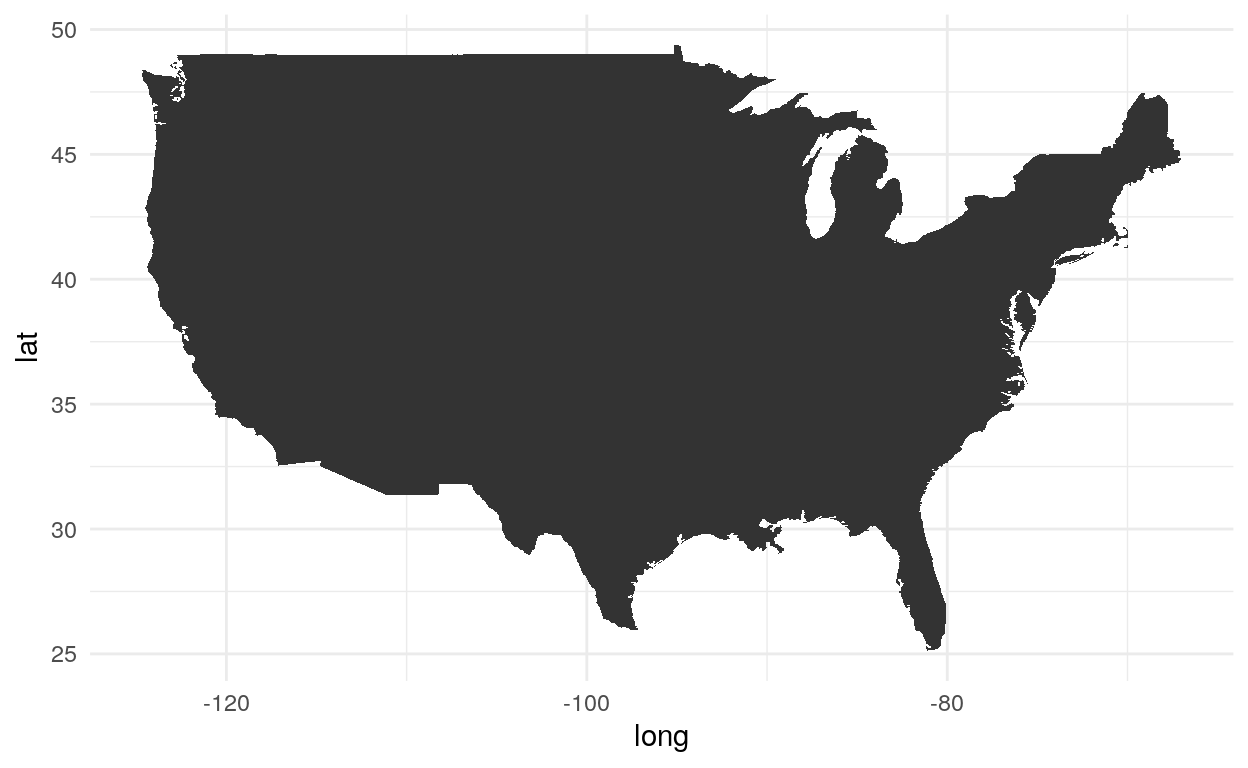### Classic

``````ggplot(usa, aes(x = long, y = lat, group = group)) +
geom_polygon() +
theme_classic()
``````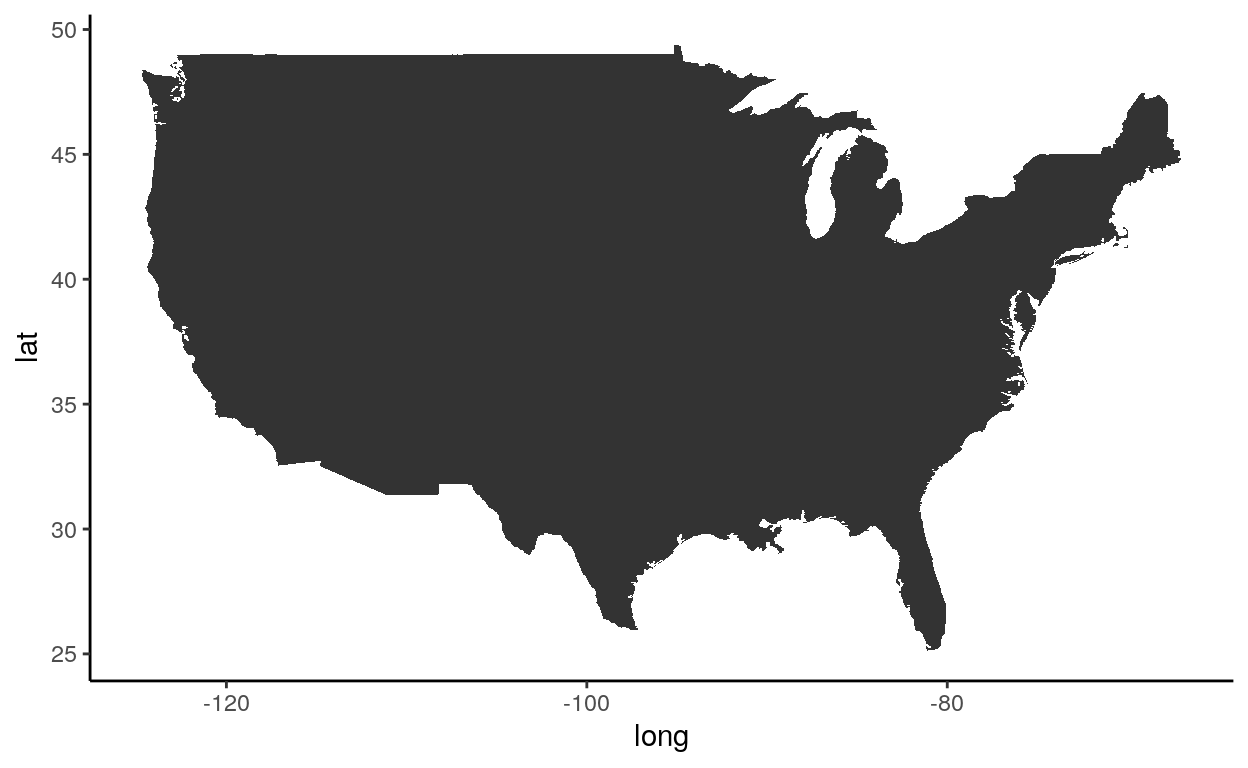### Void

``````ggplot(usa, aes(x = long, y = lat, group = group)) +
geom_polygon() +
theme_void()
``````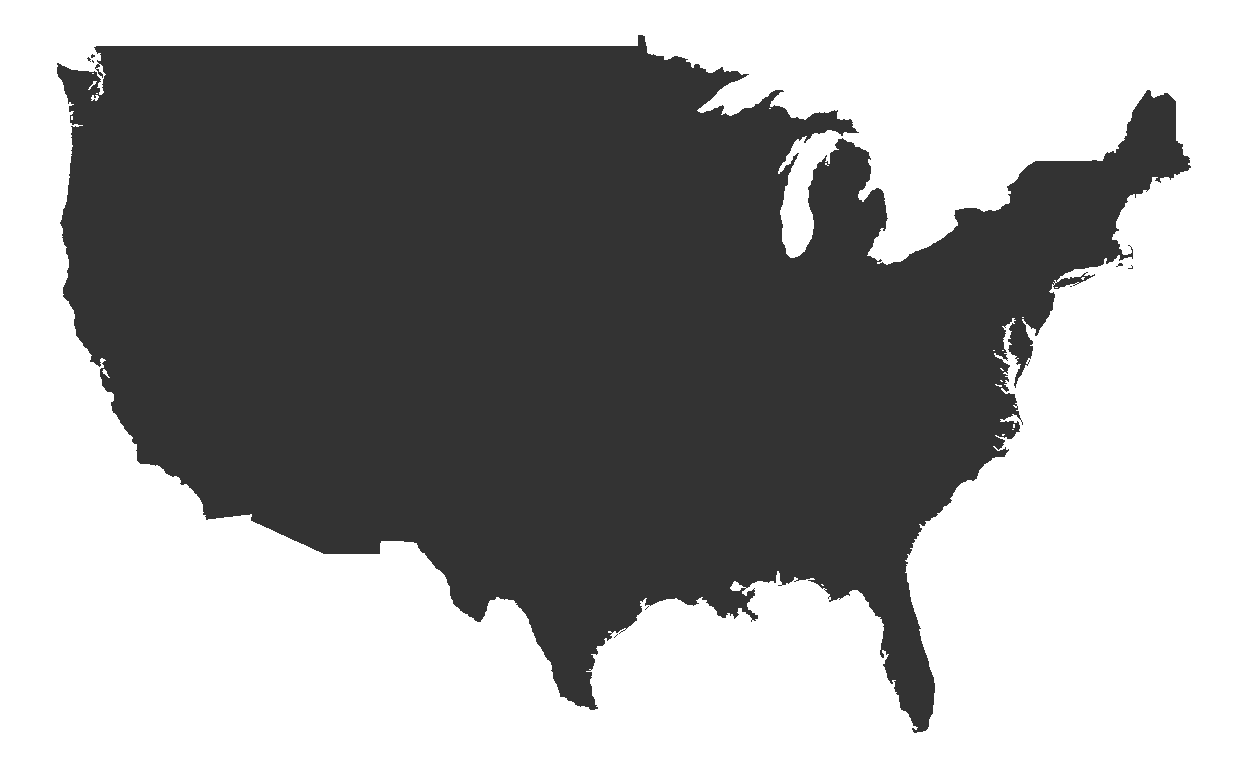### Economist

``````library(ggthemes)
``````
``````ggplot(usa, aes(x = long, y = lat, group = group)) +
geom_polygon() +
theme_economist()
``````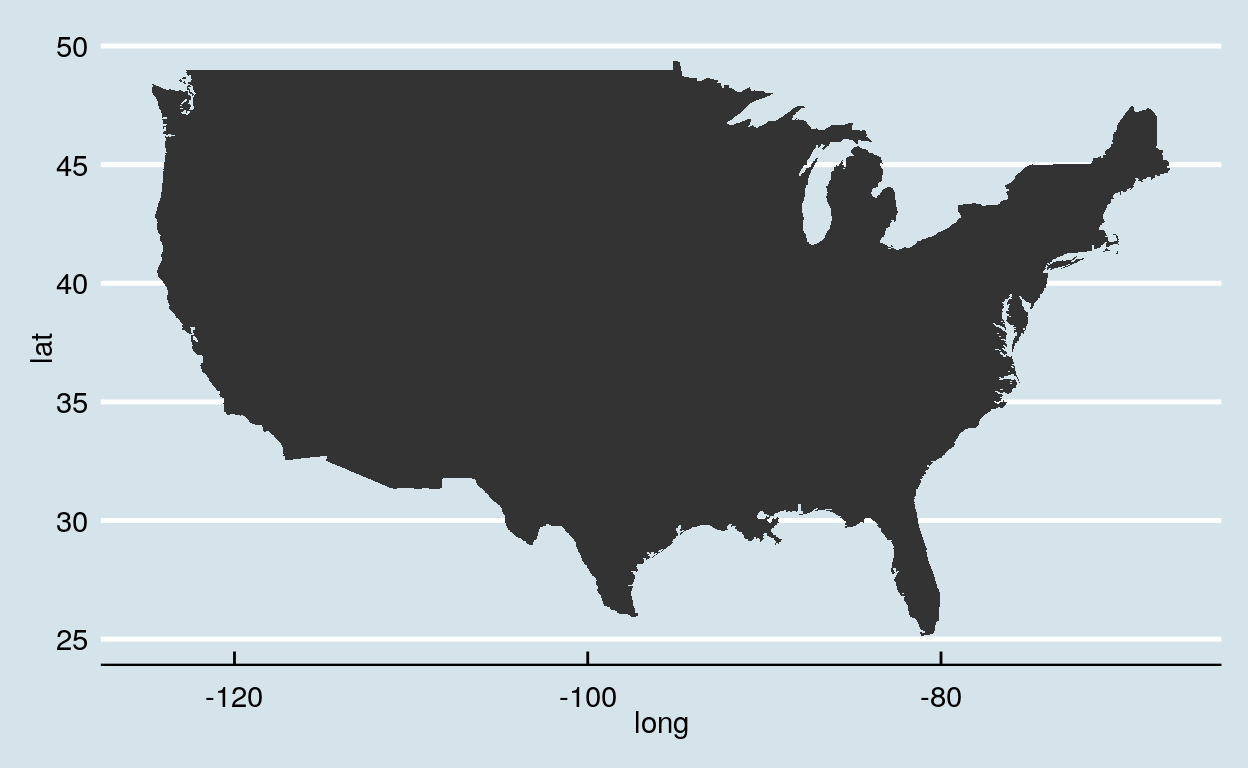Adding the `scale_color_economist()` function to color points with the economist theme colors.

``````ggplot(dataGraph, aes(date, GDP, color = country)) +
geom_point() +
theme_economist() +
scale_color_economist()
``````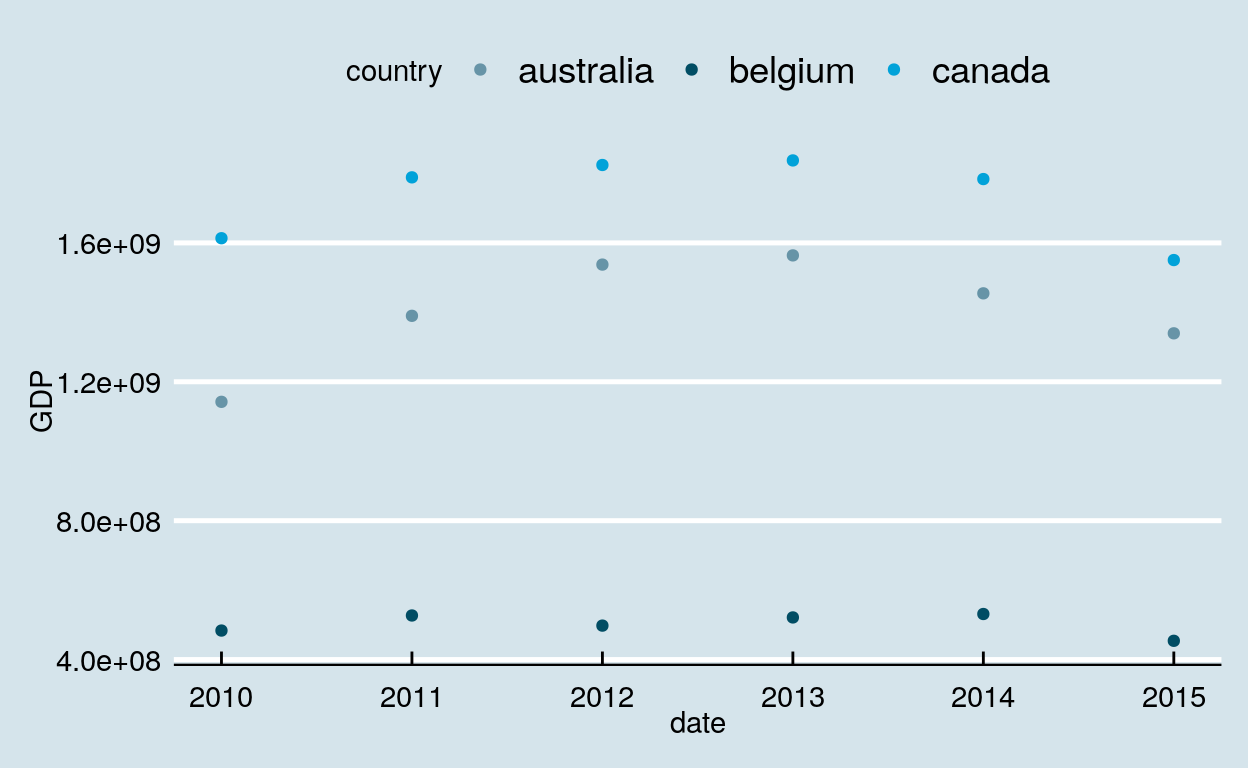### Stata

``````ggplot(dataGraph, aes(date, GDP, color = country)) +
geom_point() +
theme_stata() +
scale_color_stata()
``````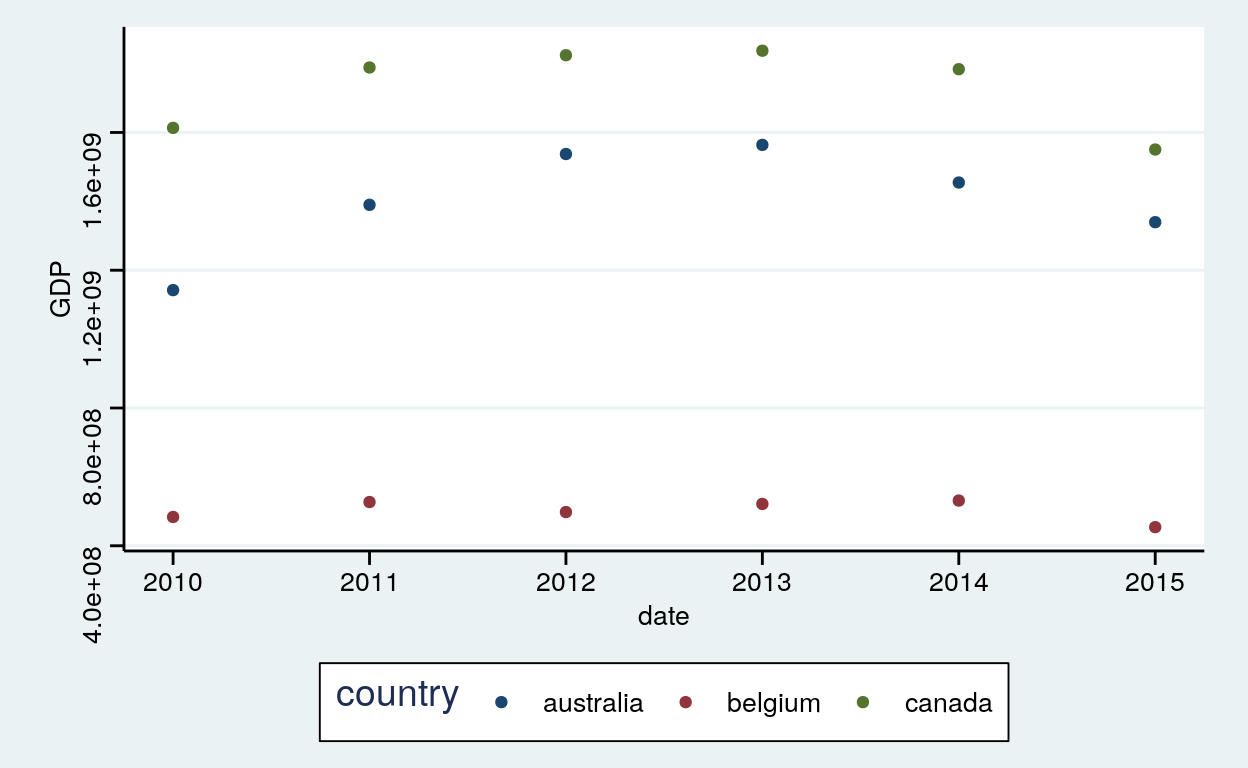### Wall Street Journal

``````ggplot(dataGraph, aes(date, GDP, color = country)) +
geom_point() +
theme_wsj() +
scale_colour_wsj("colors6")
``````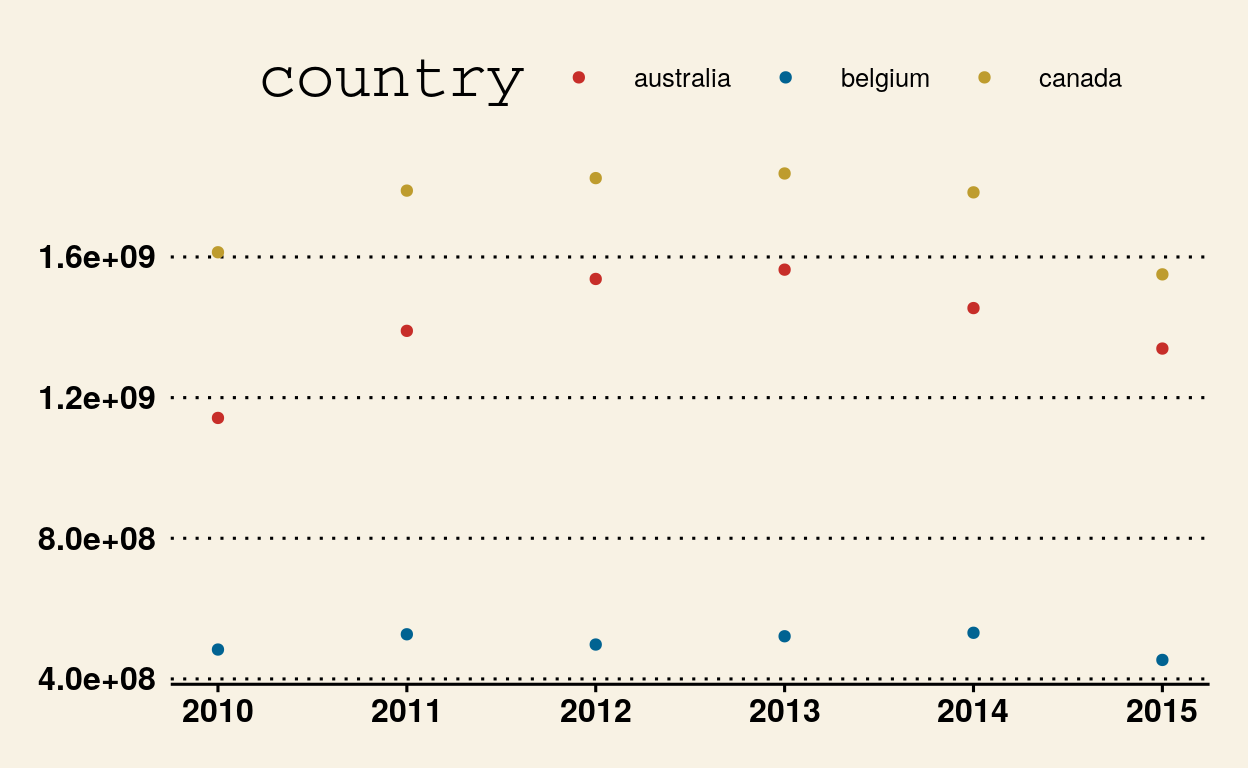### LibreOffice Calc

``````ggplot(dataGraph, aes(date, GDP, color = country)) +
geom_point() +
theme_calc() +
scale_colour_calc()
``````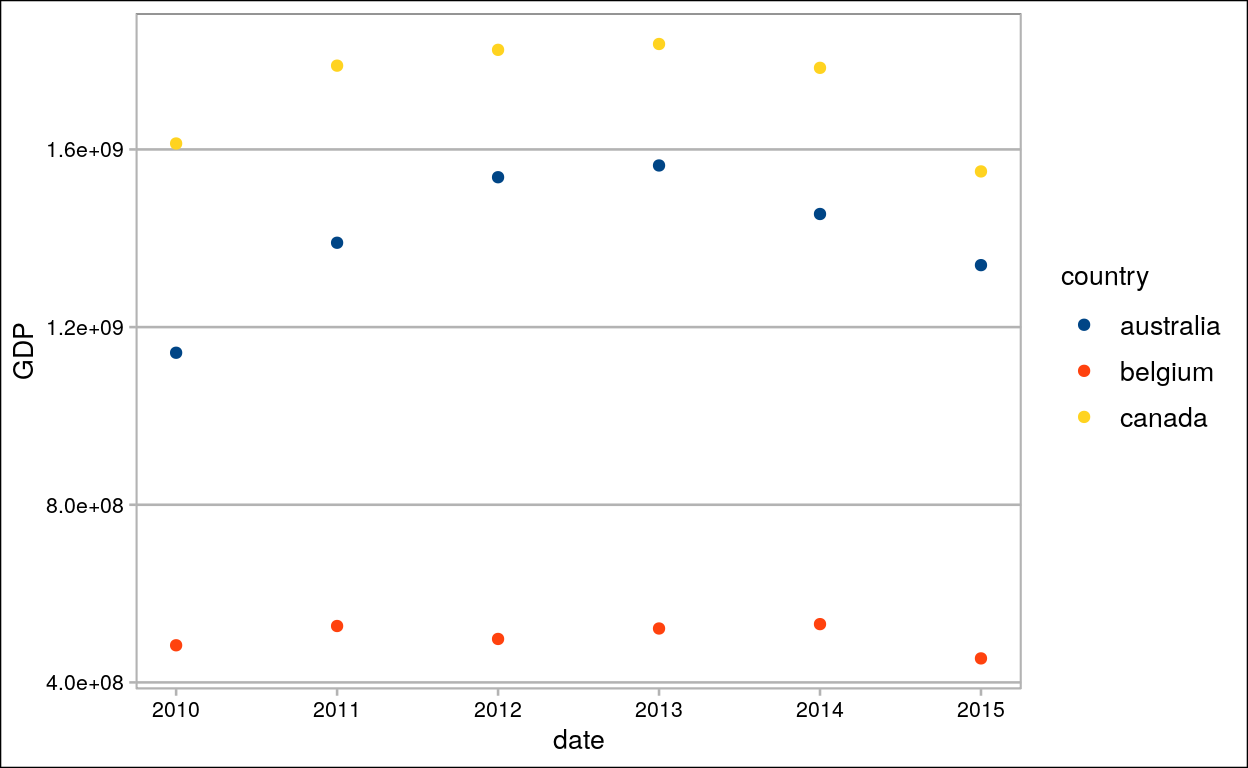## Modify components of a theme

### Labs

#### Title, subtitle, x, y, coulour, shape, caption and tag

``````ggplot(dataGraph, aes(date, GDP)) +
geom_point(aes(colour = country, shape = section), size = 3) +
labs(title = "Gross Domestic Product by Countries",
subtitle = "(2010 - 2015)",
x = "Years",
y = "Gross domestic product",
colour = "Countries",
shape = "Sections",
caption = "Source: Nüance-R",
tag = "A")
``````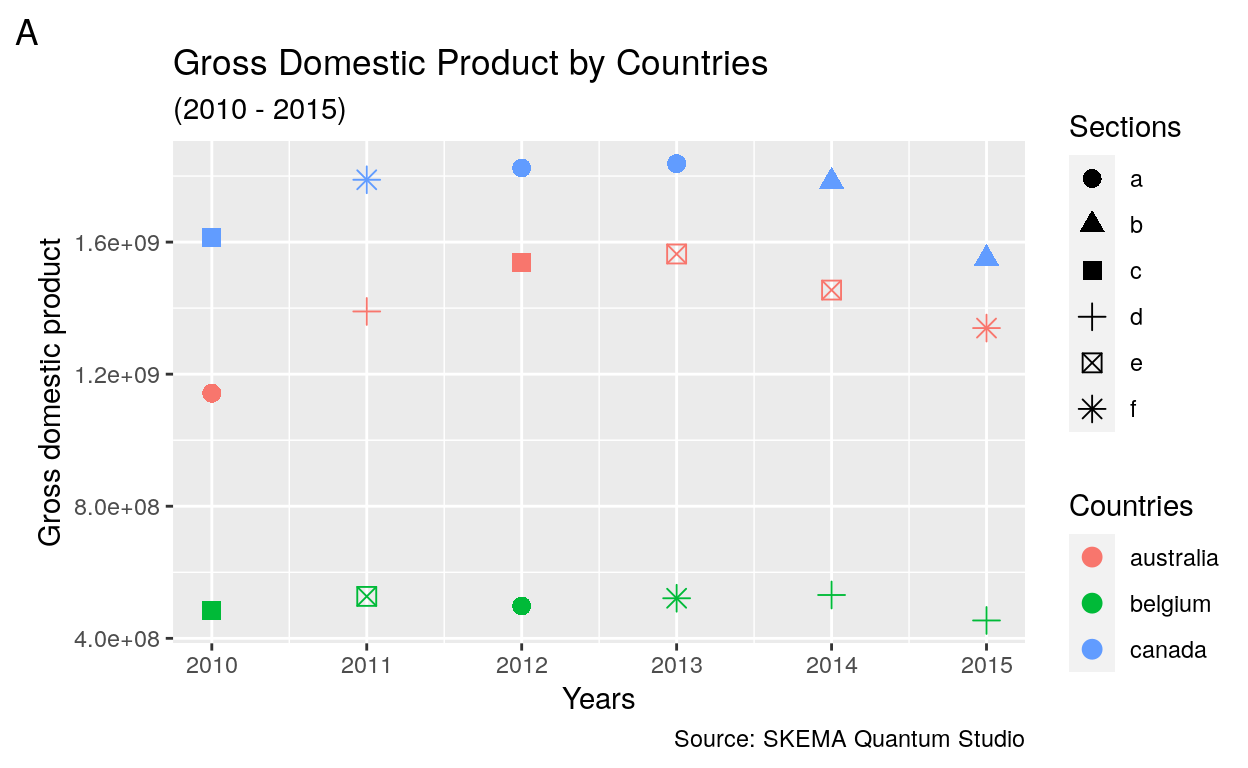### Theme

#### Plot

##### Title
``````ggplot(dataGraph, aes(date, GDP)) +
geom_point(aes(colour = country, shape = section), size = 3) +
labs(title = "Gross Domestic Product by Countries") +
theme(plot.title = element_text(size = 20))
``````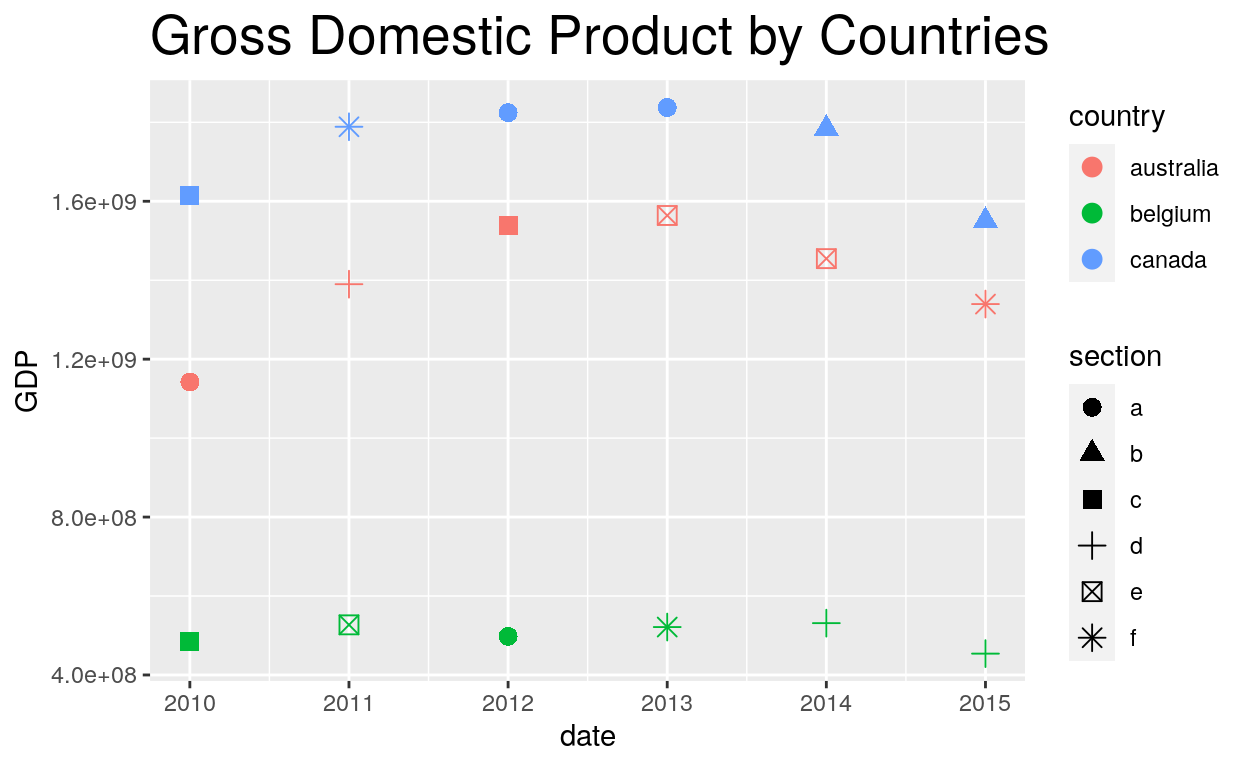##### Background
``````ggplot(dataGraph, aes(date, GDP)) +
geom_point(aes(colour = country, shape = section), size = 3) +
labs(title = "Gross Domestic Product by Countries") +
theme(plot.title = element_text(size = 20),
plot.background = element_rect(fill = "gold"))
``````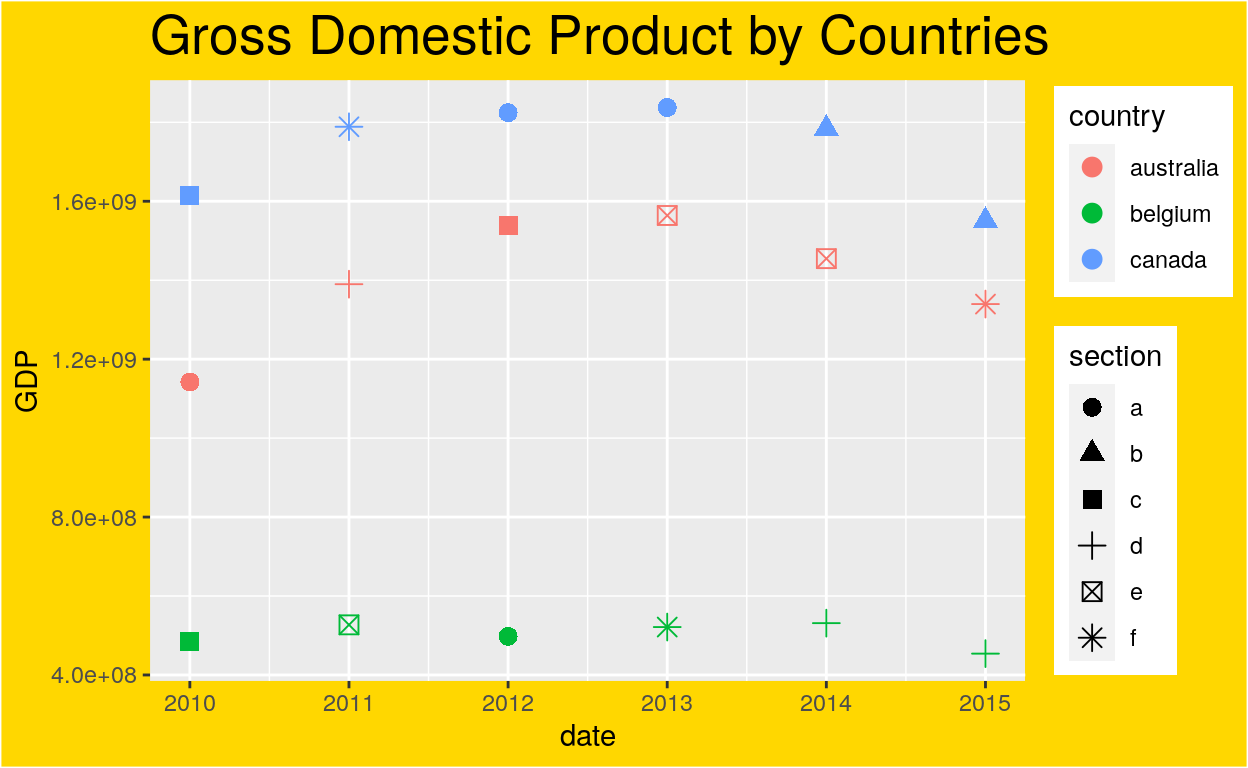#### Legend

###### Position
``````ggplot(dataGraph, aes(date, GDP)) +
geom_point(aes(colour = country, shape = section), size = 3) +
theme(legend.position = "none")
``````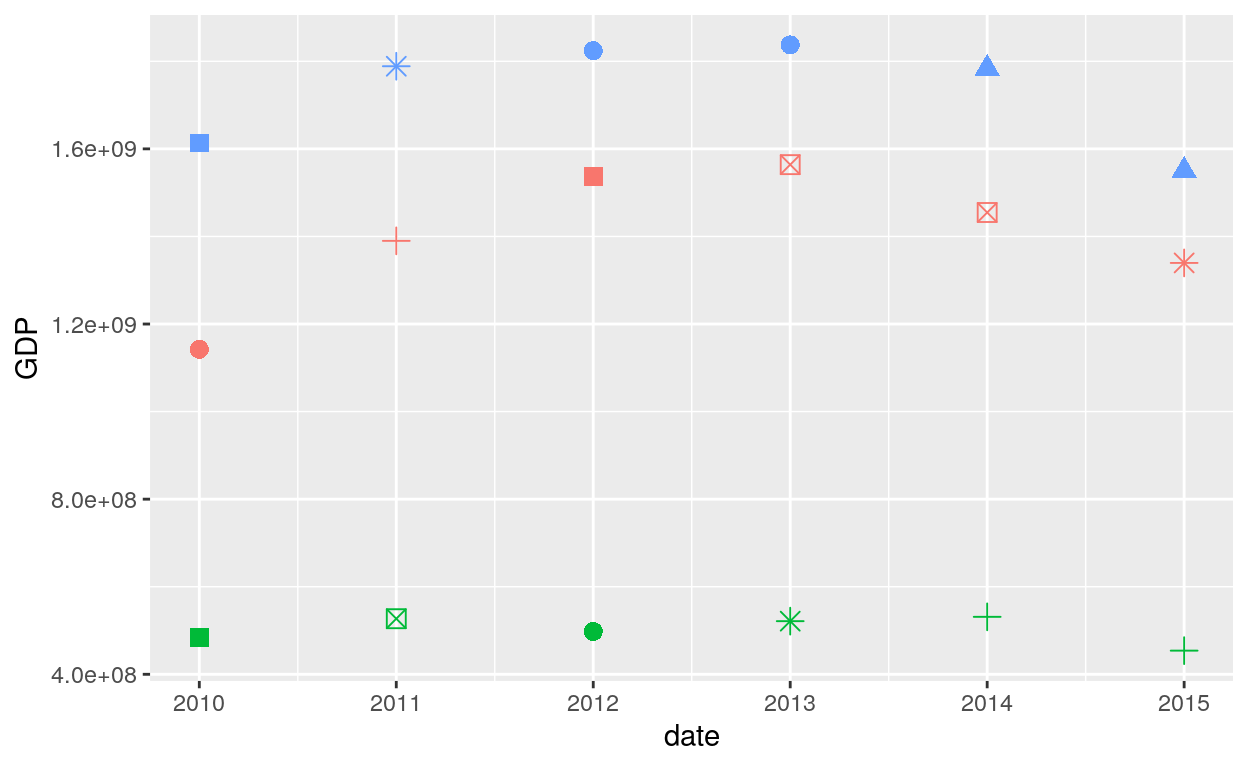``````ggplot(dataGraph, aes(date, GDP)) +
geom_point(aes(colour = country, shape = section), size = 3) +
theme(legend.position = "bottom")
``````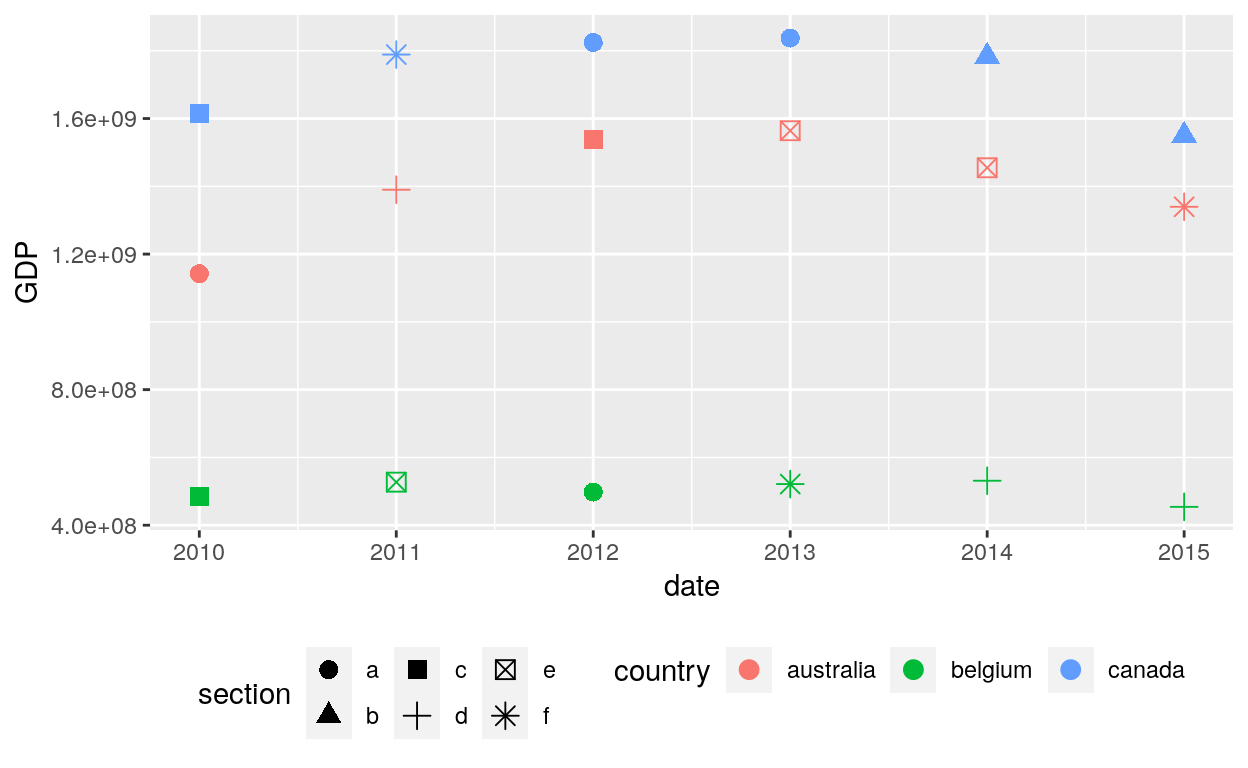``````ggplot(dataGraph, aes(date, GDP)) +
geom_point(aes(colour = country, shape = section), size = 3) +
labs(title = "Gross Domestic Product by Countries") +
theme(legend.position = "left")
``````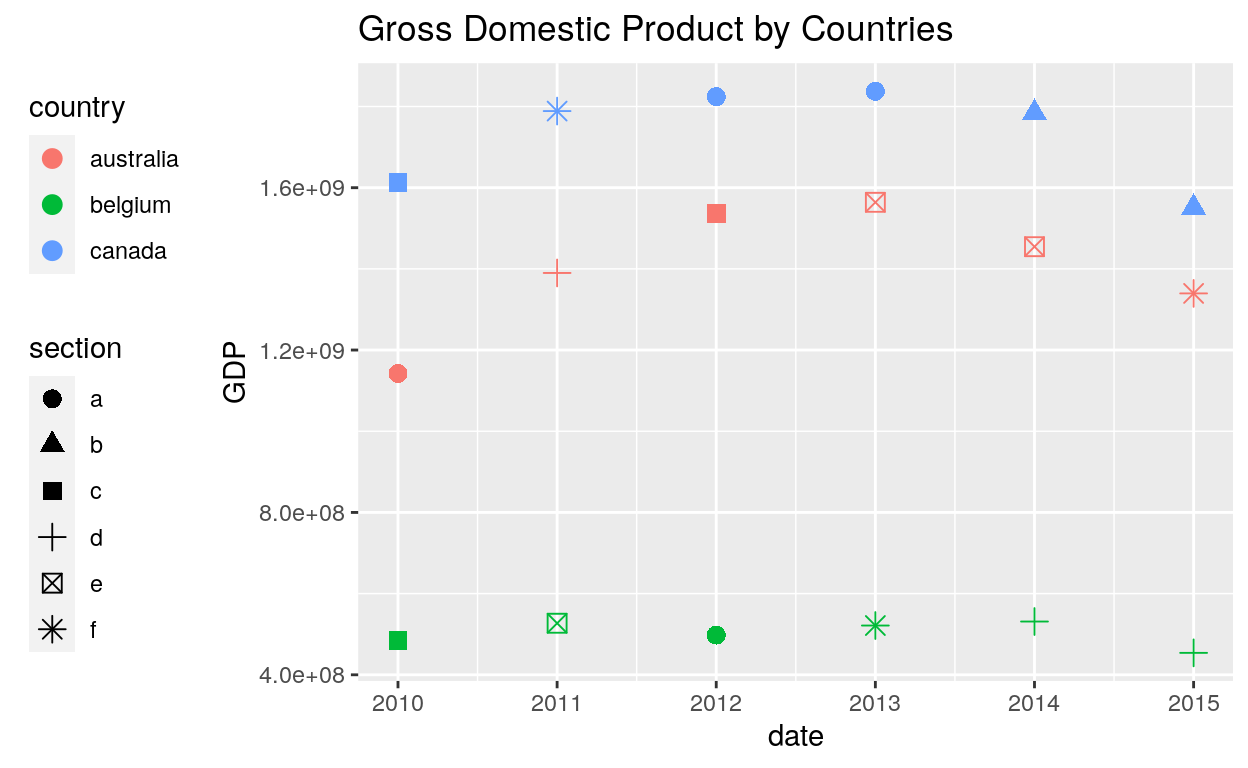###### Justification, Box, Margin
``````# Or place legends inside the plot using relative coordinates between 0 and 1
# legend.justification sets the corner that the position refers to
ggplot(dataGraph, aes(date, GDP)) +
geom_point(aes(colour = country, shape = section), size = 3) +
labs(title = "Gross Domestic Product by Countries") +
theme(legend.position = c(.95, .95),
legend.justification = c("right", "top"),
legend.box.just = "right",
legend.margin = margin(6, 6, 6, 6))
``````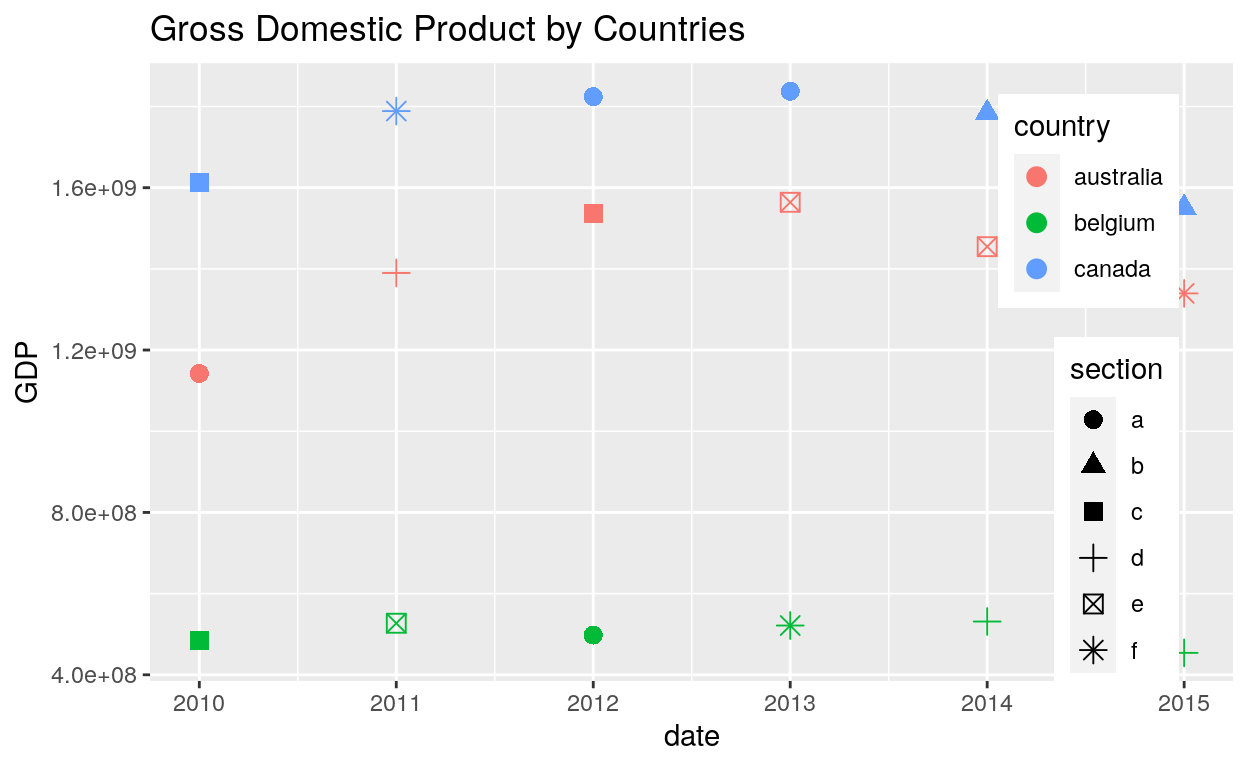###### Box background and margin
``````# The legend.box properties work similarly for the space around all the legends
ggplot(dataGraph, aes(date, GDP)) +
geom_point(aes(colour = country, shape = section), size = 3) +
labs(title = "Gross Domestic Product by Countries") +
theme(legend.box.background = element_rect(),
legend.box.margin = margin(6, 6, 6, 6))
``````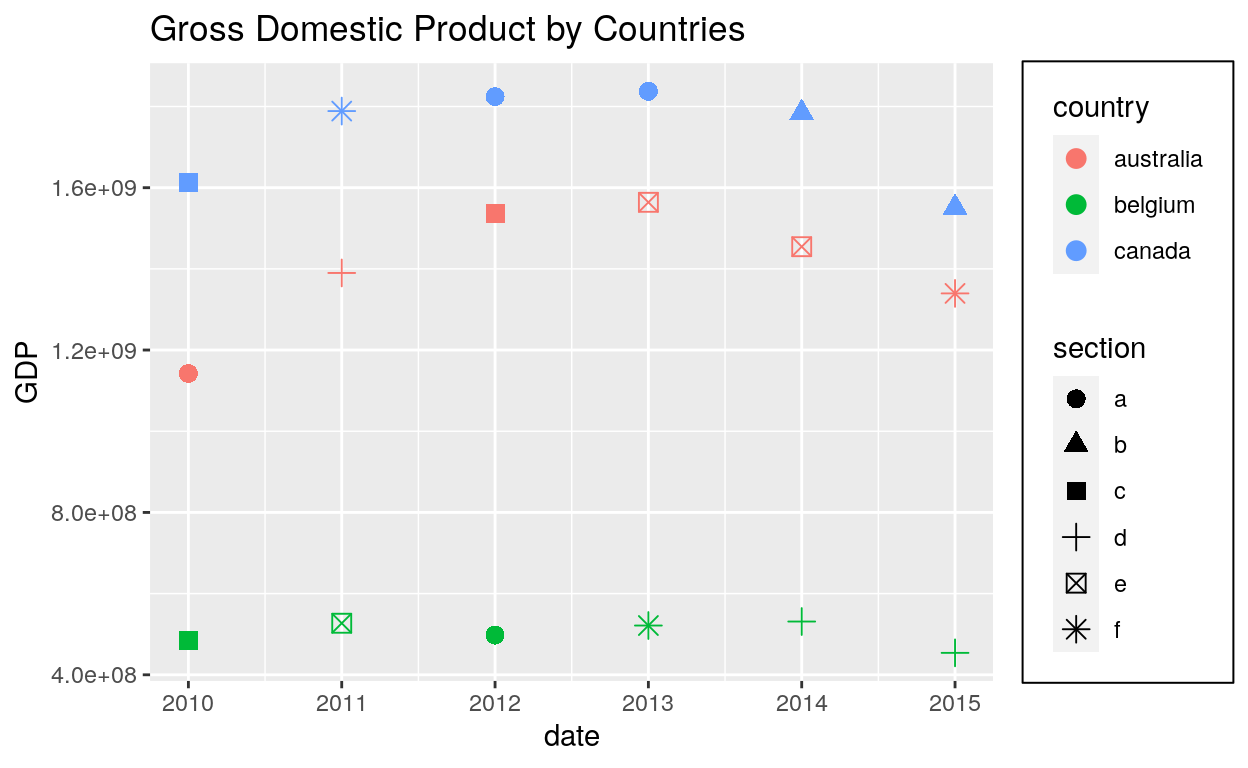###### Key
``````ggplot(dataGraph, aes(date, GDP)) +
geom_point(aes(colour = country, shape = section), size = 3) +
labs(title = "Gross Domestic Product by Countries") +
theme(legend.key = element_rect(fill = "white", colour = "black"))
``````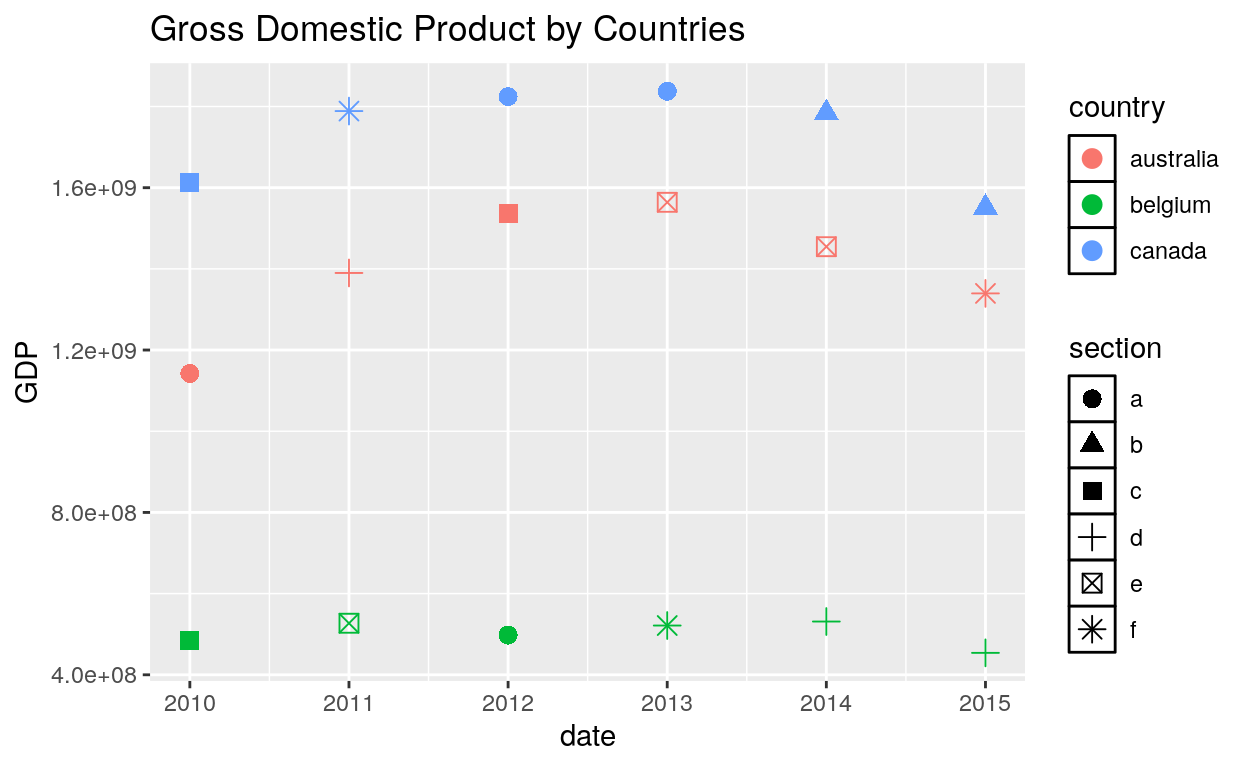###### Text and Title
``````ggplot(dataGraph, aes(date, GDP)) +
geom_point(aes(colour = country, shape = section), size = 3) +
labs(title = "Gross Domestic Product by Countries") +
theme(legend.text = element_text(size = 8, colour = "red"),
legend.title = element_text(face = "bold"))
``````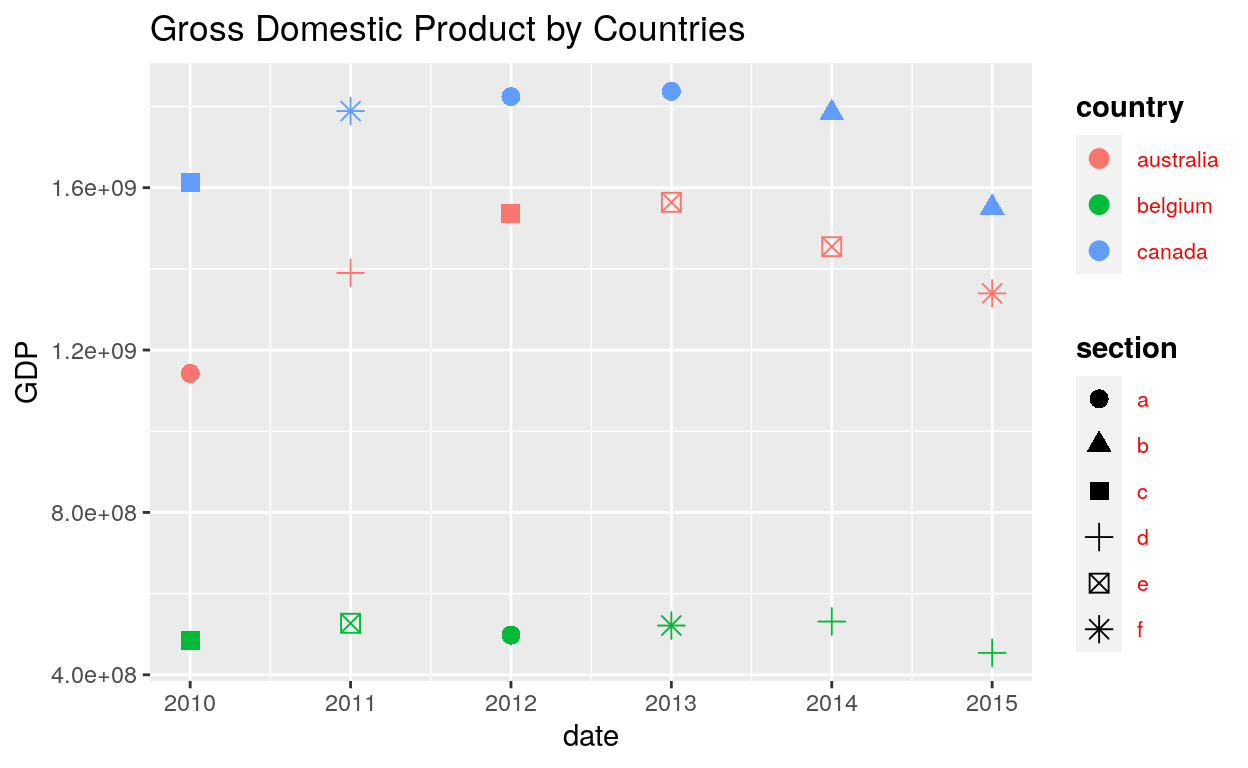#### Axis

###### Line
``````ggplot(dataGraph, aes(date, GDP)) +
geom_point(aes(colour = country, shape = section), size = 3) +
labs(title = "Gross Domestic Product by Countries") +
theme(axis.line = element_line(size = 3, colour = "grey80"))
``````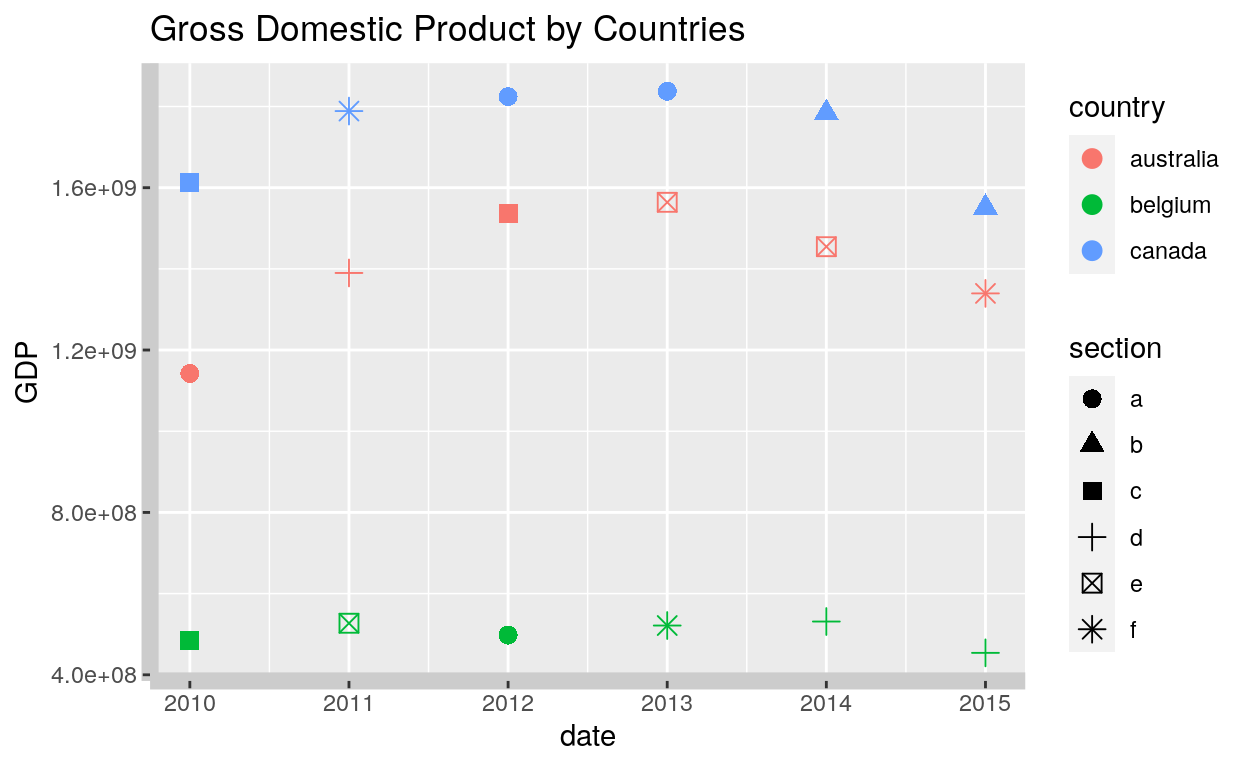###### Text
``````ggplot(dataGraph, aes(date, GDP)) +
geom_point(aes(colour = country, shape = section), size = 3) +
labs(title = "Gross Domestic Product by Countries") +
theme(axis.line = element_line(size = 3, colour = "grey80"),
axis.text = element_text(colour = "blue"),
axis.text.x = element_text(margin = margin(t = .8, unit = "cm"), angle = 90))
``````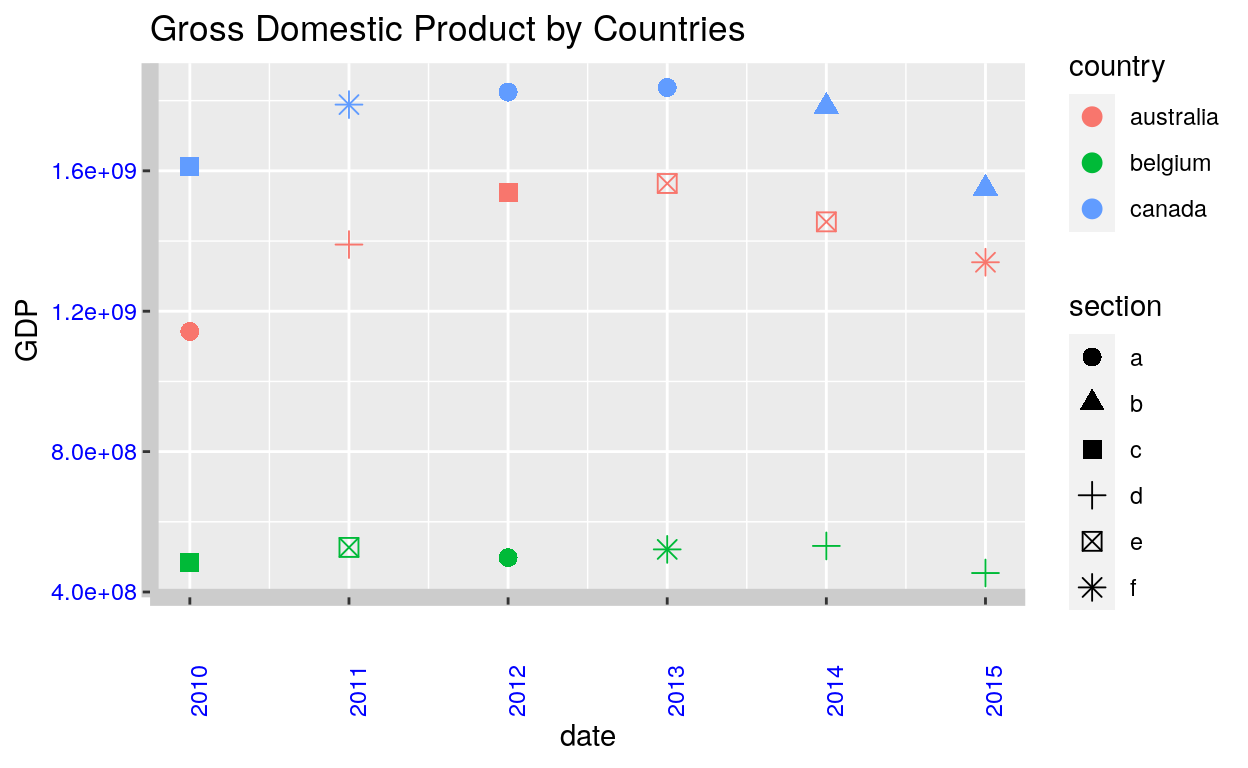###### Ticks
``````ggplot(dataGraph, aes(date, GDP)) +
geom_point(aes(colour = country, shape = section), size = 3) +
labs(title = "Gross Domestic Product by Countries") +
theme(axis.line = element_line(size = 3, colour = "grey80"),
axis.text = element_text(colour = "blue"),
axis.text.x = element_text(margin = margin(t = .8, unit = "cm"), angle = 90),
axis.ticks = element_line(size = 2),
axis.ticks.length.y = unit(.25, "cm"),
axis.ticks.length.x = unit(.3, "cm"),
axis.title.y = element_text(size = 15, angle = 90))
``````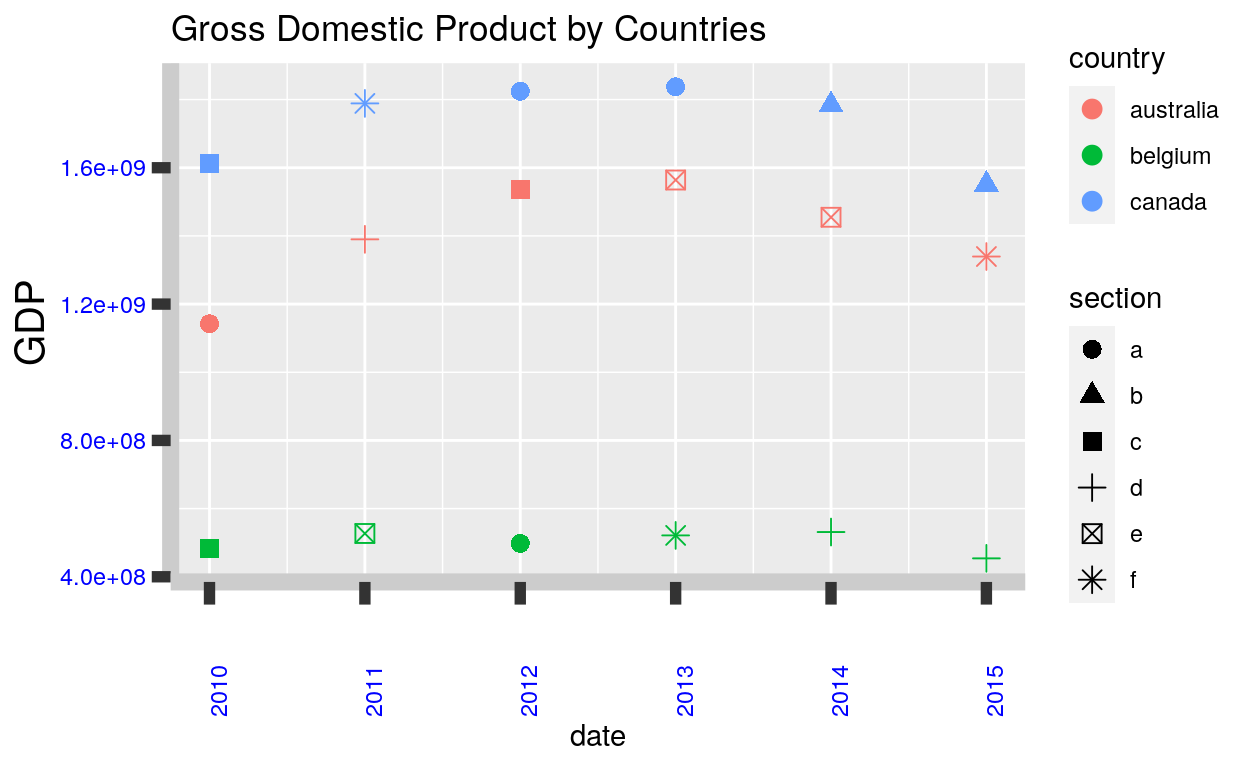#### Panel

###### Background
``````ggplot(dataGraph, aes(date, GDP)) +
geom_point(aes(colour = country, shape = section), size = 3) +
labs(title = "Gross Domestic Product by Countries") +
theme(plot.title = element_text(size = 20),
panel.background = element_rect(fill = "lavender", colour = "purple"))
``````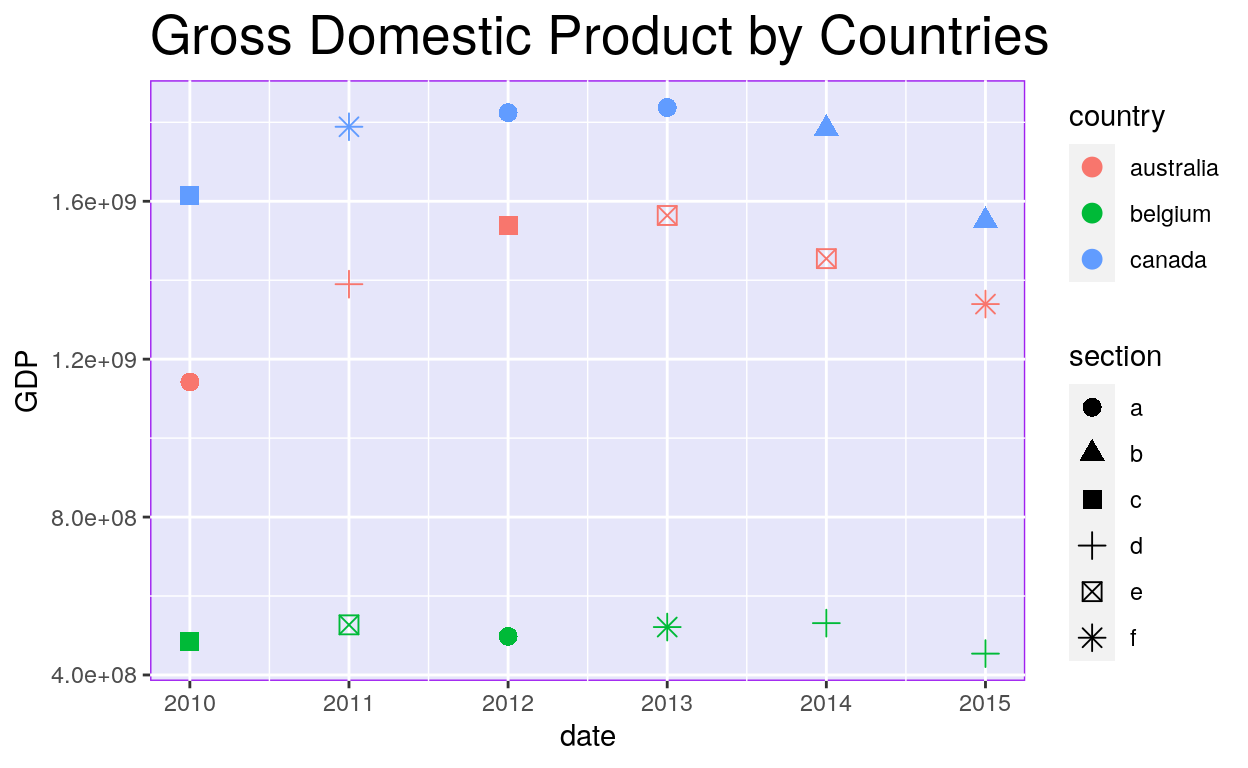###### Border
• Default
``````ggplot(dataGraph, aes(date, GDP)) +
geom_point(aes(colour = country, shape = section), size = 3) +
labs(title = "Gross Domestic Product by Countries") +
theme(plot.title = element_text(size = 20),
panel.border = element_rect(fill = NA))
``````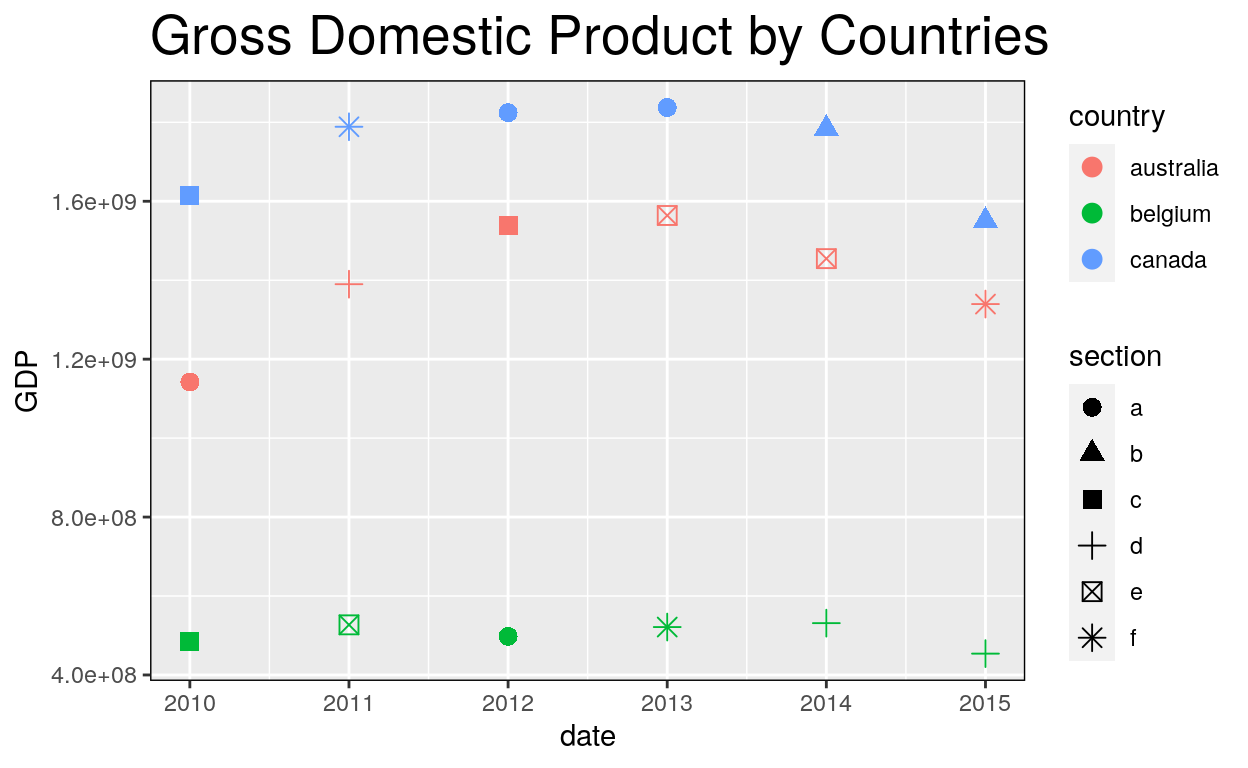• Linetype option
``````ggplot(dataGraph, aes(date, GDP)) +
geom_point(aes(colour = country, shape = section), size = 3) +
labs(title = "Gross Domestic Product by Countries") +
theme(plot.title = element_text(size = 20),
panel.border = element_rect(linetype = "dashed", fill = NA))
``````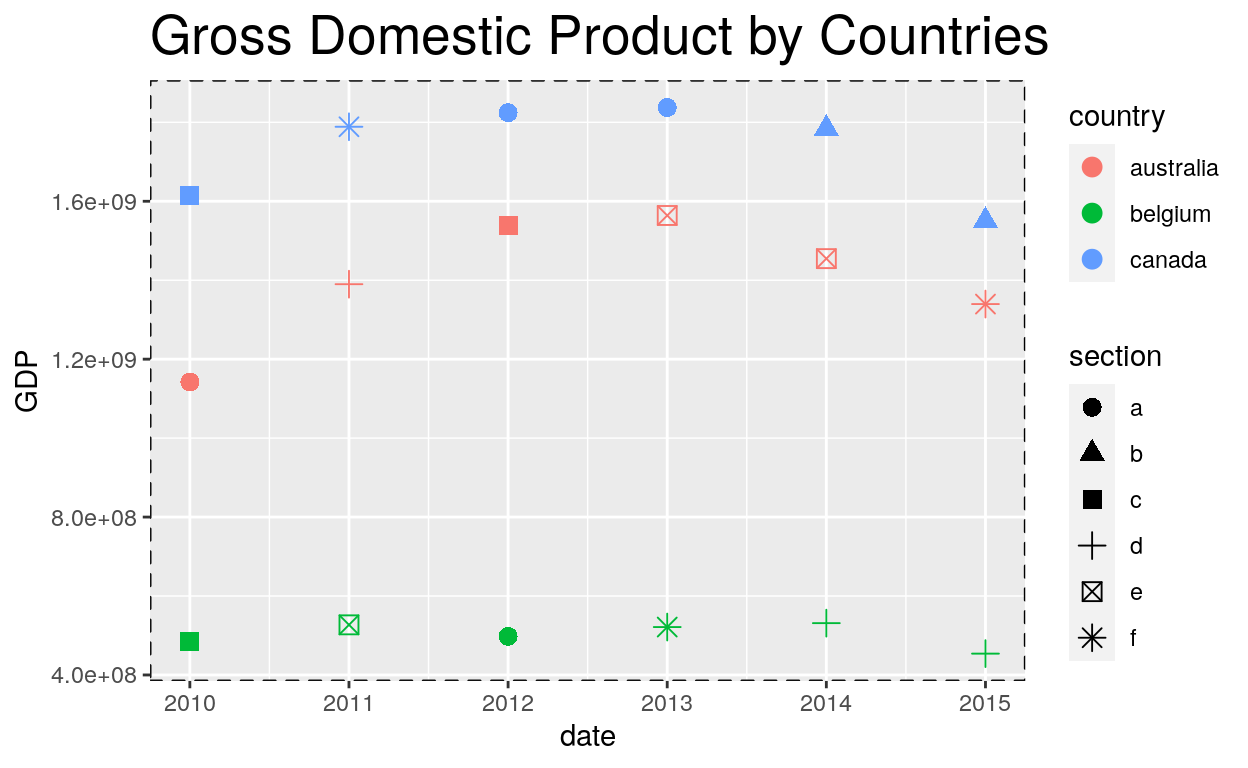###### Grid major and minor
``````ggplot(dataGraph, aes(date, GDP)) +
geom_point(aes(colour = country, shape = section), size = 3) +
labs(title = "Gross Domestic Product by Countries") +
theme(plot.title = element_text(size = 20),
panel.grid.major = element_line(colour = "magenta"))
``````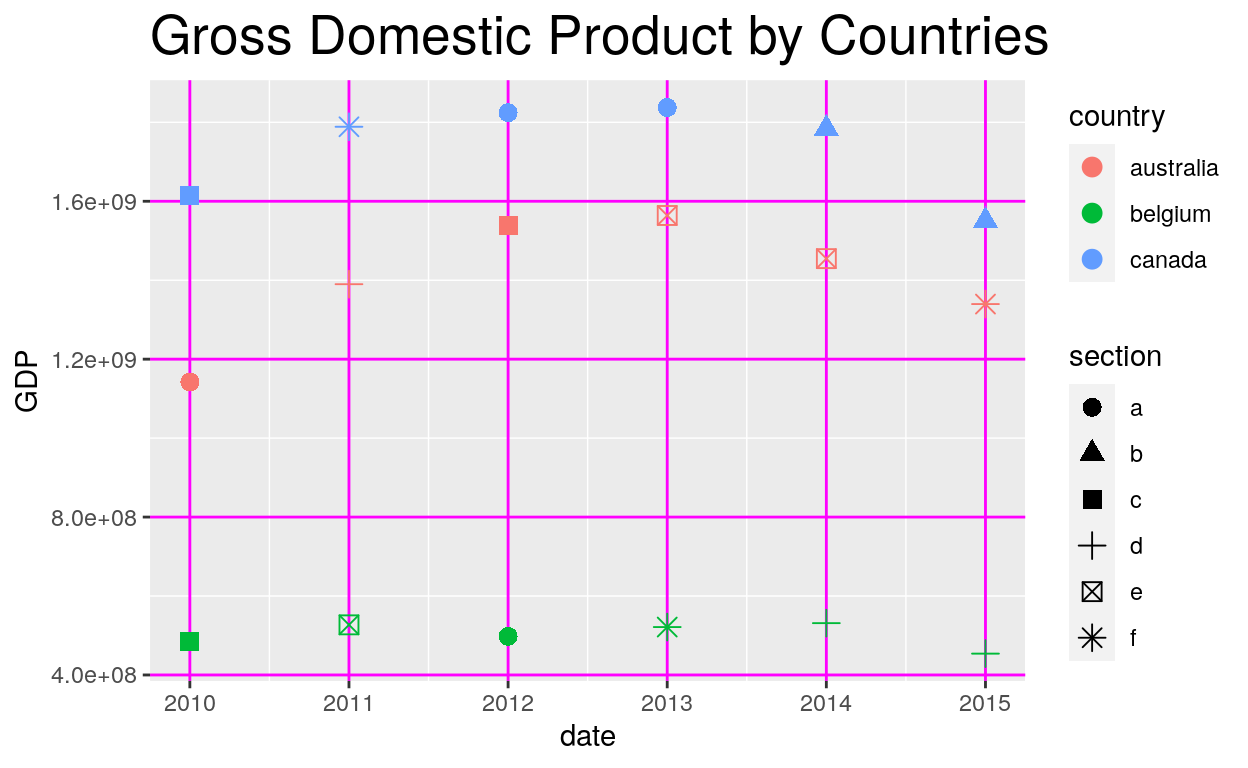``````ggplot(dataGraph, aes(date, GDP)) +
geom_point(aes(colour = country, shape = section), size = 3) +
labs(title = "Gross Domestic Product by Countries") +
theme(panel.grid.major.y = element_blank(),
panel.grid.minor.y = element_blank())
``````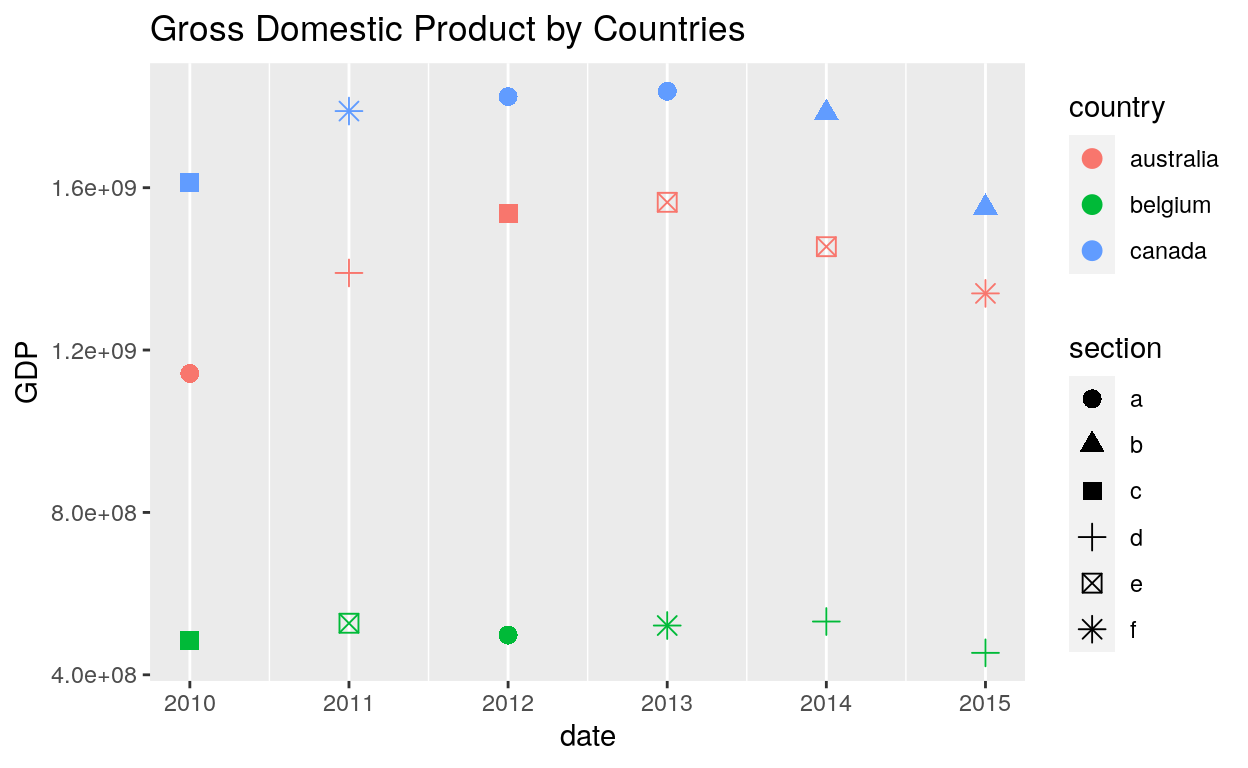``````ggplot(dataGraph, aes(date, GDP)) +
geom_point(aes(colour = country, shape = section), size = 3) +
labs(title = "Gross Domestic Product by Countries") +
theme(panel.grid.major.x = element_blank(),
panel.grid.minor.x = element_blank())
``````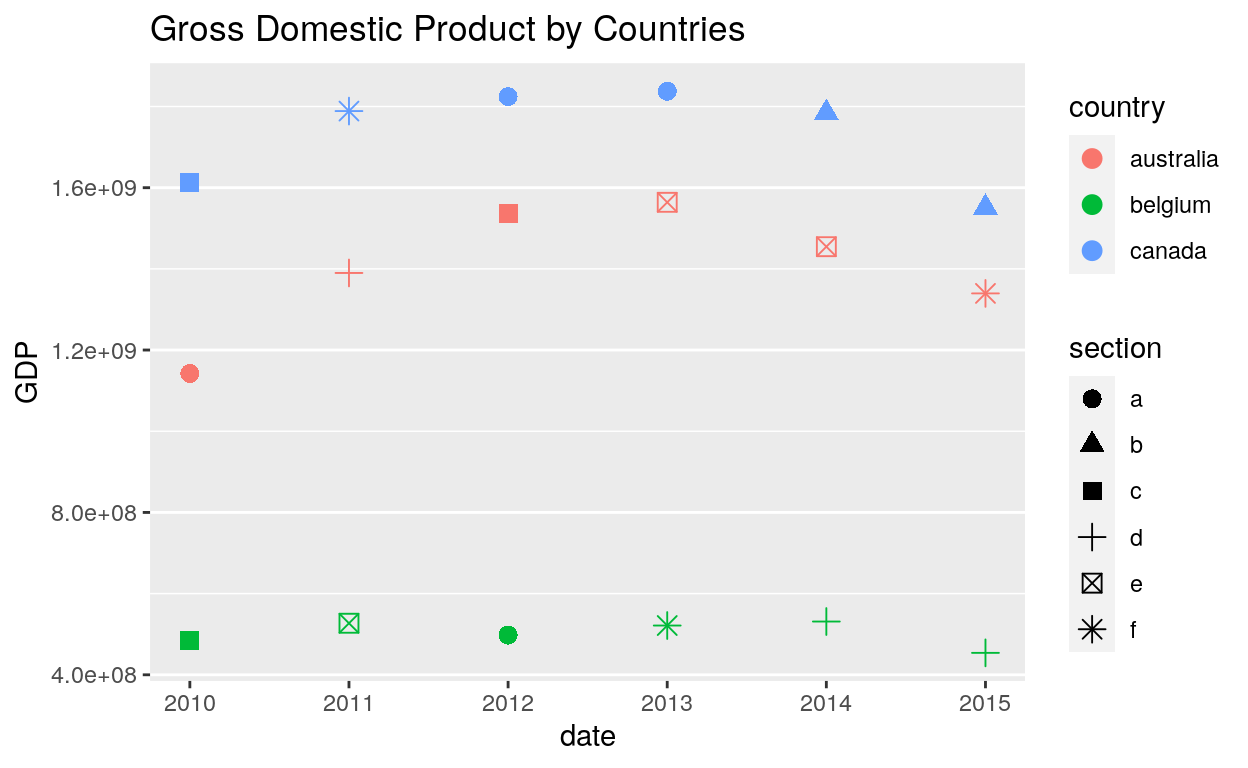### Strip

#### Background

``````ggplot(dataGraph, aes(sample, GDP)) +
geom_point() +
facet_wrap(~ country) +
theme(strip.background = element_rect(colour = "black", fill = "white"))
``````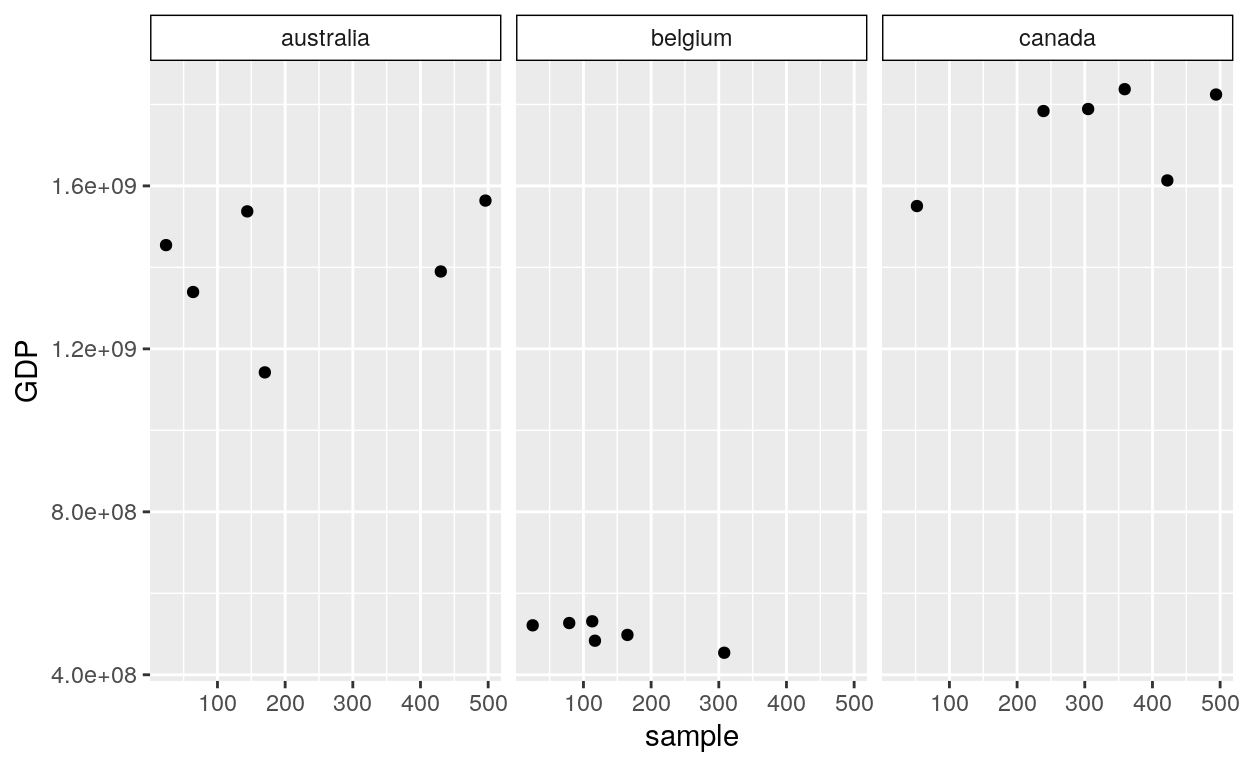#### Text

``````ggplot(dataGraph, aes(sample, GDP)) +
geom_point() +
facet_wrap(~ country) +
theme(strip.text.x = element_text(colour = "white", face = "bold"))
````````````ggplot(dataGraph, aes(sample, GDP)) +
geom_point() +
facet_wrap(~ country) +
theme(panel.spacing = unit(2, "lines"))
``````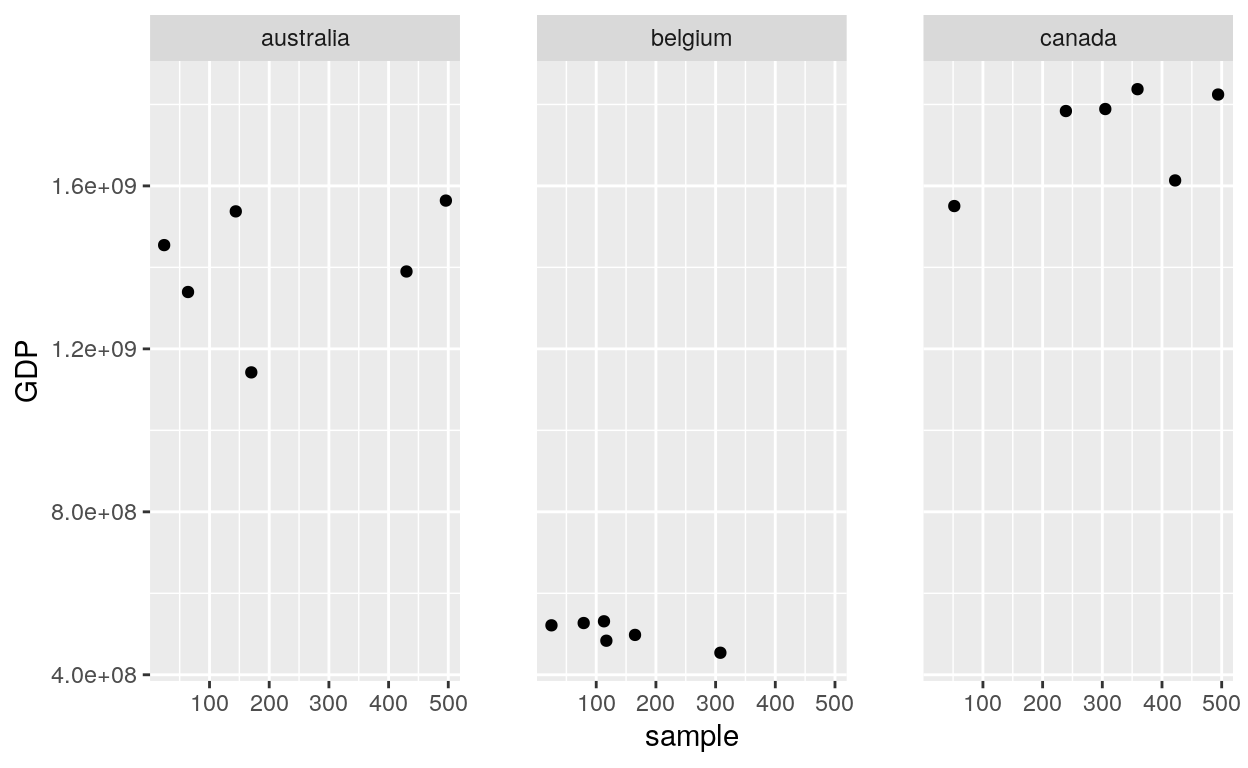## Facetting

### Facet wrap

``````ggplot(dataGraph, aes(sample, GDP)) +
geom_point() +
facet_wrap(~ country)
``````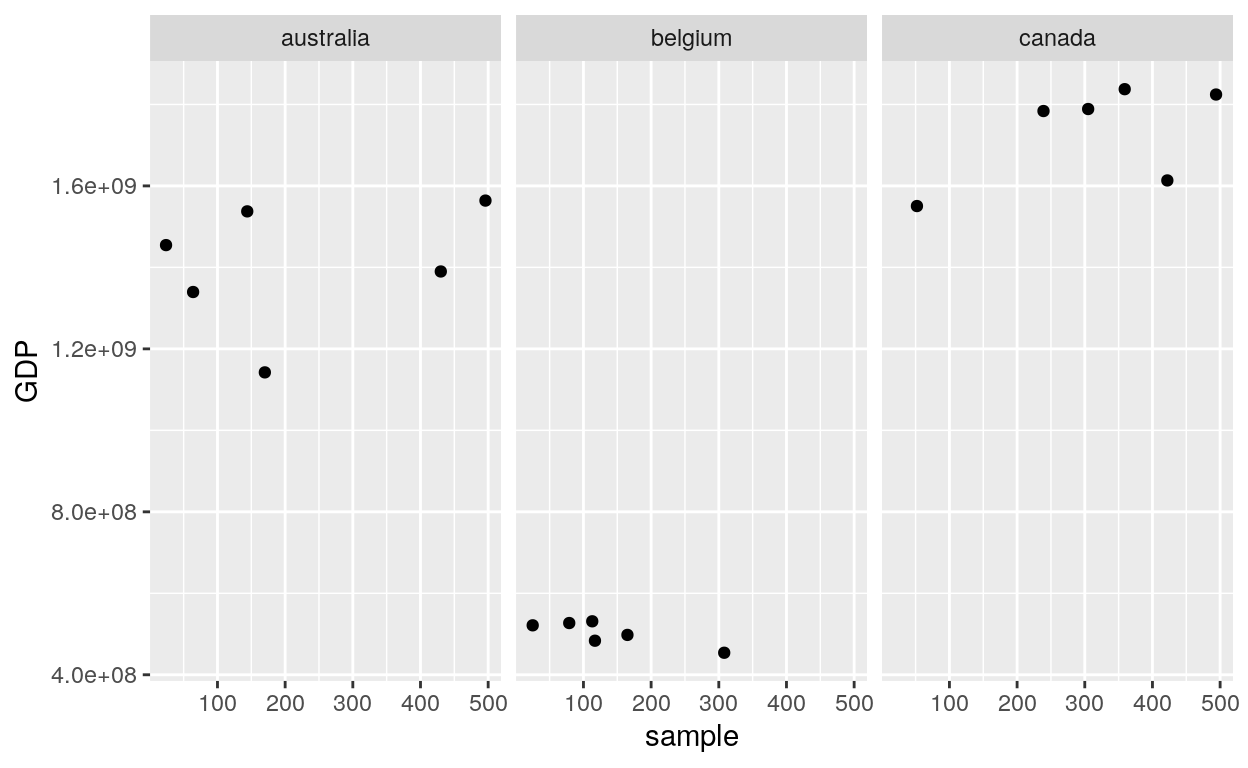### Facet Grid

#### Rows

``````ggplot(dataGraph, aes(GDP, sample)) +
geom_point() +
facet_grid(rows = vars(country))
``````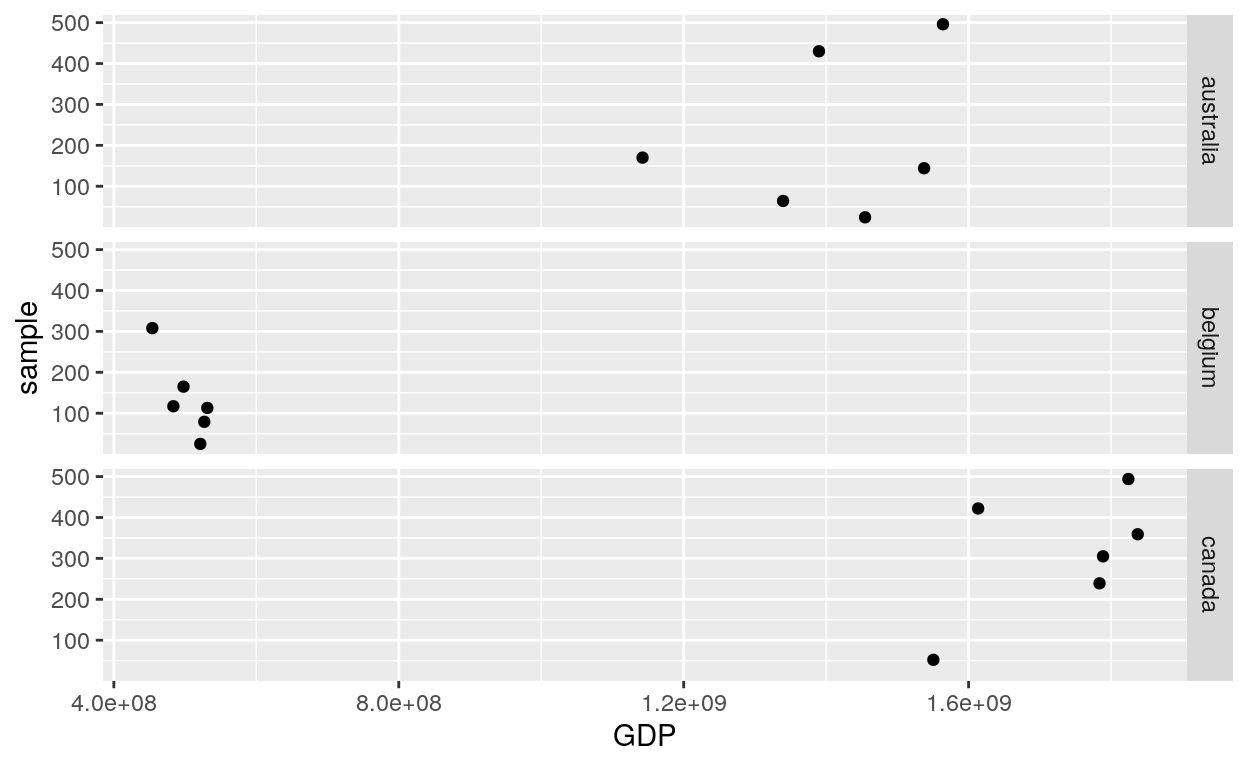#### Cols

``````ggplot(dataGraph, aes(GDP, sample)) +
geom_point() +
facet_grid(cols = vars(country))
``````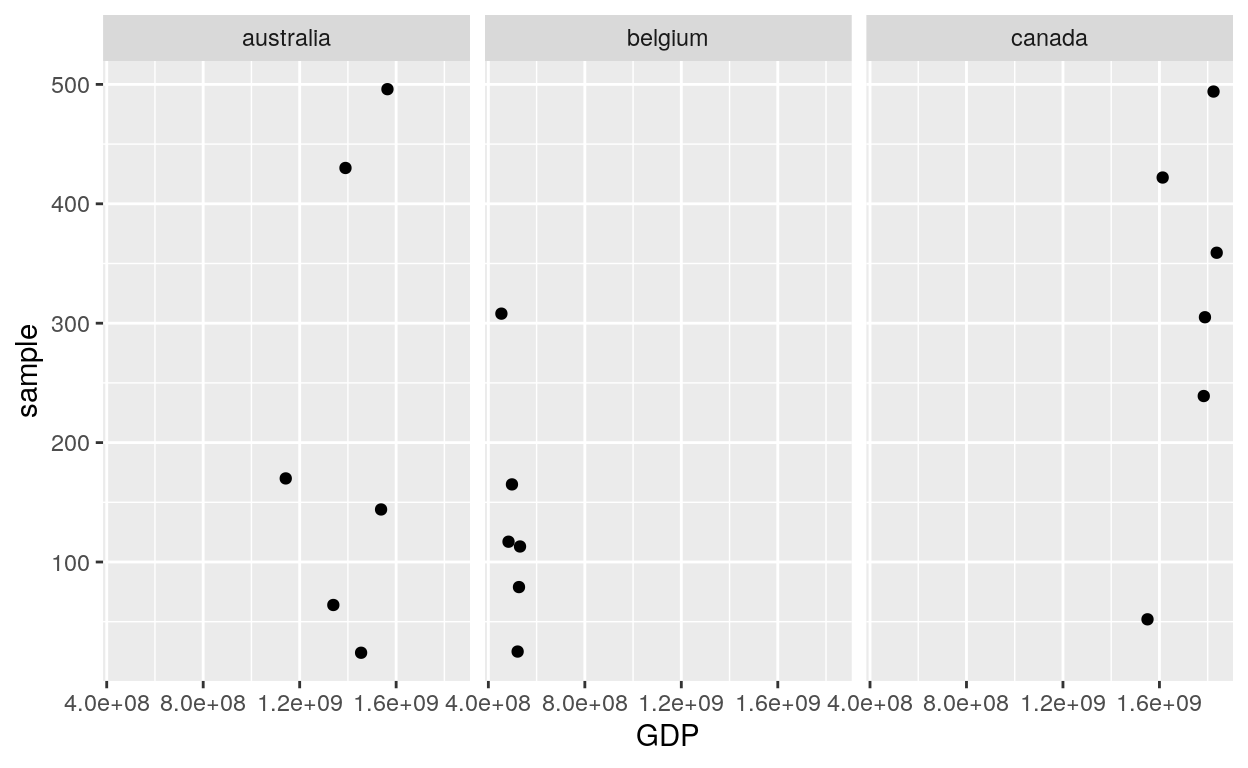#### Vars

``````ggplot(dataGraph, aes(GDP, sample)) +
geom_point() +
facet_grid(vars(country), vars(section)) +
theme(axis.text.x = element_text(angle = 90))
``````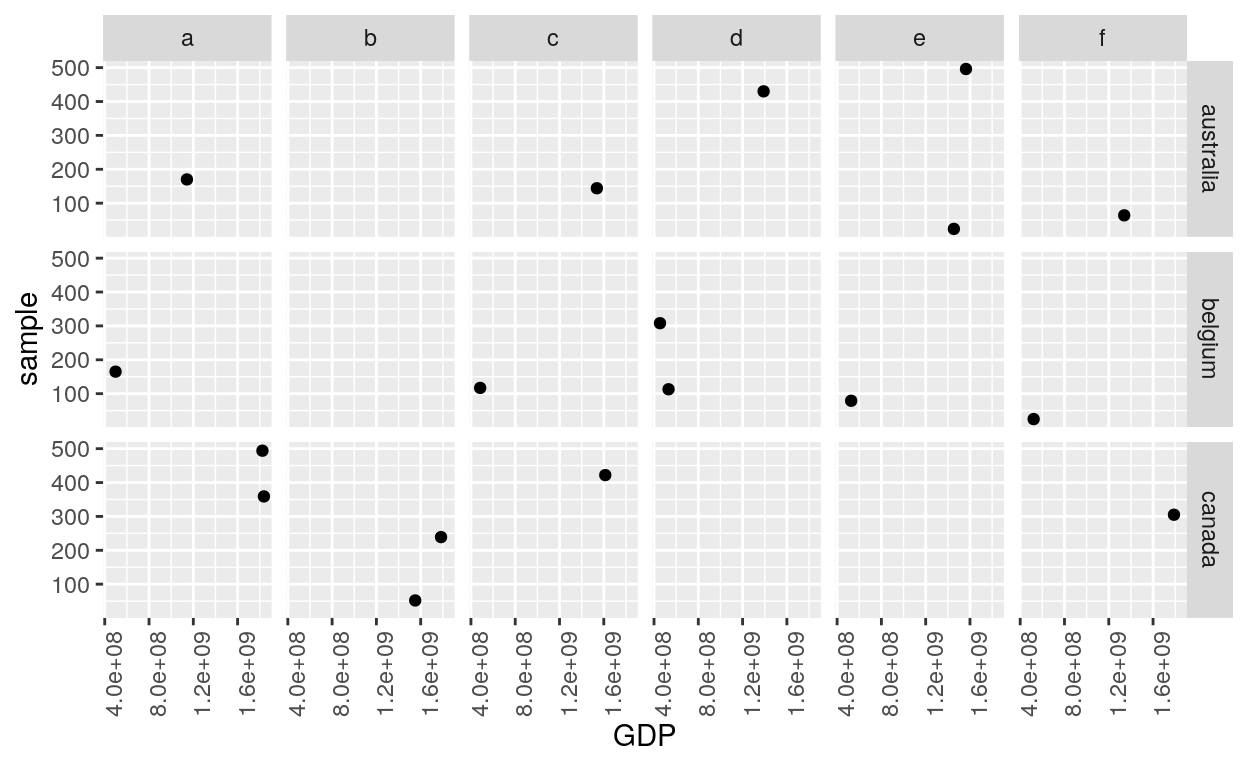``````# You can also choose whether the scales should be constant across all panels (the default),
# or whether they should be allowed to vary
ggplot(dataGraph, aes(GDP, sample, colour = country)) +
geom_point() +
facet_grid(. ~ country, scales = "free") +
theme(axis.text.x = element_text(angle = 90))
``````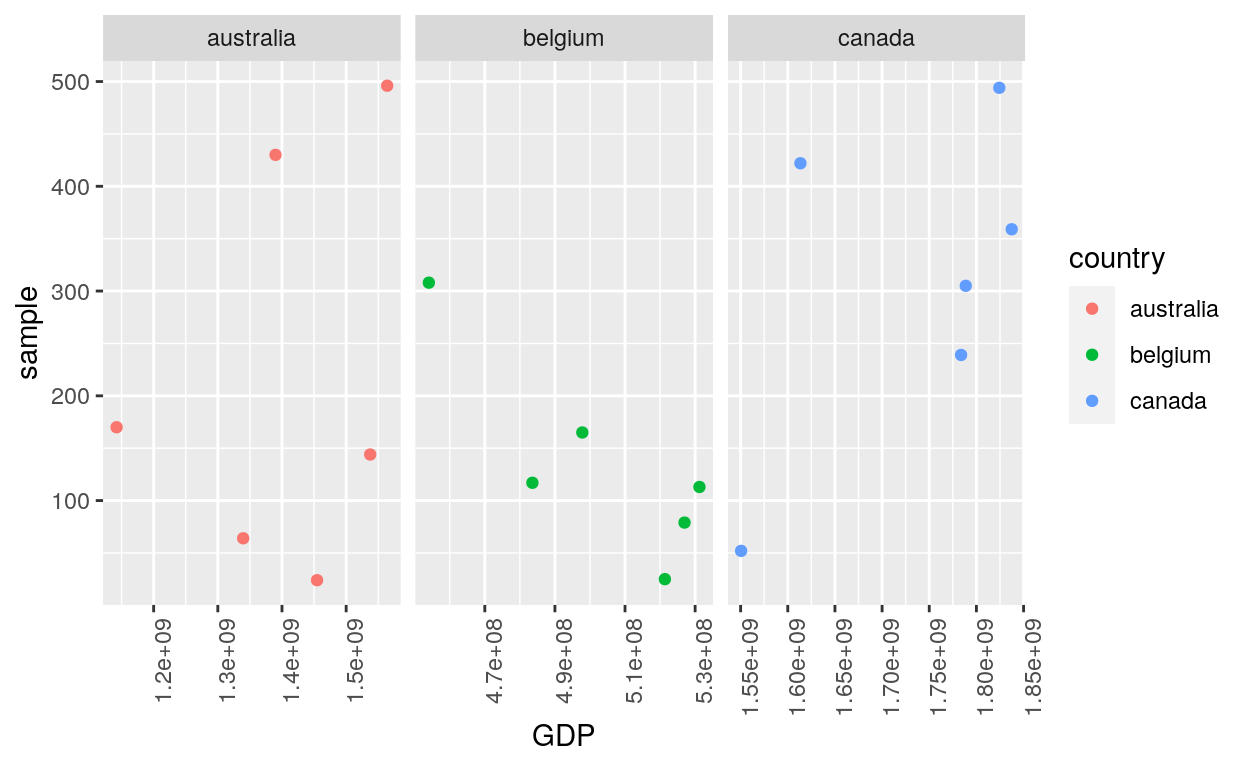## Guides

### Fill

``````ggplot(dataGraph, aes(date, GDP, fill=country)) +
geom_bar(stat="identity", position = "dodge") +
guides(fill = guide_legend(title = "LEFT",
title.position = "left",
title.theme = element_text(size = 15, face = "italic", colour = "red",angle = 0)))
``````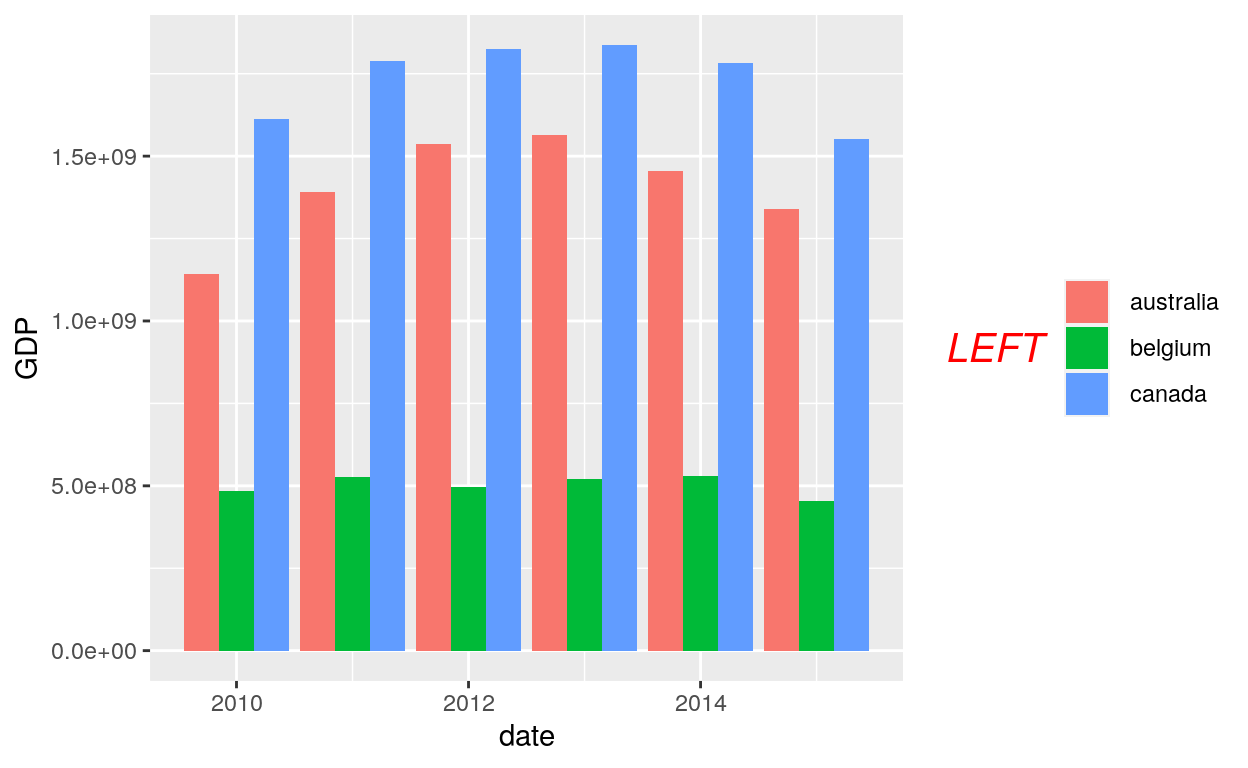### Colour

``````ggplot(dataGraph, aes(date, GDP, colour=country)) +
geom_jitter(alpha = 1/3, width = 0.01, height = 0.01) +
guides(colour = guide_legend(override.aes = list(alpha = 1)))
``````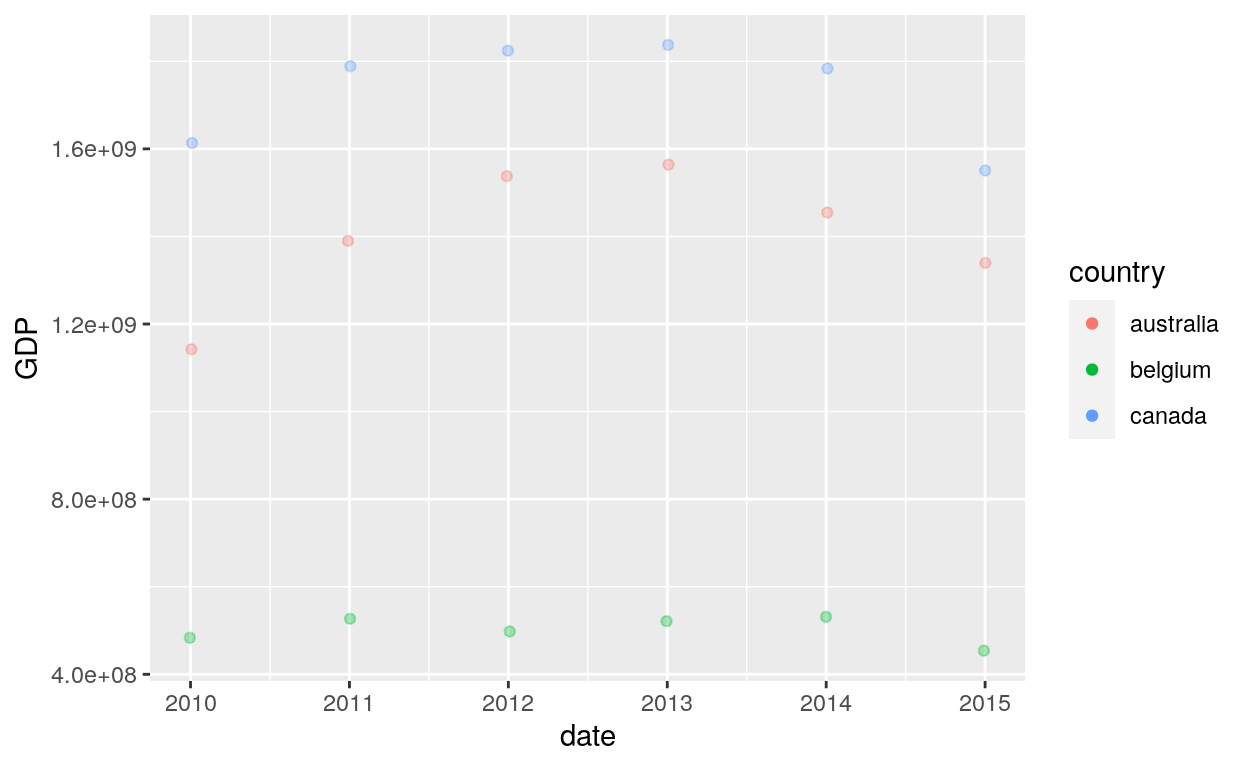## Scales

### Sequential, diverging and qualitative

The brewer scales provides sequential, diverging and qualitative colour schemes from ColorBrewer. These are particularly well suited to display discrete values on a map. See the colorbrewer2 website for more information.

#### Default

``````ggplot(iris, aes(Sepal.Length, Sepal.Width)) +
geom_point(aes(colour = Species))
``````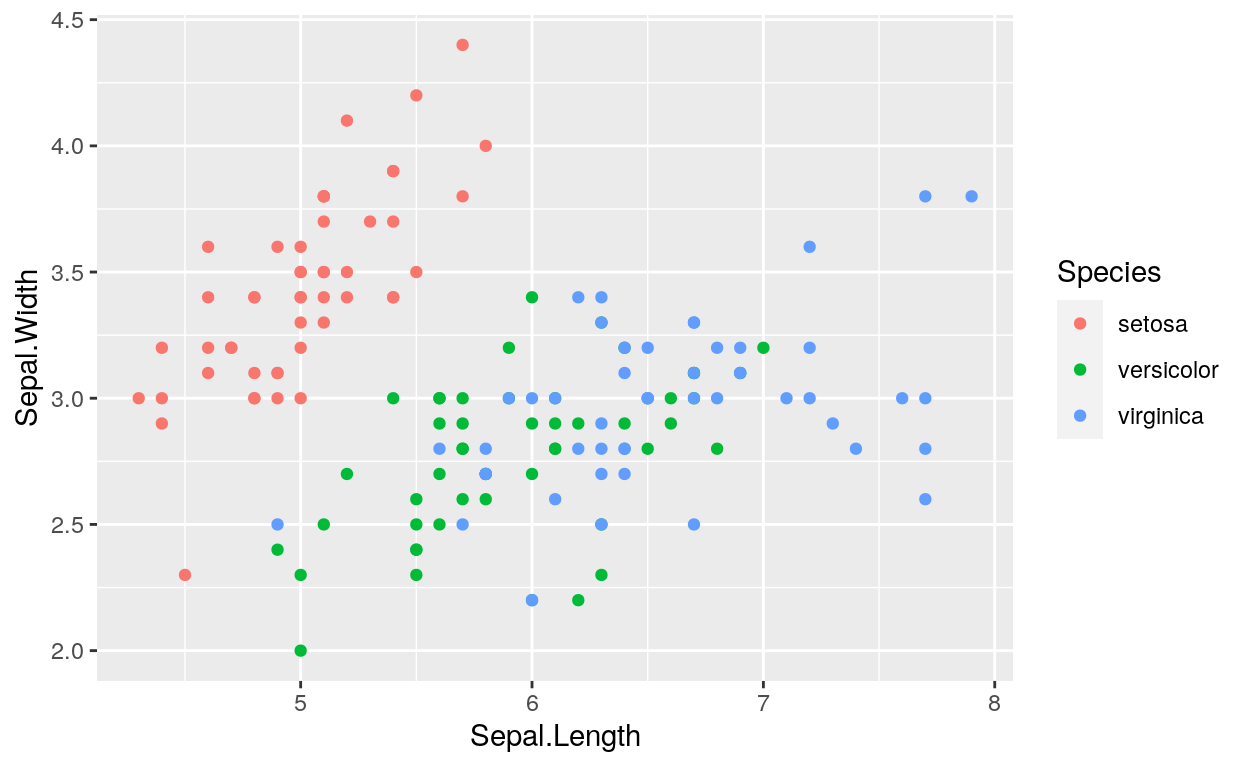#### Scale colour brewer

``````ggplot(iris, aes(Sepal.Length, Sepal.Width)) +
geom_point(aes(colour = Species)) +
scale_colour_brewer("Type of\nSpecies")
``````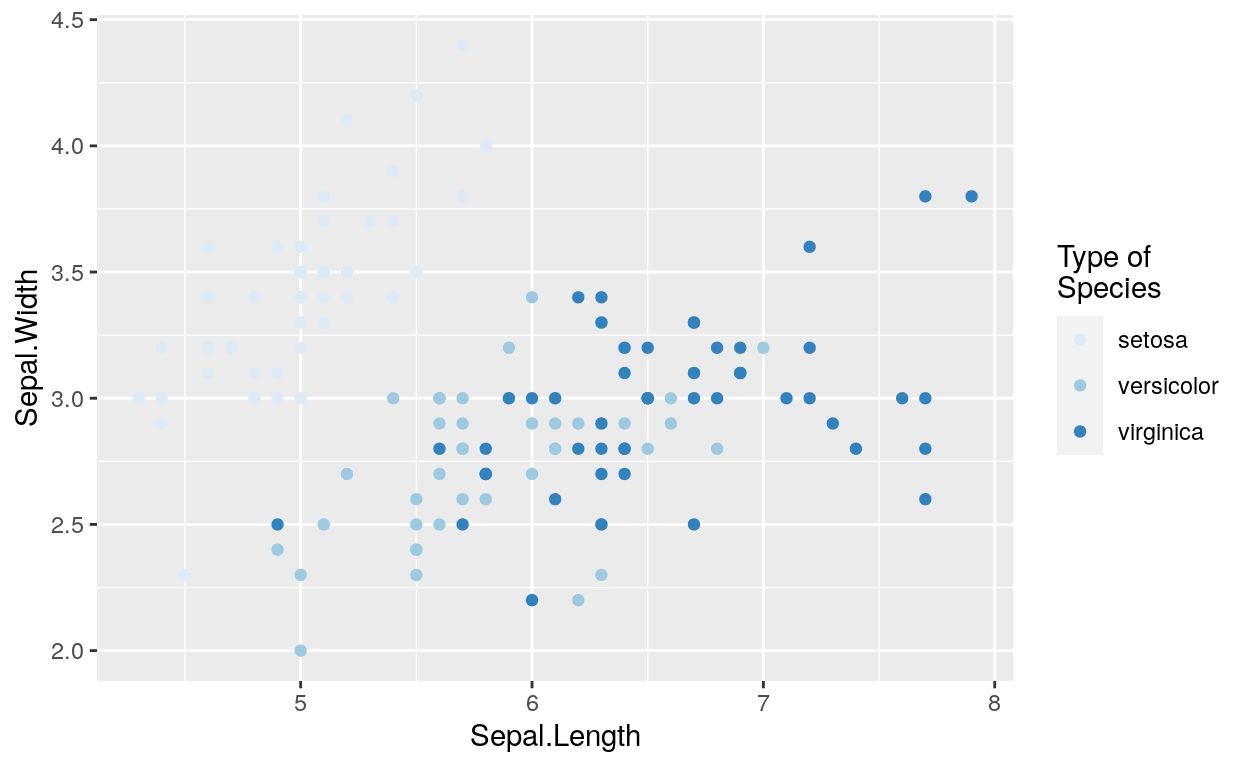``````ggplot(iris, aes(Sepal.Length, Sepal.Width)) +
geom_point(aes(colour = Species)) +
scale_colour_brewer(palette = "Greens")
``````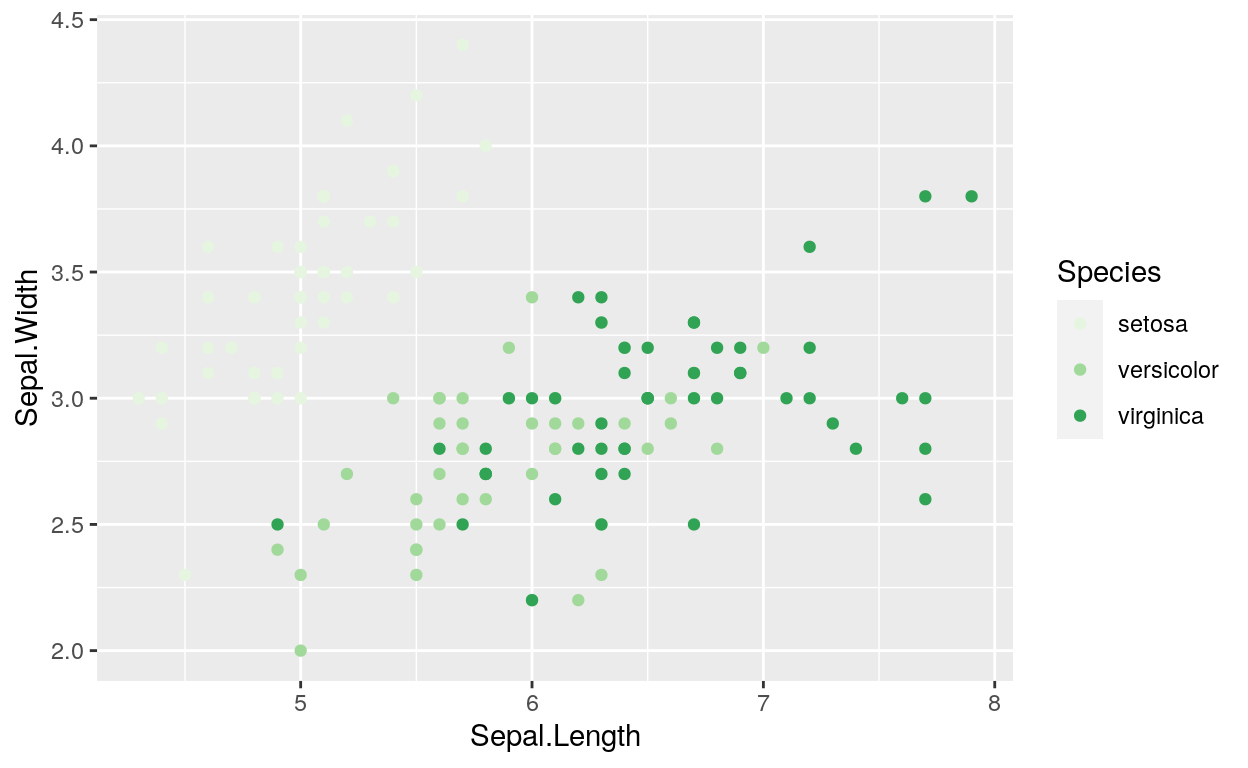``````ggplot(iris, aes(Sepal.Length, Sepal.Width)) +
geom_point(aes(colour = Species)) +
scale_colour_brewer(palette = "Set1")
``````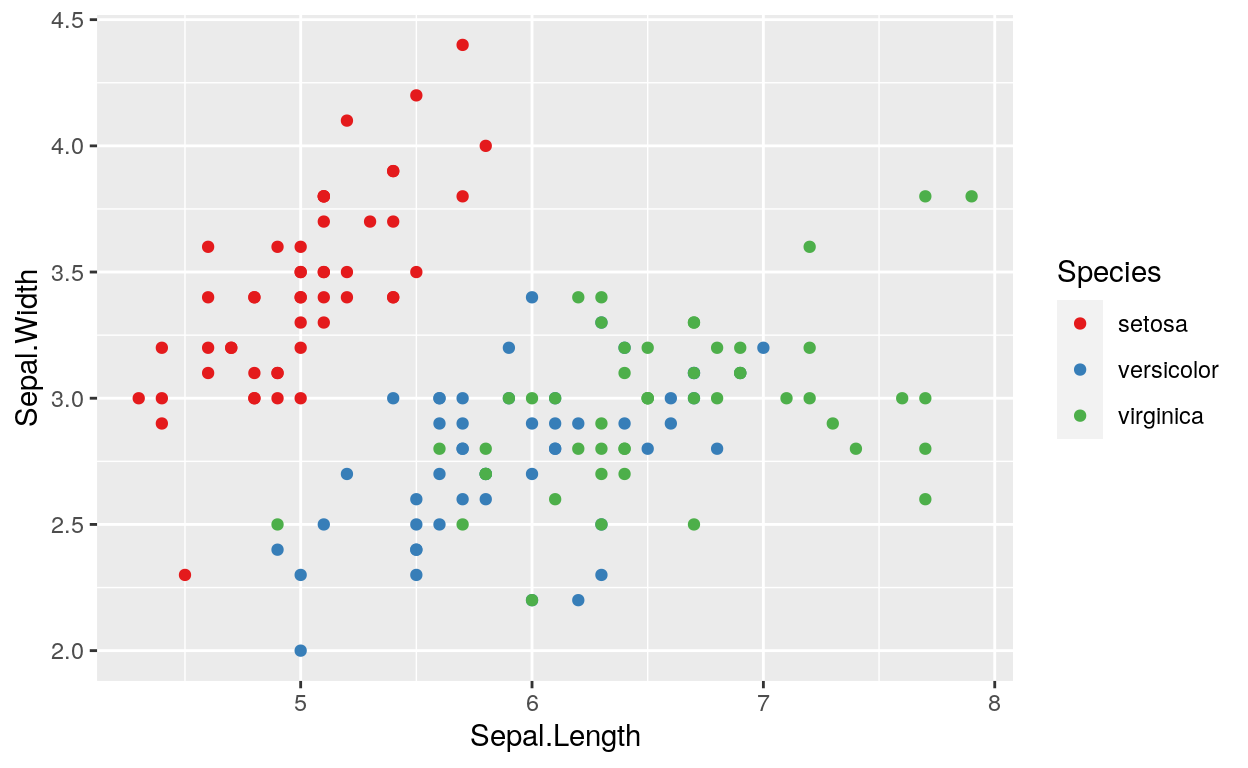#### Scale fill brewer

``````# scale_fill_brewer works just the same as
# scale_colour_brewer but for the fill option
ggplot(dataGraph, aes(date, GDP, fill = country)) +
geom_bar(stat = "identity", position = "dodge") +
scale_fill_brewer()
``````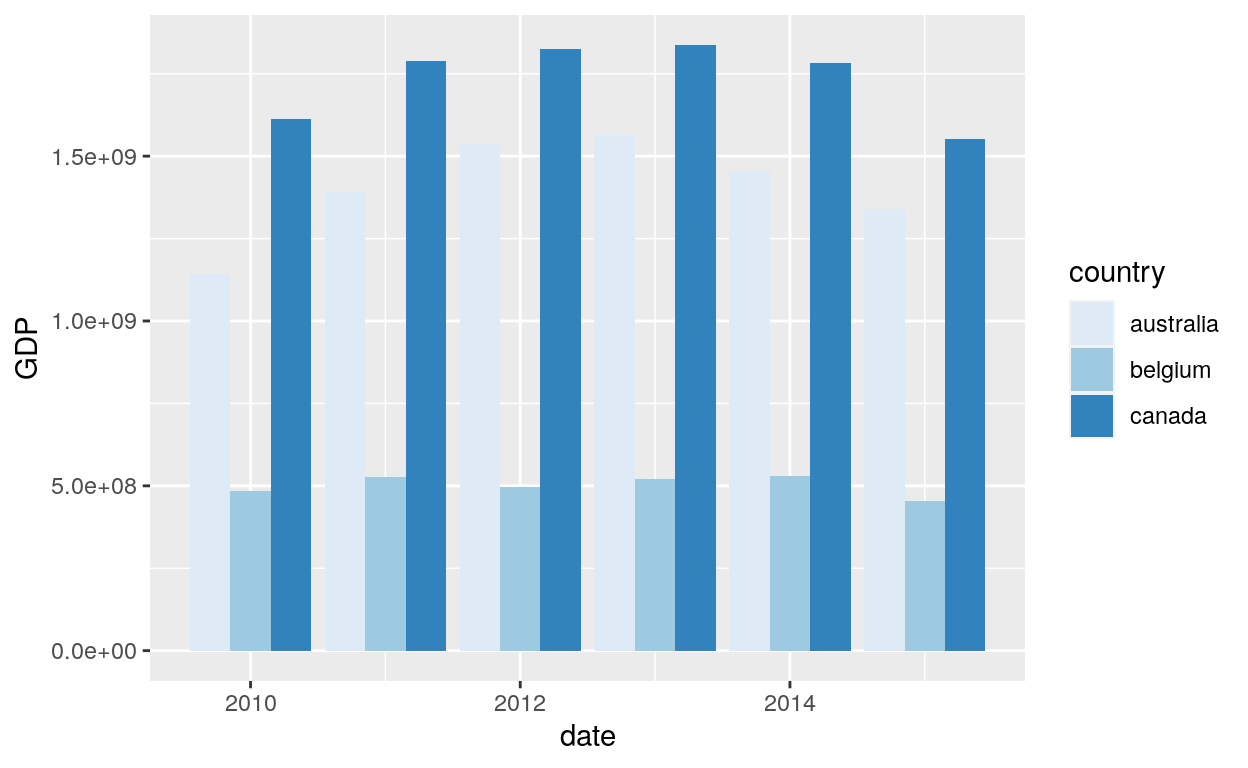``````ggplot(dataGraph, aes(date, GDP, fill = country)) +
geom_bar(stat = "identity", position = "dodge") +
scale_fill_brewer(direction = -1)
``````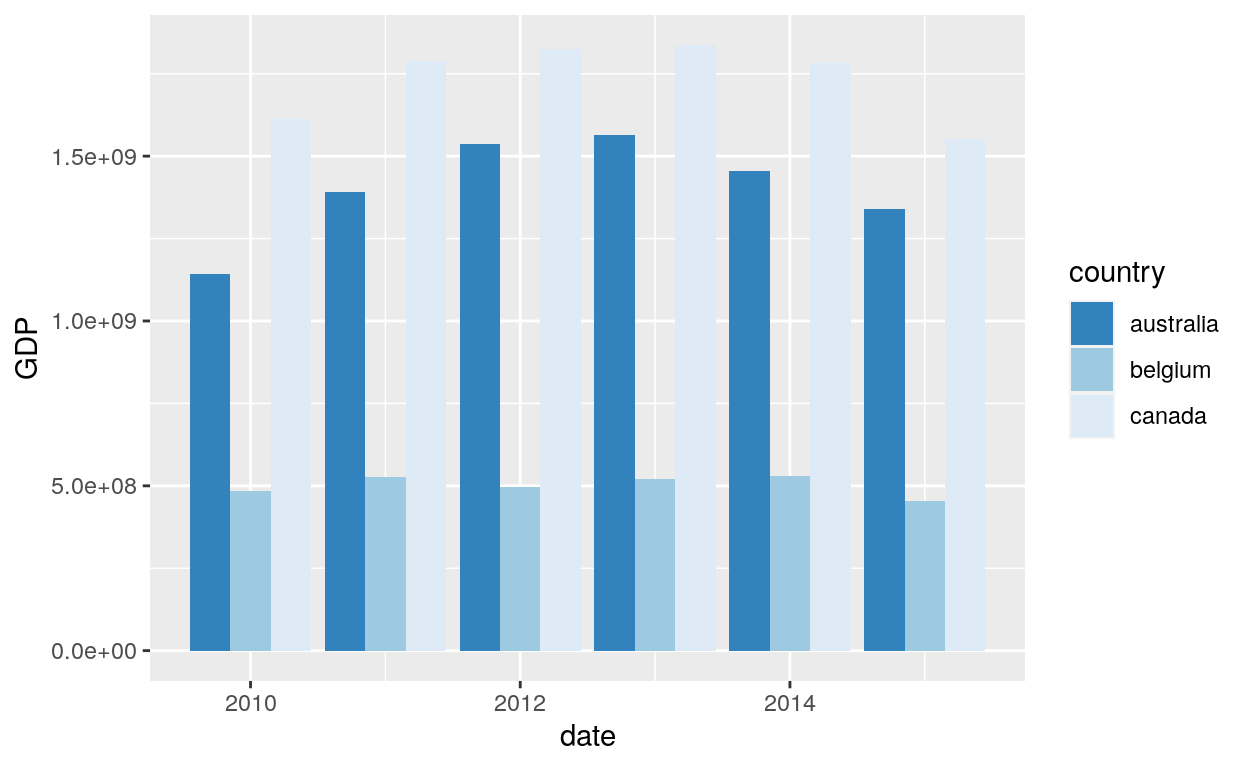### Viridis colour

The viridis scales provide colour maps that are perceptually uniform in both colour and black-and-white. They are also designed to be perceived by viewers with common forms of colour blindness. See also https://bids.github.io/colormap/.

#### Ordered factors

``````# viridis is the default colour/fill scale for ordered factors
dataGraph\$section <- factor(dataGraph\$section, levels=c("a", "b", "c", "d", "e", "f"), ordered=TRUE)
``````
``````ggplot(dataGraph, aes(GDP, sample)) +
geom_point(aes(colour = section))
``````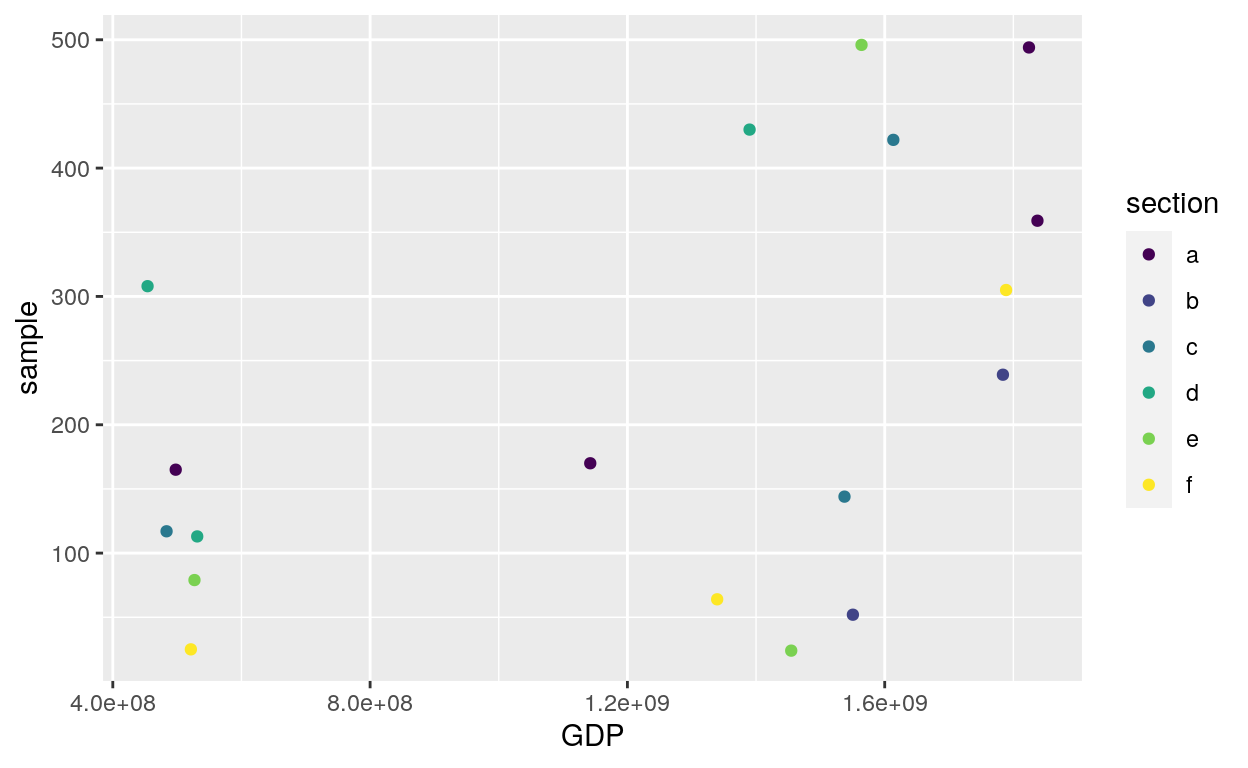#### Discrete data: Colour

##### Viridis
``````# Use viridis_d with discrete data
# Change scale label
ggplot(dataGraph, aes(GDP, sample)) +
geom_point(aes(colour = country)) +
scale_colour_viridis_d("Countries")
``````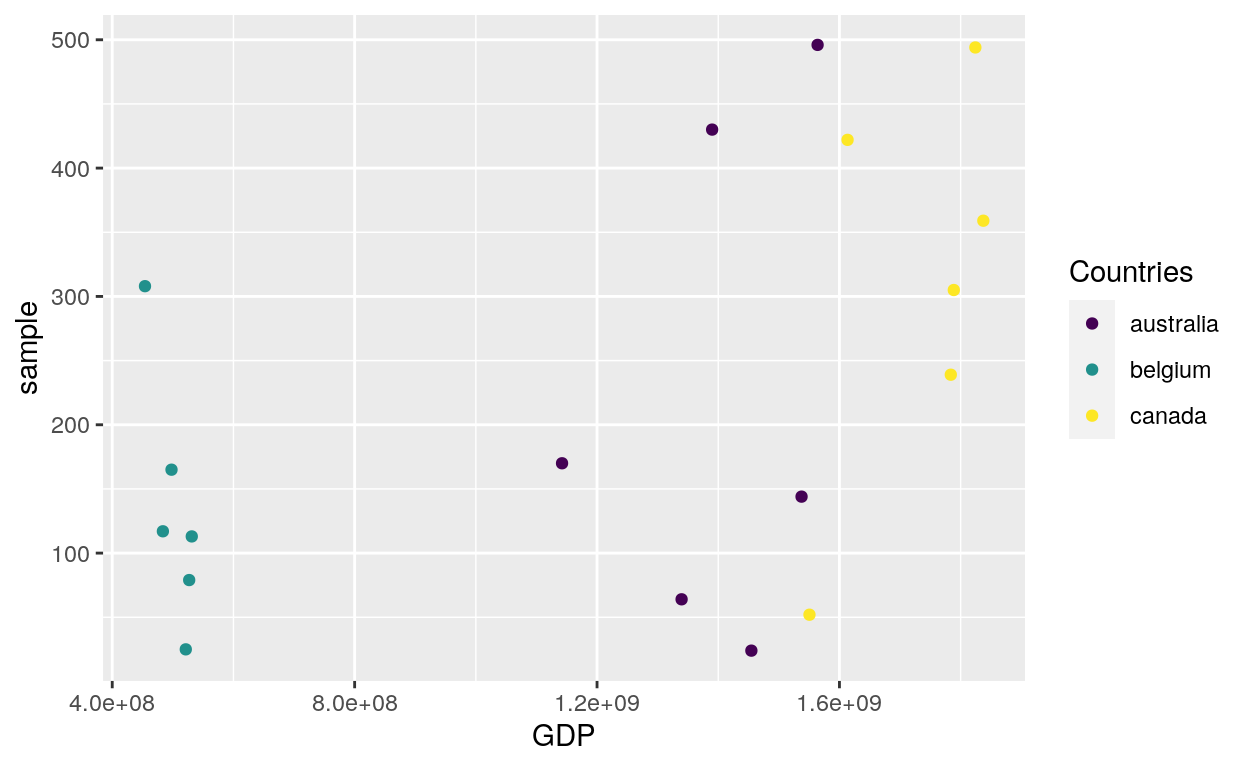##### Plasma
``````# Option plasma
ggplot(dataGraph, aes(GDP, sample)) +
geom_point(aes(colour = country)) +
scale_colour_viridis_d(option = "plasma")
``````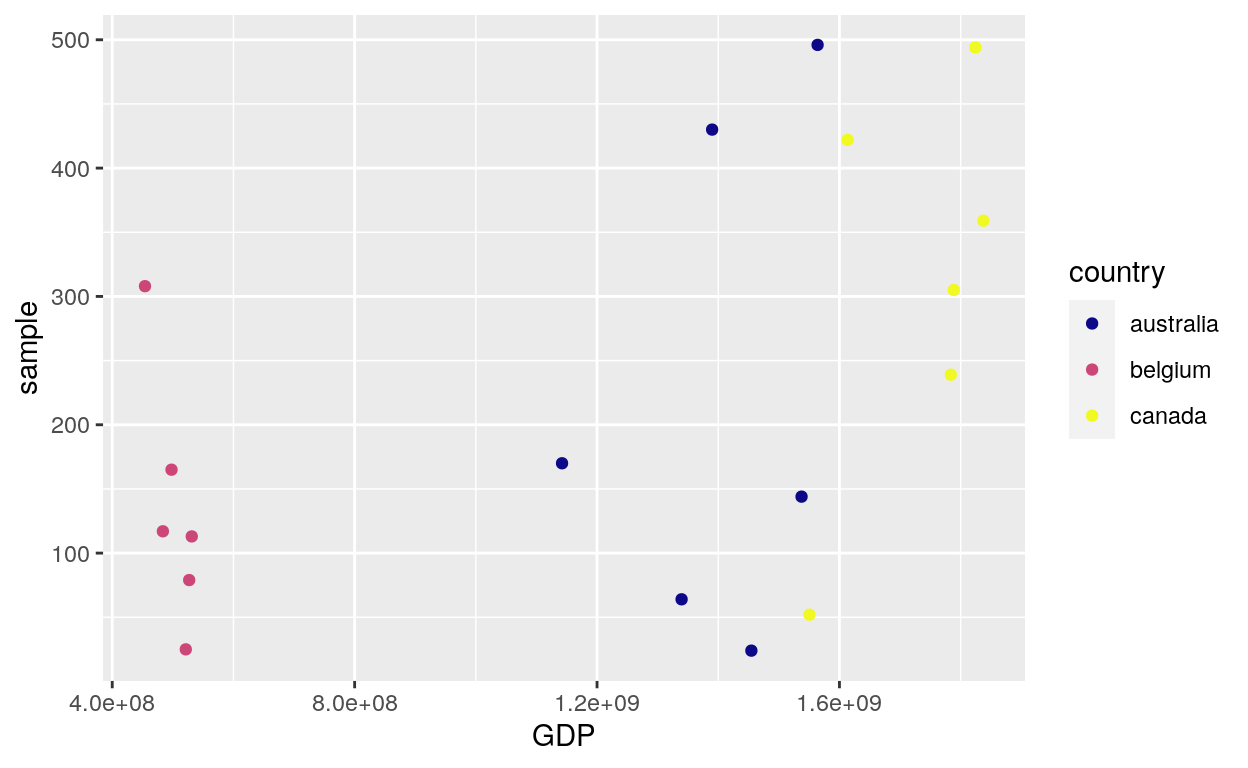##### Inferno
``````# Option inferno
ggplot(dataGraph, aes(GDP, sample)) +
geom_point(aes(colour = country)) +
scale_colour_viridis_d(option = "inferno")
``````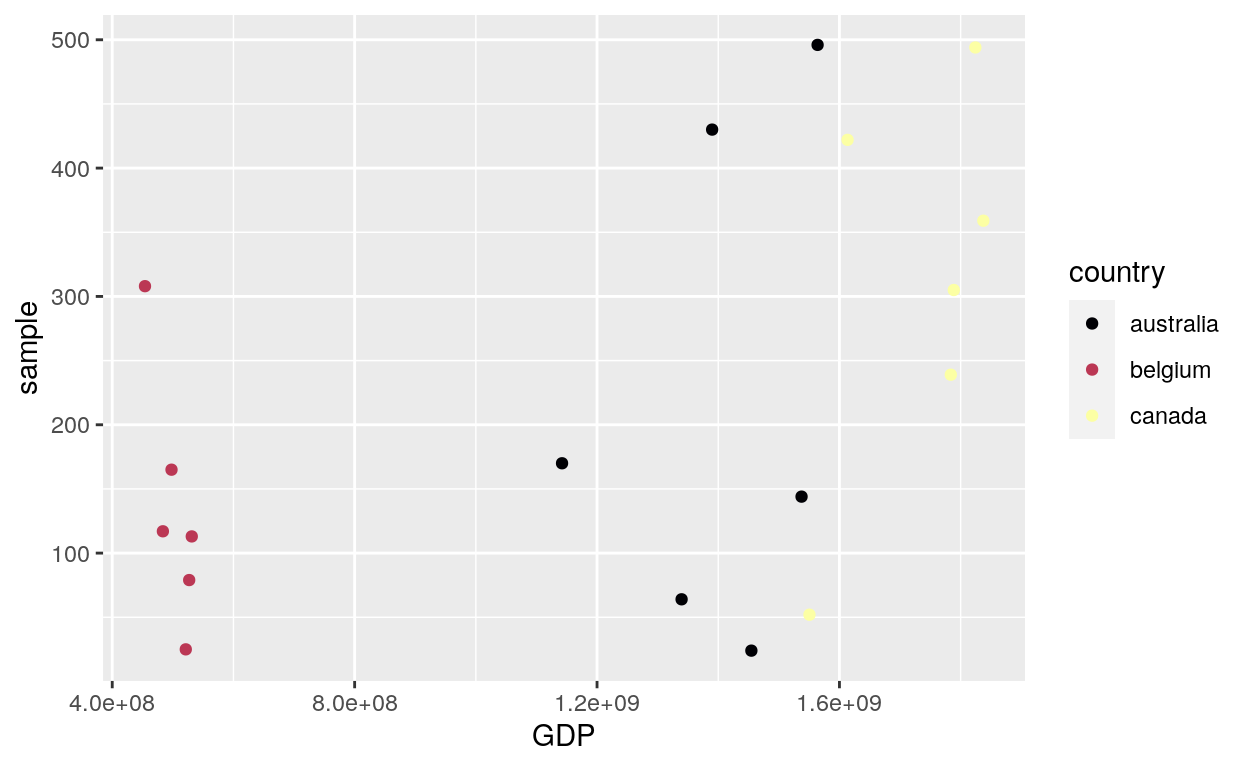#### Discrete data: Fill

##### Viridis
``````ggplot(dataGraph, aes(date, GDP, fill = country)) +
geom_bar(stat = "identity", position = "dodge") +
scale_fill_viridis_d()
``````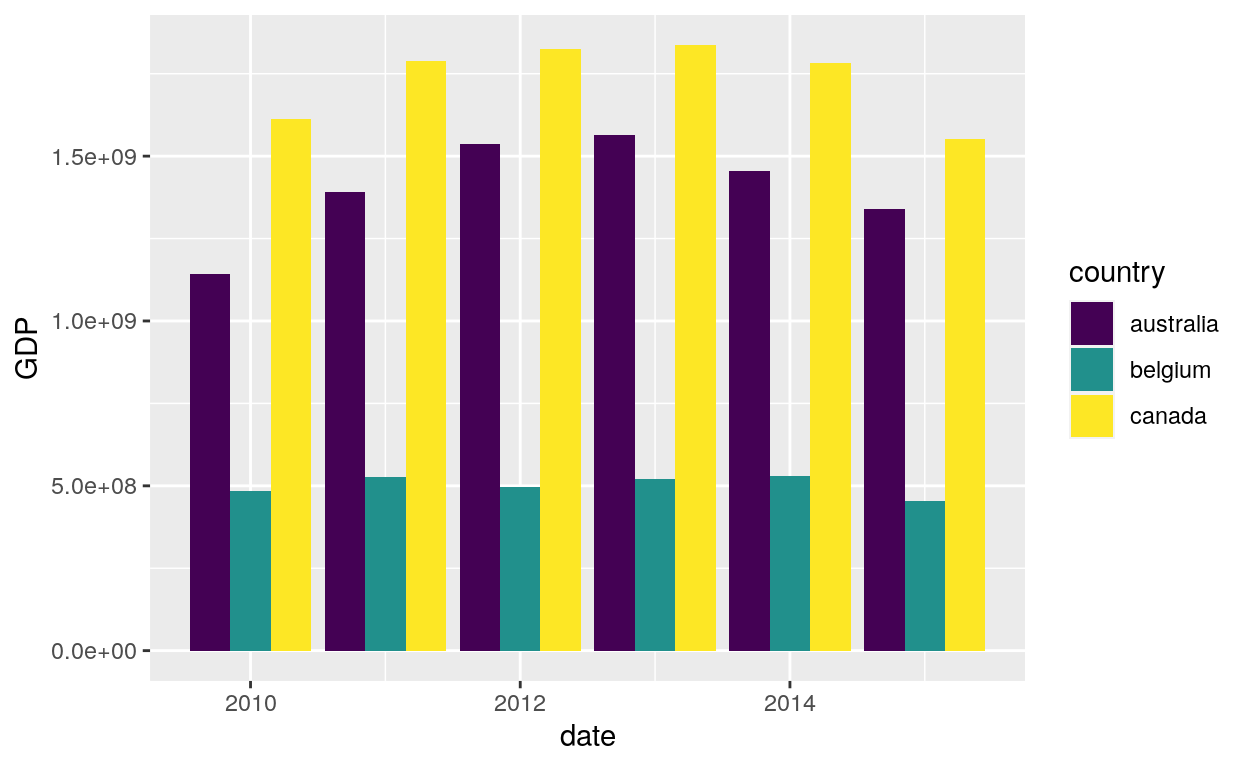##### Direction
``````ggplot(dataGraph, aes(date, GDP, fill = country)) +
geom_bar(stat = "identity", position = "dodge") +
scale_fill_viridis_d(direction = -1)
``````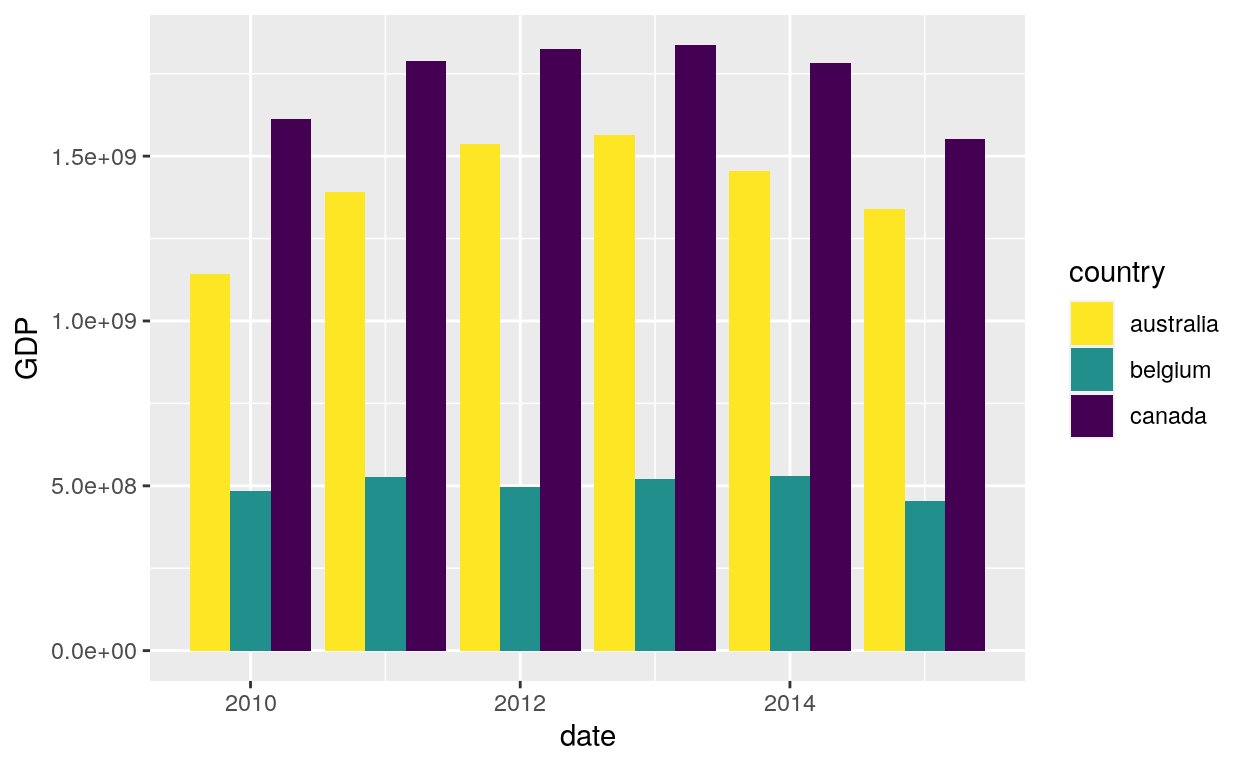##### Magma
``````ggplot(dataGraph, aes(date, GDP, fill = country)) +
geom_bar(stat = "identity", position = "dodge") +
scale_fill_viridis_d(option = "magma")
``````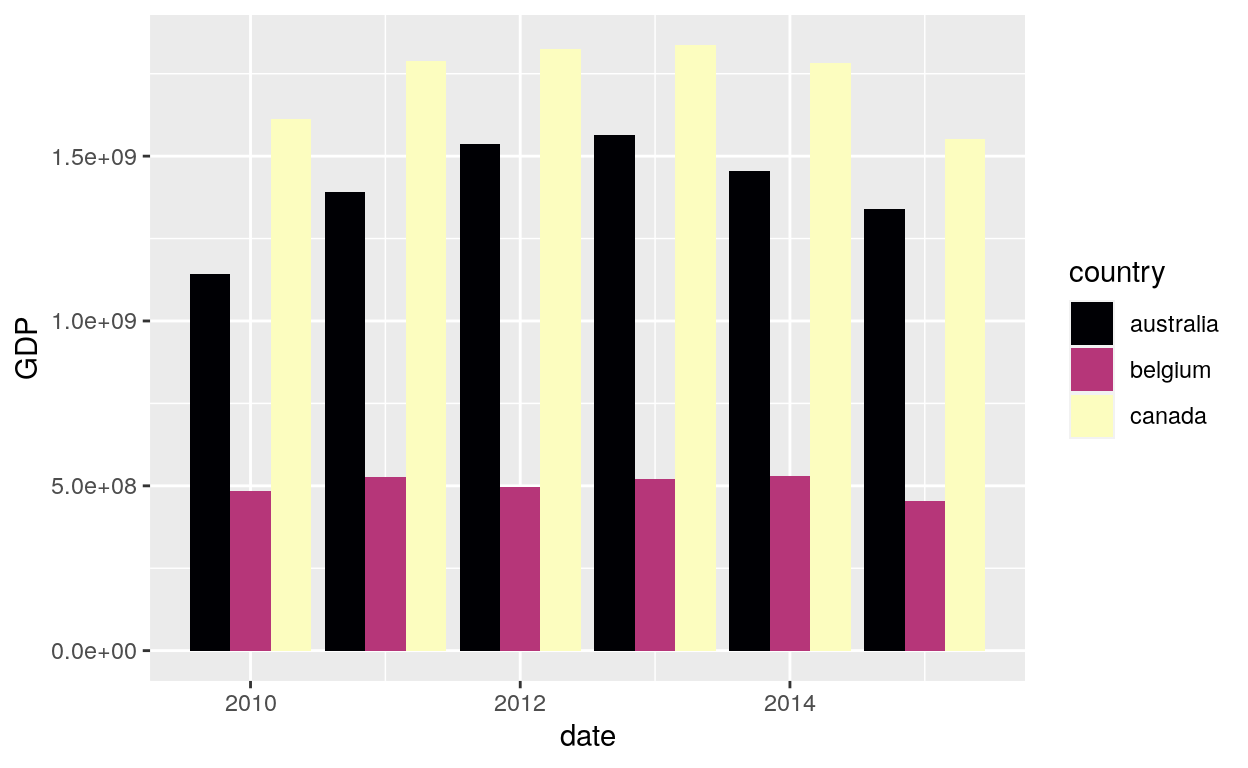##### Cividis
``````ggplot(dataGraph, aes(date, GDP, fill = country)) +
geom_bar(stat = "identity", position = "dodge") +
scale_fill_viridis_d(option = "cividis")
``````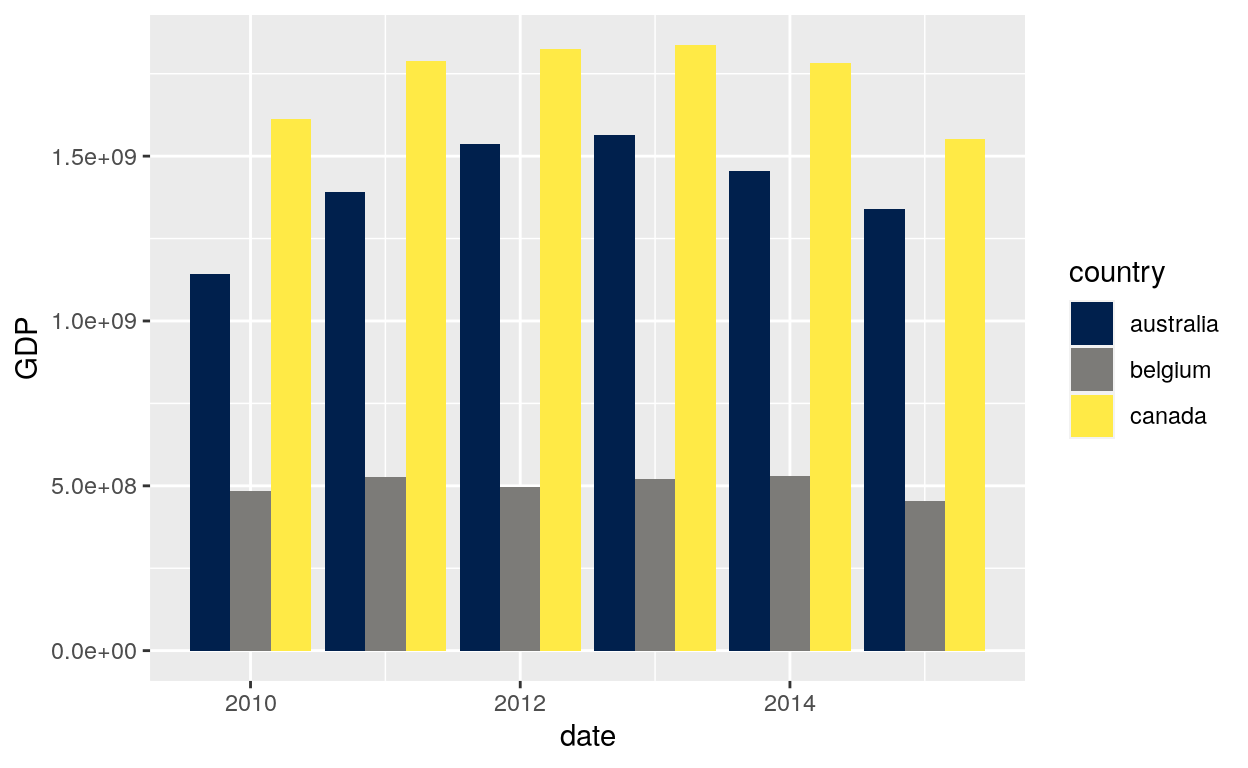### Line patterns

Default line types based on a set supplied by Richard Pearson, University of Manchester. Continuous values can not be mapped to line types.

#### Aes linetype

``````ggplot(dataGraph, aes(date, GDP)) +
geom_line(aes(group = country, linetype = country))
``````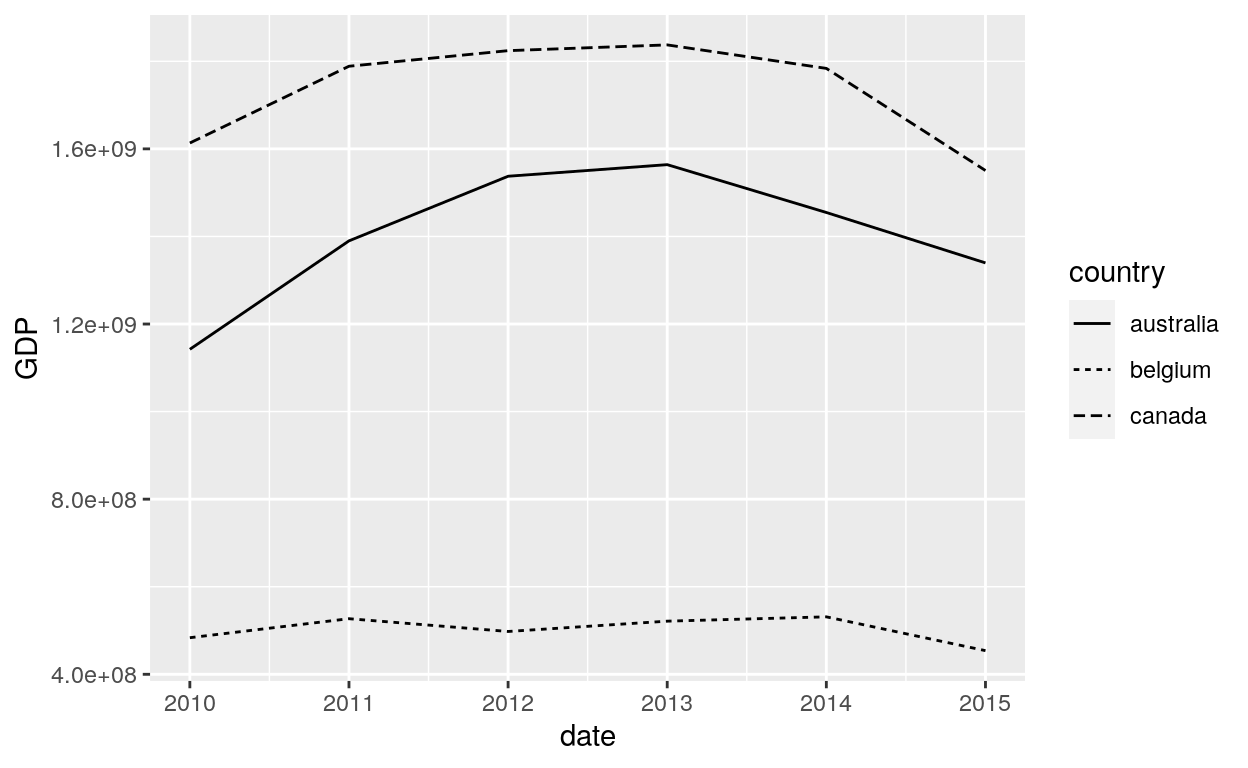#### Colored line type

``````ggplot(dataGraph, aes(date, GDP, colour = country)) +
geom_line(aes(group = country, linetype = country))
``````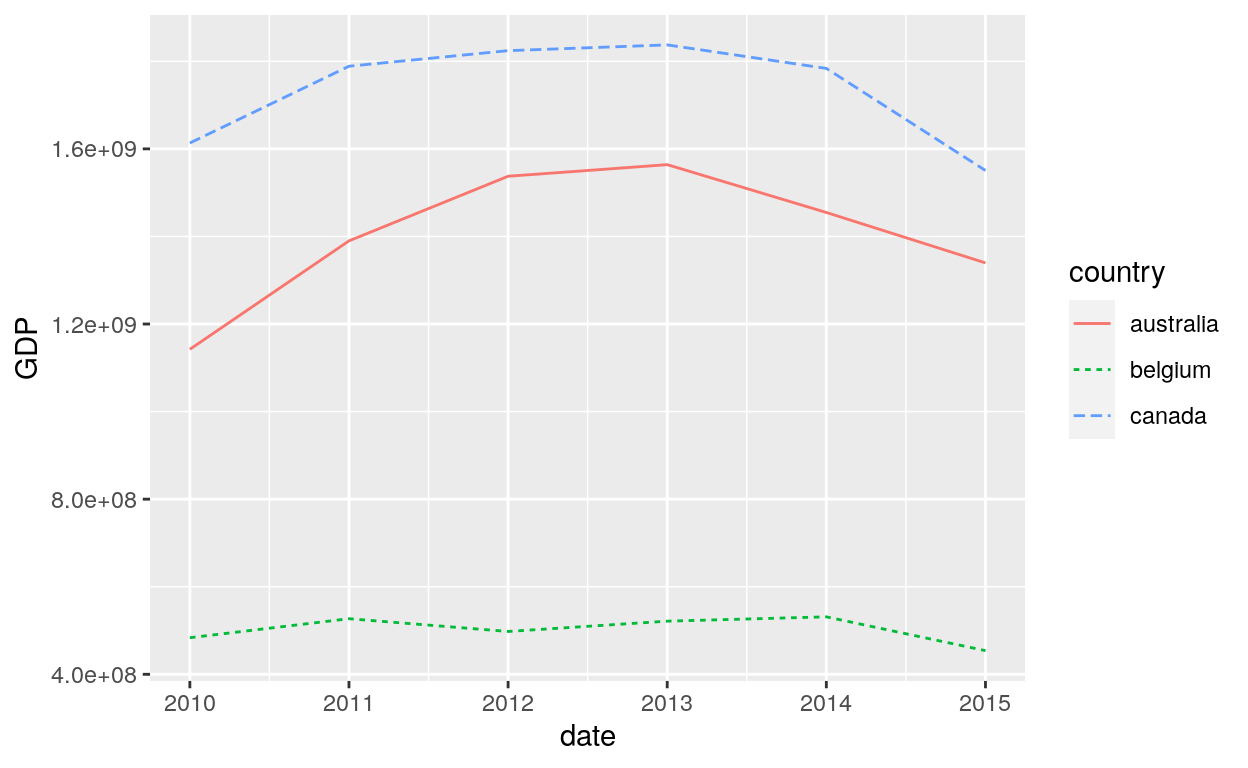#### Common line types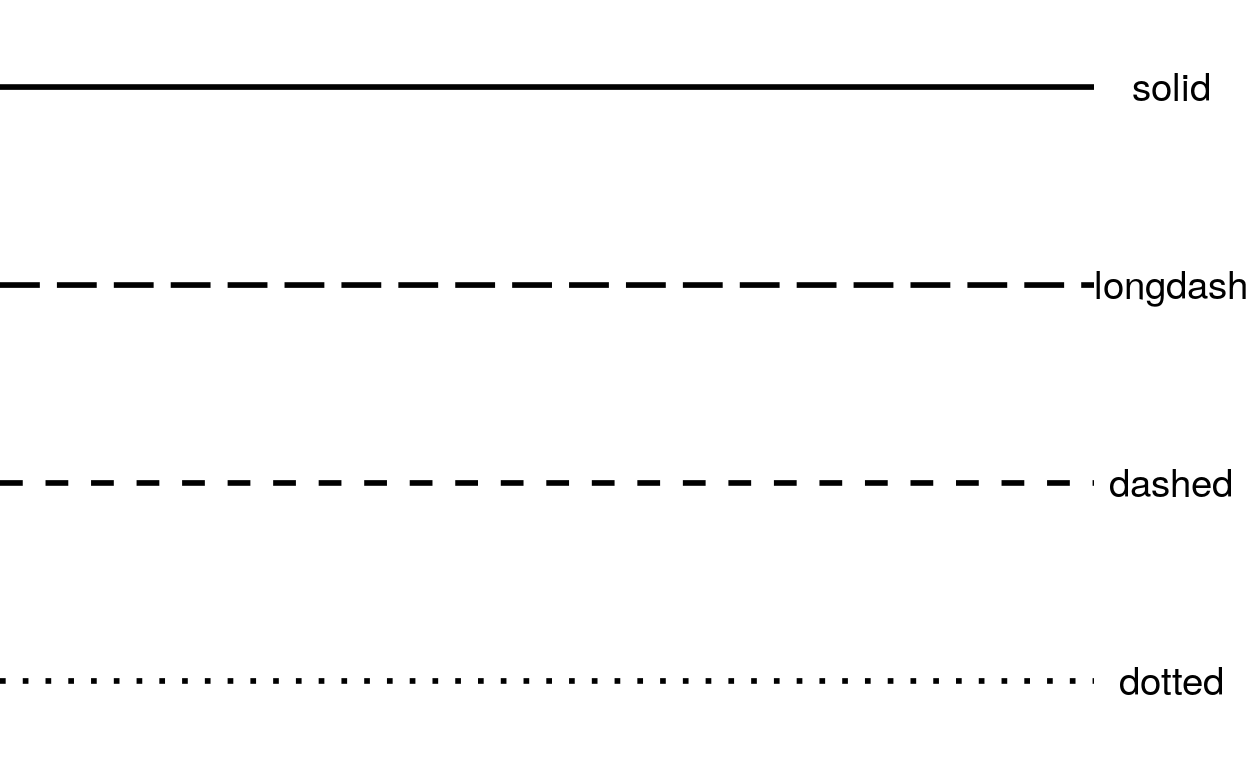### Shapes

scale_shape maps discrete variables to six easily discernible shapes (aka glyphs). If you have more than six levels, you will get a warning message, and the seventh and subsequence levels will not appear on the plot. Use scale_shape_manual() to supply your own values. You can not map a continuous variable to shape.

#### Shape

``````ggplot(dataGraph, aes(GDP, sample)) +
geom_point(aes(shape = country))
``````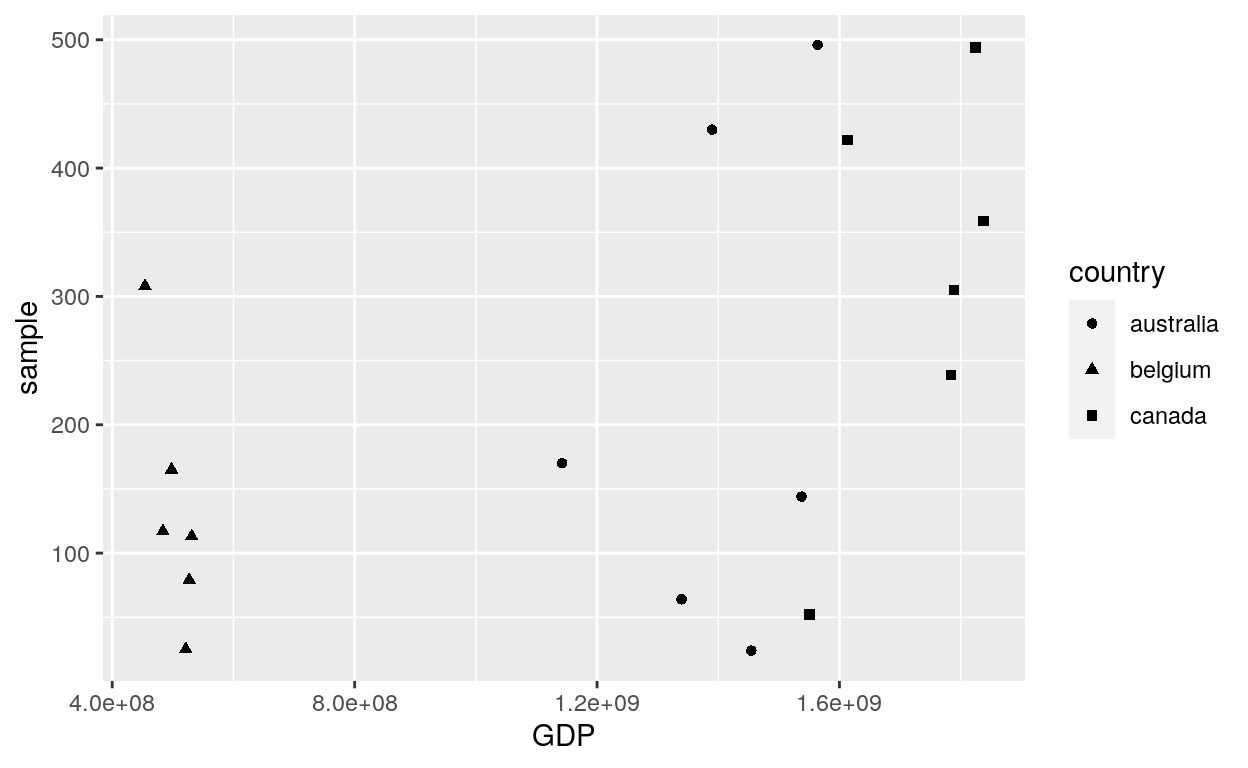#### Solid

``````ggplot(dataGraph, aes(GDP, sample)) +
geom_point(aes(shape = country)) +
scale_shape(solid = FALSE)
``````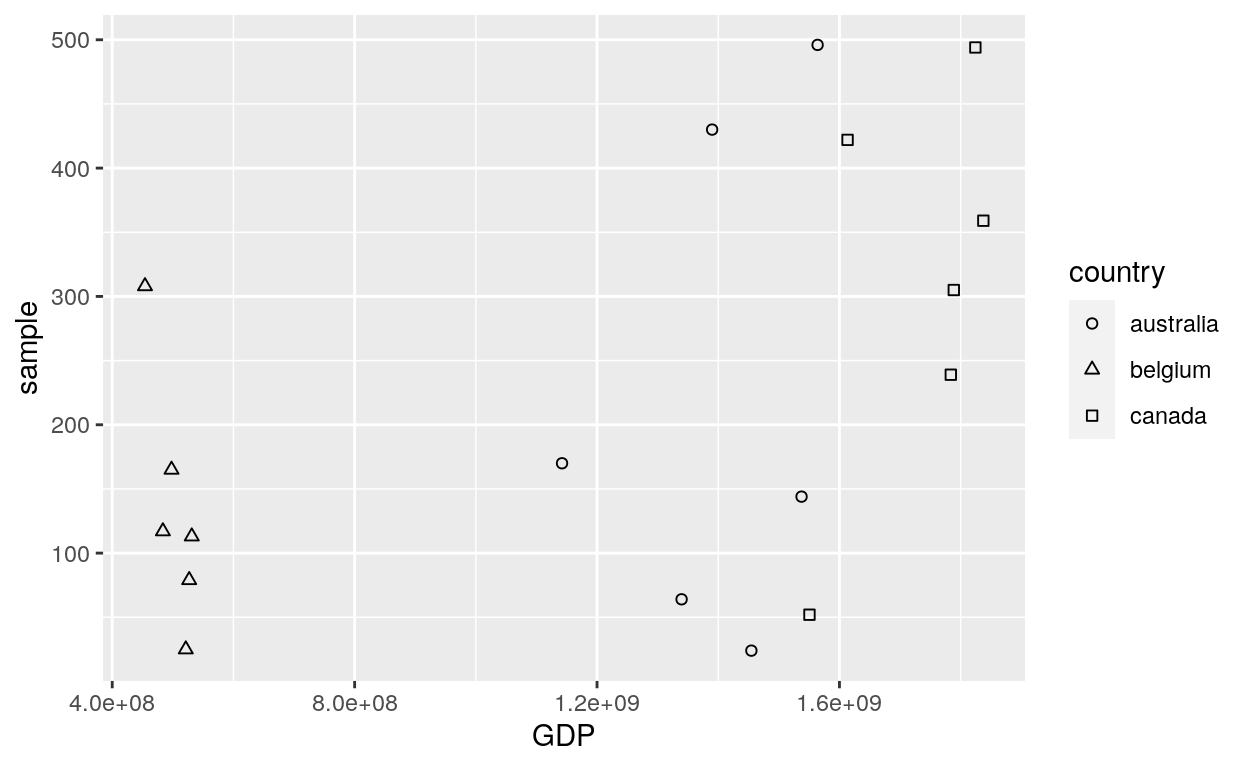#### Legend

``````# Change the name of the legend
ggplot(dataGraph, aes(GDP, sample)) +
geom_point(aes(shape = country)) +
scale_shape(name = "Countries")
``````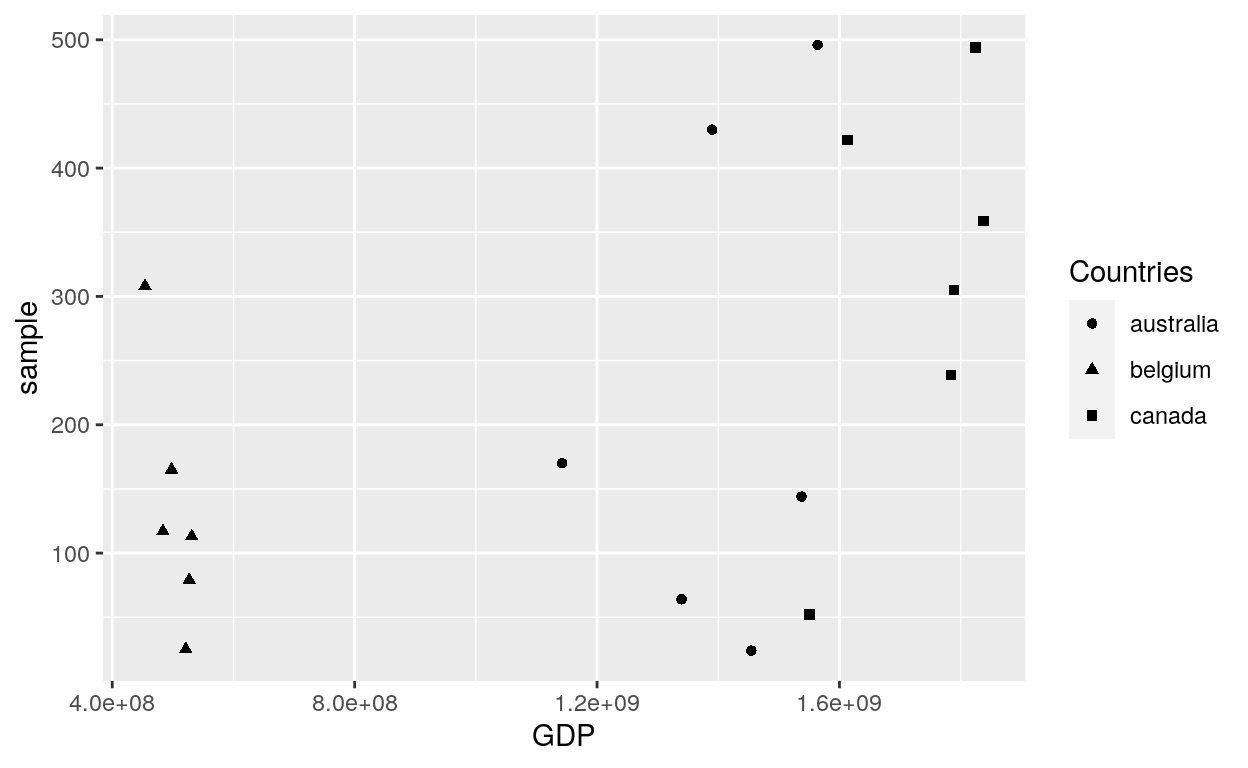#### Coloured shapes

``````ggplot(dataGraph, aes(GDP, sample)) +
geom_point(aes(shape = country, colour = country)) +
scale_shape(name = "Countries")
``````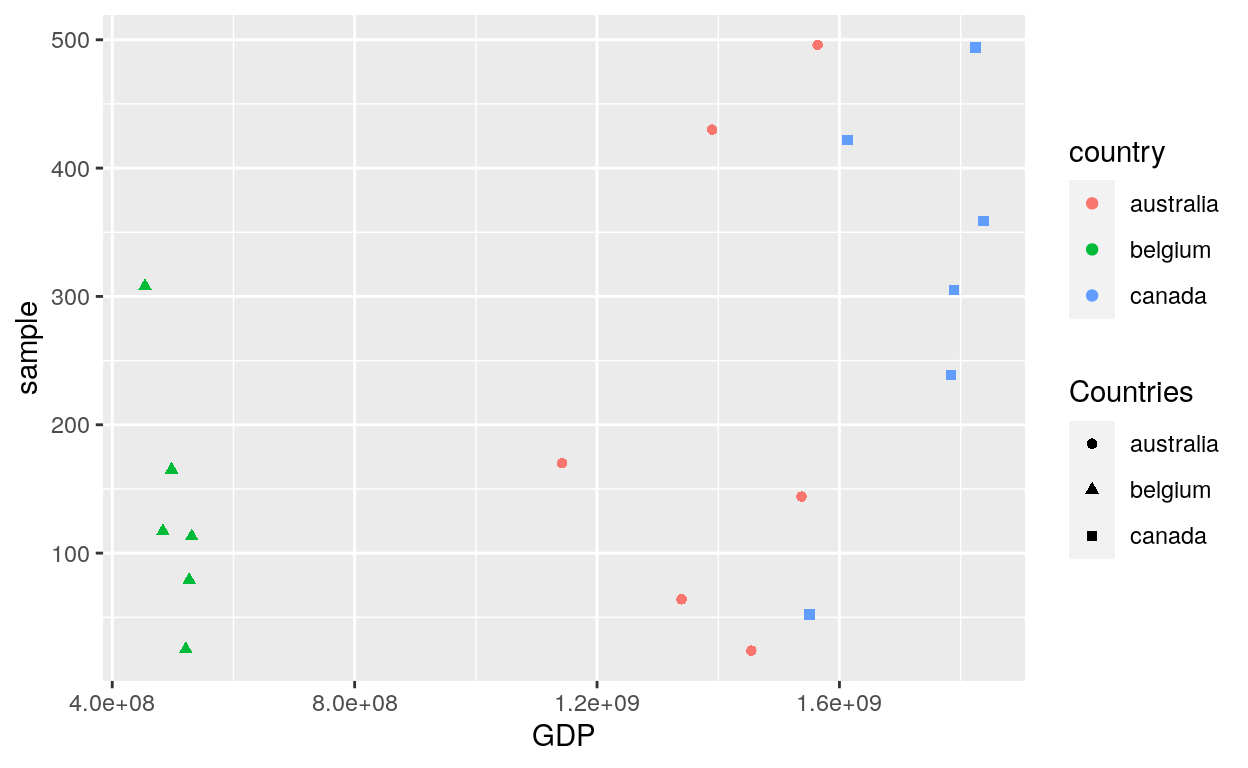``````ggplot(dataGraph, aes(date, GDP, colour = country)) +
geom_line(aes(group = country, linetype = country)) +
geom_point(aes(colour = country, shape = country))
``````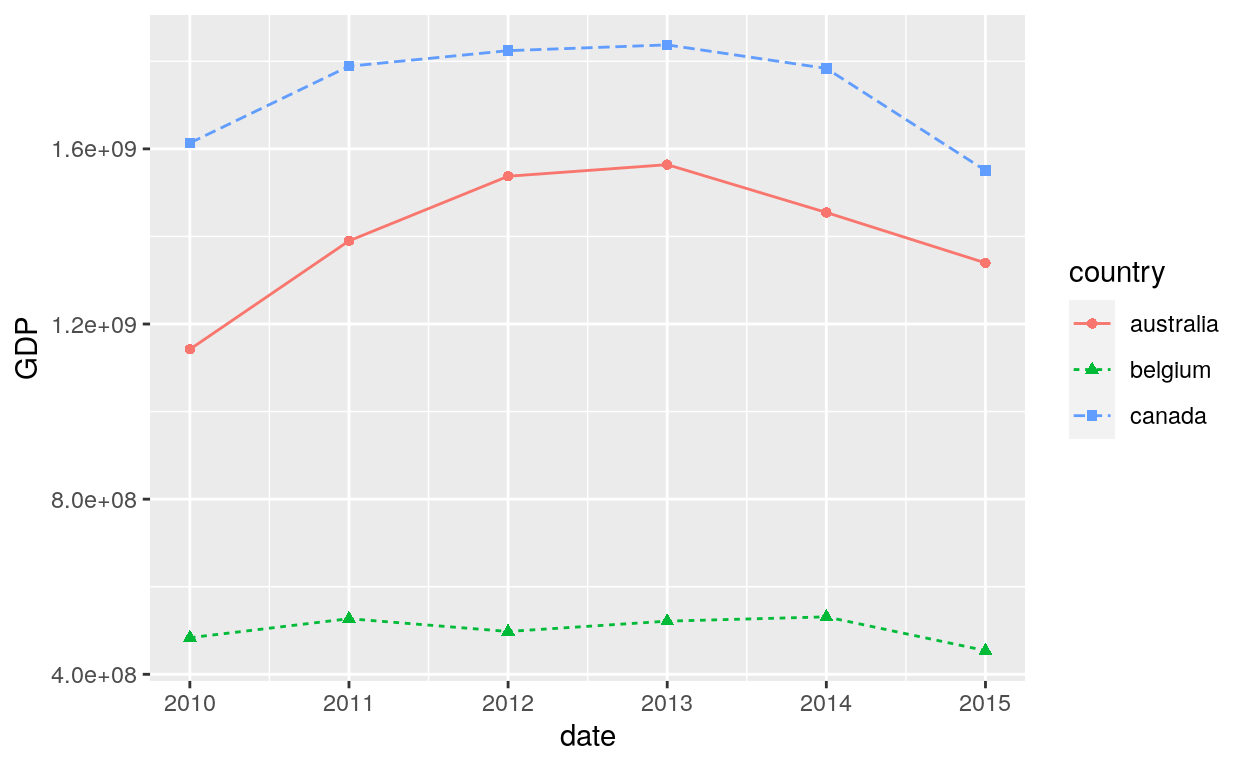#### Size

• Fixed size
``````ggplot(dataGraph, aes(GDP, sample)) +
geom_point(aes(shape = country, colour = country), size = 5)
``````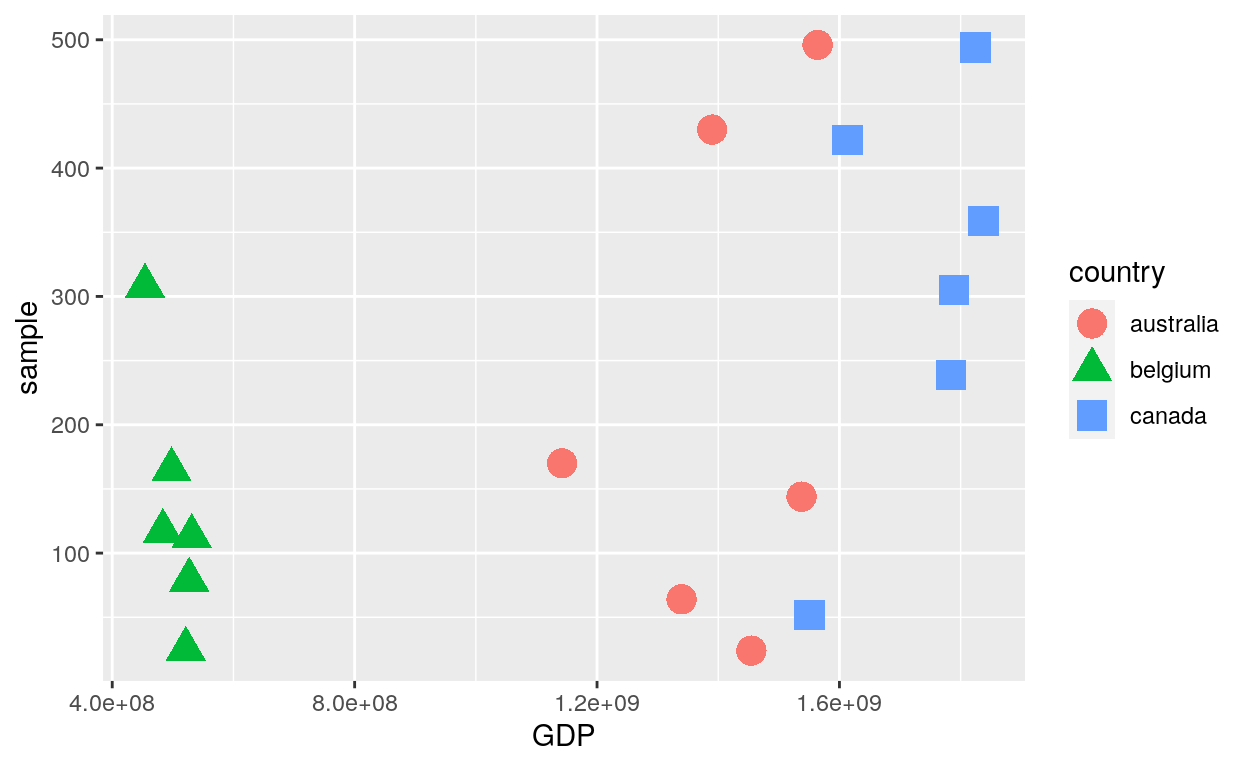``````ggplot(dataGraph, aes(date, GDP, colour = country)) +
geom_line(aes(group = country, linetype = country)) +
geom_point(aes(colour = country, shape = country), size = 3)
``````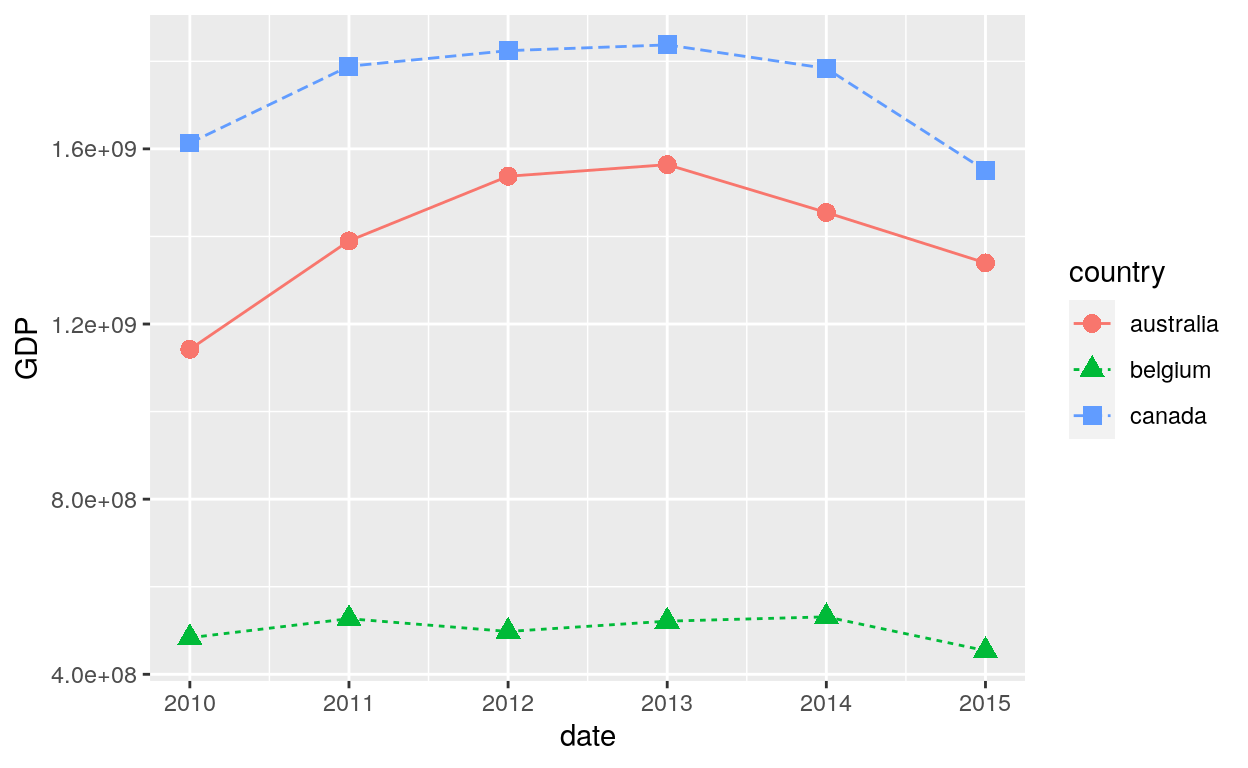• Aes size
``````ggplot(dataGraph, aes(GDP, sample)) +
geom_point(aes(shape = country, colour = country, size = GDP))
``````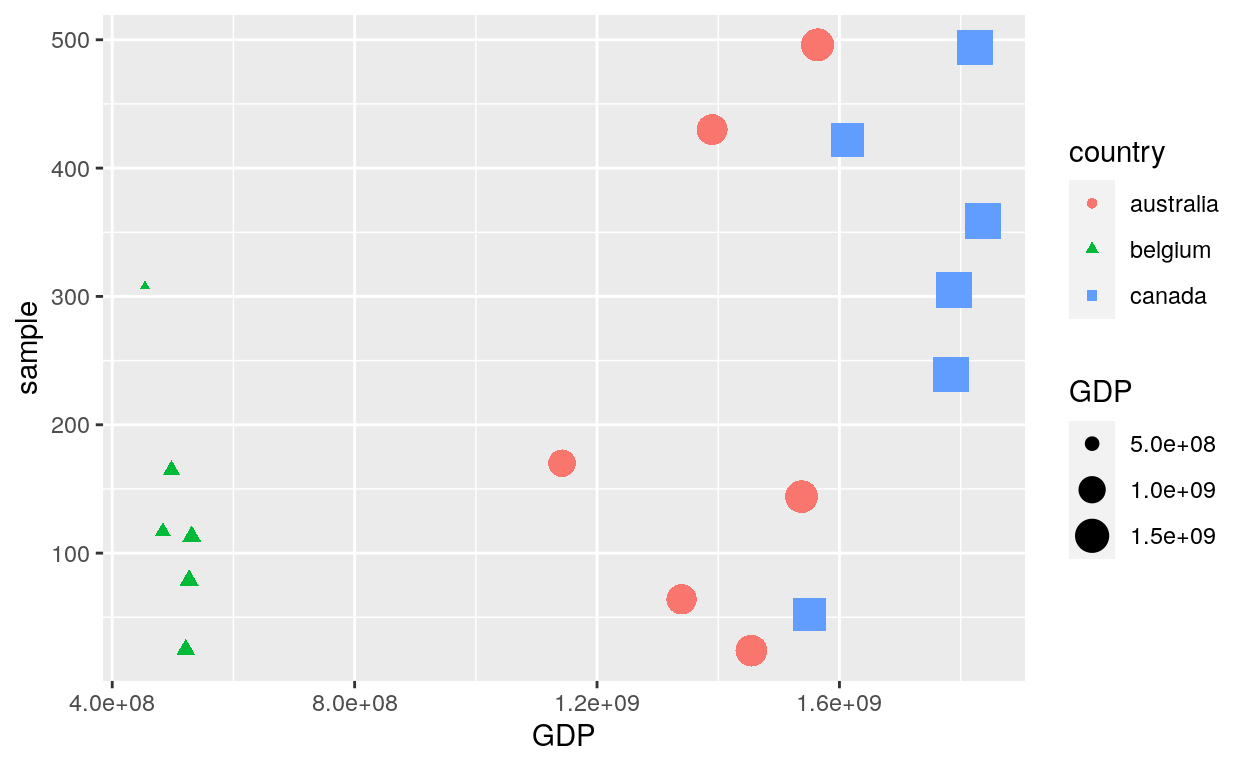``````ggplot(dataGraph, aes(date, GDP, colour = country)) +
geom_line(aes(group = country, linetype = country)) +
geom_point(aes(colour = country, shape = country, size = GDP))
``````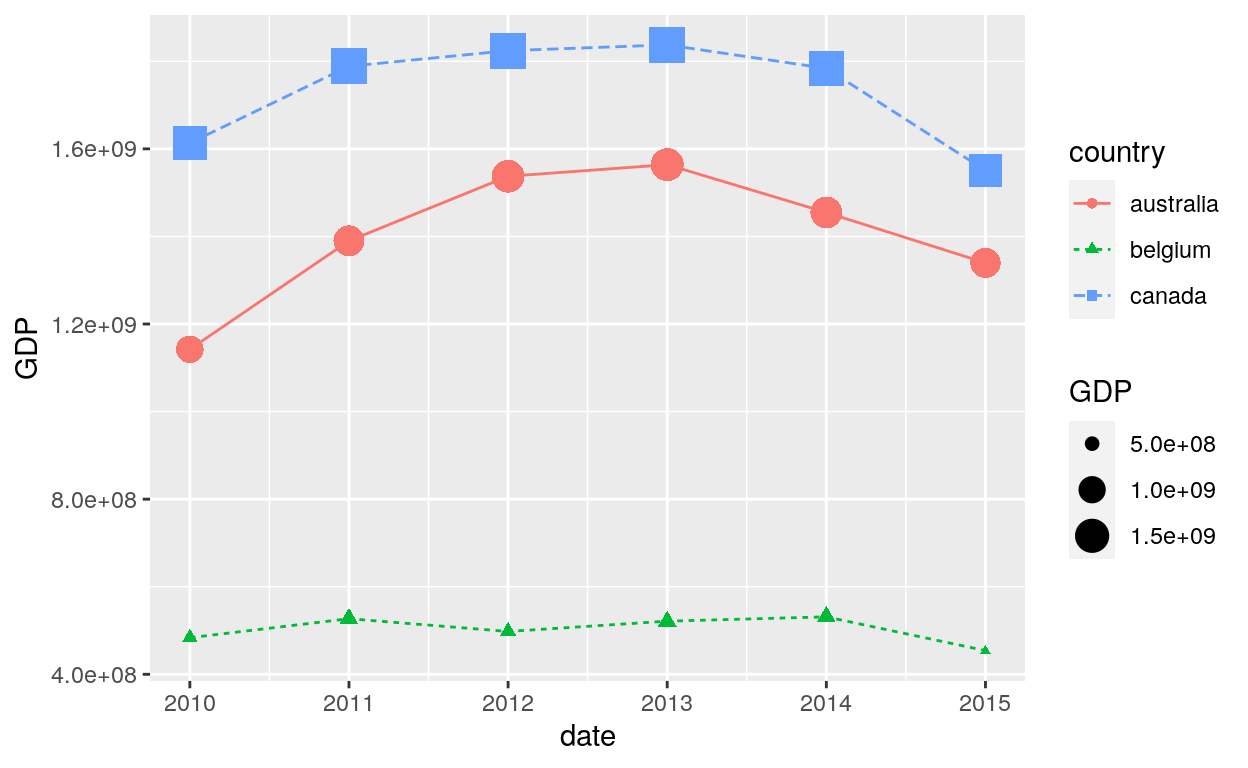#### List of all shapes

``````df_shapes <- data.frame(shape = 0:24)
ggplot(df_shapes, aes(0, 0, shape = shape)) +
geom_point(aes(shape = shape), size = 5, fill = 'red') +
scale_shape_identity() +
facet_wrap(~shape) +
theme_void()
``````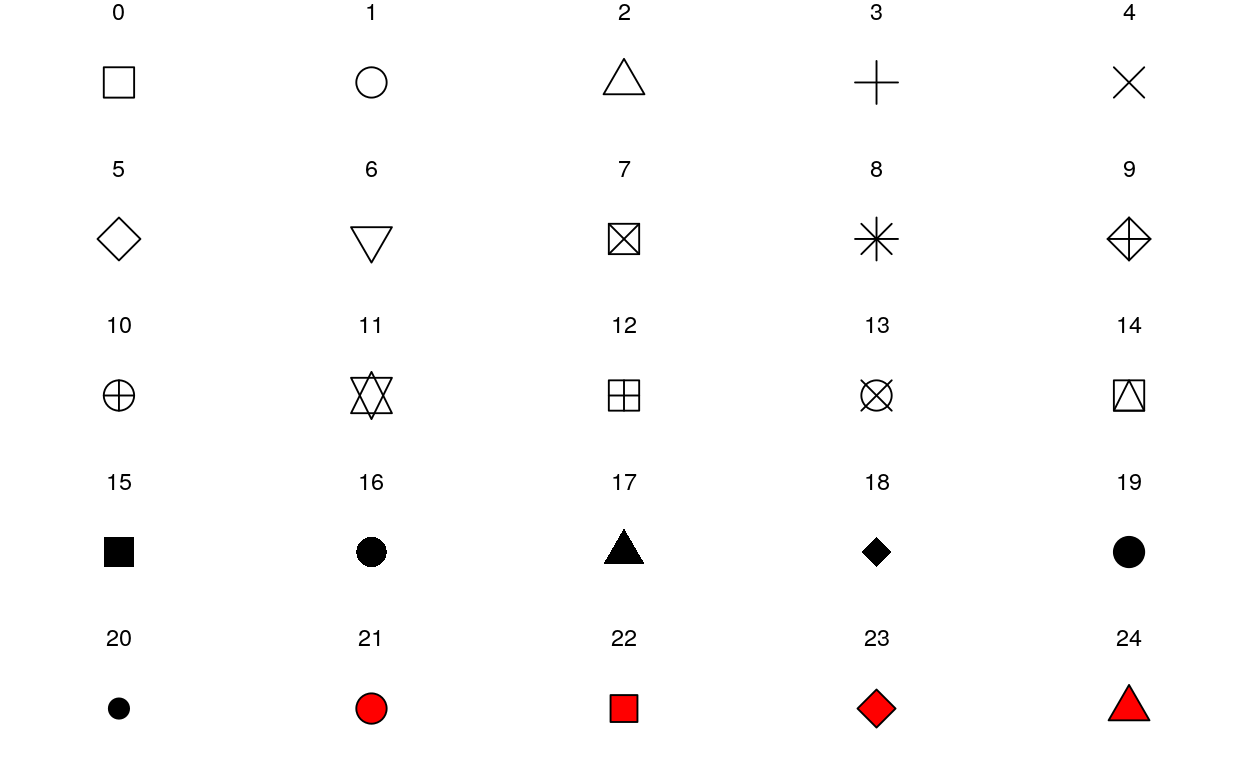### Citation

`Warin (2019, Aug. 6). Thierry Warin, PhD: [R Course] Data Visualisation with R: Options. Retrieved from https://warin.ca/posts/rcourse-datavisualizationwithr-options/`
```@misc{warin2019[r,